# Chapter 3 Logical and Lookup Functions

Going beyond the basic computational tools of Excel unlocks unlimited potential for processing and analyzing data. This chapter takes you beyond basic Excel computations by introducing logical functions and lookup functions. Logical functions are used to assess the contents within cell locations and produce custom outputs or mathematical computations. We will look at two types of logical functions in this chapter. The first is the IF function, which uses a logical test to evaluate the contents in a cell location. The OR and AND functions also use logical tests to evaluate the contents in a cell location and are often used within the IF function. The second type of logical function that we will look at are statistical IF functions. These functions combine the logical test feature of the IF function with the mathematical computation features of statistical functions (which were reviewed in Chapter 2 "Mathematical Computations"). The last section of this chapter demonstrates the use of two lookup functions. These functions are used to find data in one worksheet and display it in another. We will demonstrate the skills for this chapter through the construction of a personal investment portfolio. This theme builds on the personal budget project introduced in Chapter 2 "Mathematical Computations". In the personal budget project, we analyzed the impact of investing money in an account that provides a continuous rate of return. In this chapter we look at how you can decide where to invest your money and how to analyze the performance of those investments.

## 3.1 Logical Functions

### Learning Objectives

1. Learn how to use the Freeze Panes command to lock specific columns and rows in place while scrolling through large worksheets.
2. Understand the construction and use of formulas, basic statistical functions, and financial functions.
3. Learn how to construct a logical test to evaluate the contents of a cell location.
4. Learn how to use the IF function to evaluate the data in a cell location using a logical test.
5. Learn how to use the OR function within an IF function to evaluate the data in a cell location using multiple logical tests.
6. Learn how to use the AND function within an IF function to evaluate the data in a cell location using multiple logical tests.
7. Review the construction of nested IF functions for evaluating data using more than one logical test.
8. Learn how to set a conditional format rule so formatting commands are automatically applied based on the value in a cell location.

This section reviews the use of logical functions in Excel through the construction of an investment portfolio. Although it may seem that managing investments is a specialized career choice, the reality is that almost everyone will become an investor at some point in their lives. Many companies offer employees retirement savings benefits through 401(k) or 403(b)Employee retirement savings plans offered by businesses and by public and private institutions. These plans allow you to deduct money from your paycheck every month, tax-free, and invest it. plans. These plans allow you to deduct money from your paycheck every month, tax-free, and invest it. In addition to the tax benefits afforded by such plans, many employers match a percentage of your monthly savings or deposit money into your retirement account as an added form of compensation. When you sign up for these savings plans, your company will give you a list of options as to how your money can be invested, and you choose the type of investments you would like the company to make on your behalf. As a result of this process, you become an investor. Excel can be an extremely valuable tool to help you make these investment decisions and analyze the performance of the money you have invested.

Figure 3.1 "Completed Personal Investment Portfolio Workbook" shows the completed investment portfolio workbook that we will complete in this chapter. Similar to the personal budget example in Chapter 2 "Mathematical Computations", the Portfolio Summary worksheet contains a summary of the data entered or calculated in other worksheets in the workbook. This project begins by building on the Investment Detail worksheet.

Figure 3.1 Completed Personal Investment Portfolio Workbook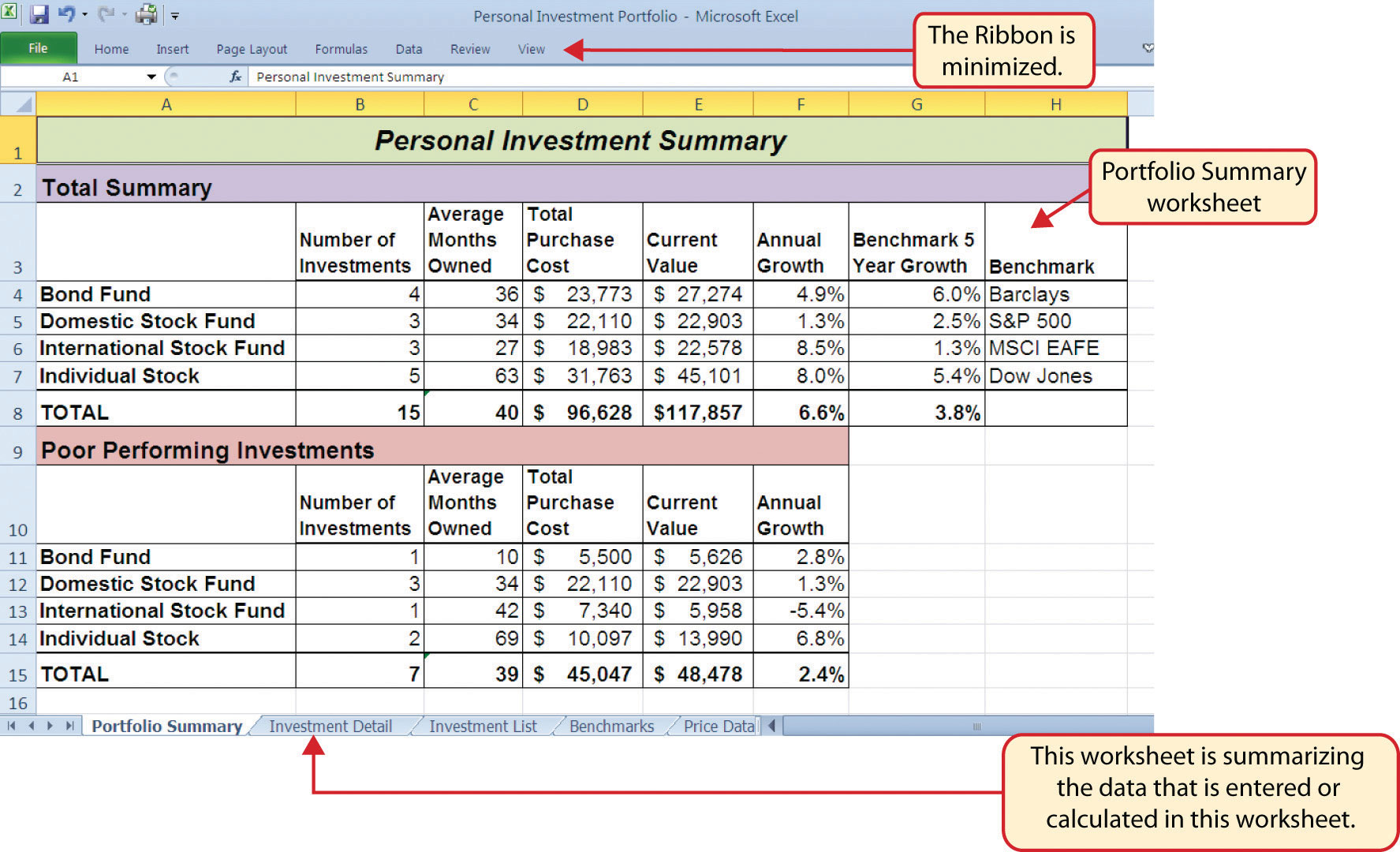## Freeze Panes

Follow-along file: Excel Objective 3.00

### Lesson Video: Freeze Panes

The Investment Detail worksheet shown in Figure 3.2 "Investment Detail Worksheet" contains the majority of the information used to create the Portfolio Summary worksheet shown in Figure 3.1 "Completed Personal Investment Portfolio Workbook". When you first open the worksheet, you will notice it is not possible to view all twenty-four columns on your computer screen. As you scroll to the right to view the rest of the columns, you will lose site of the row headings in Columns A and B. The headings in these columns show the investment that pertains to the data in Columns C through X. To solve this problem of viewing the row headings while scrolling through the remaining columns in the worksheet, we will use the Freeze Panes command.

Figure 3.2 Investment Detail Worksheet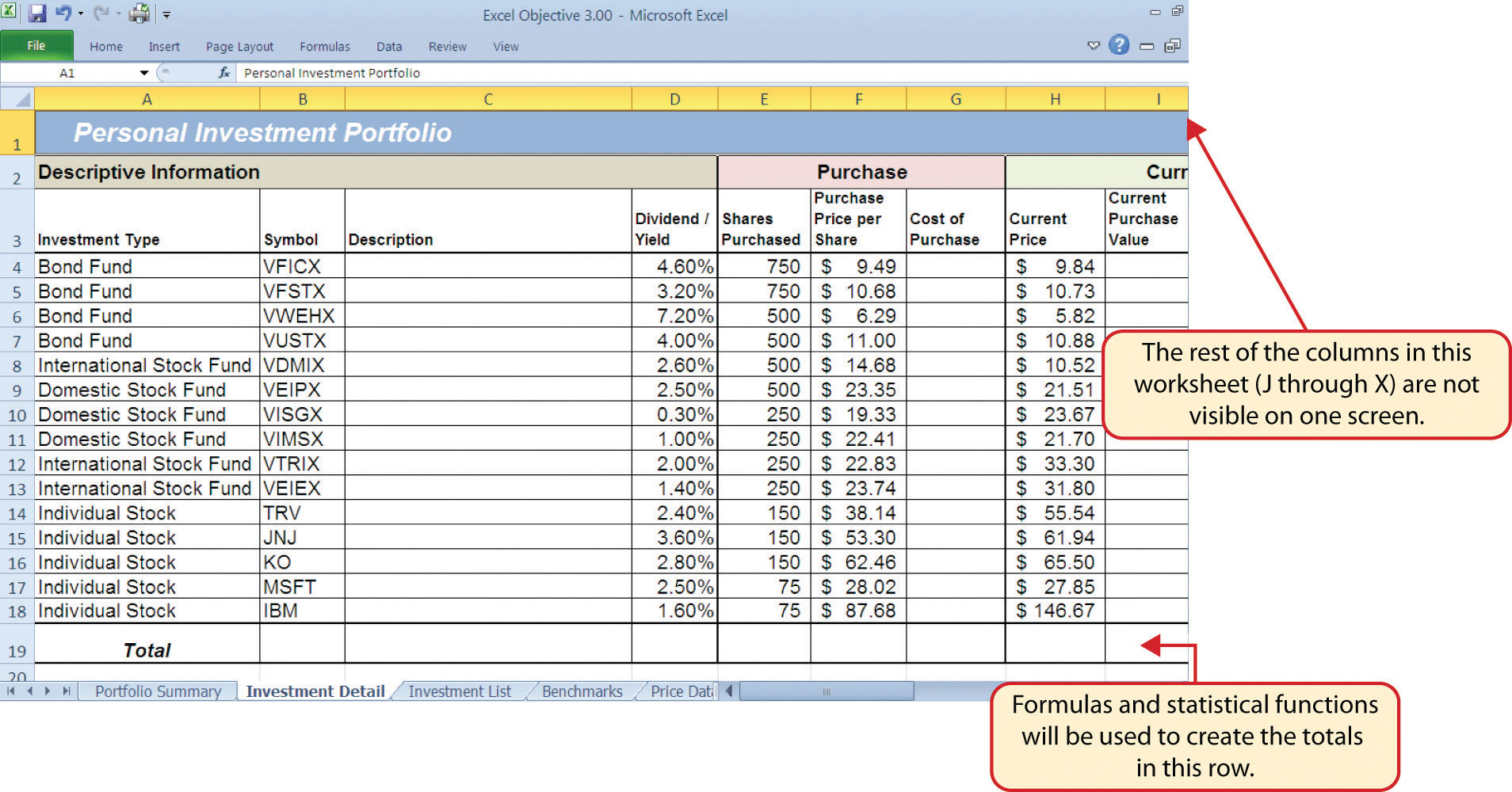The Freeze PanesAn Excel command that allows you to lock specific columns and rows in place while scrolling through a large worksheet. command allows you to scroll across the Investment Detail worksheet while keeping the row headings in Columns A and B locked in place. The following steps explain how to do this:

1. Click cell C4 on the Investment Detail worksheet. We select this cell because the Freeze Panes option locks the columns to the left of the activated cell as well as the rows above the activated cell.
2. Click the View tab on the Ribbon.
3. Click the Freeze Panes button (see Figure 3.3 "Freeze Panes Command").
4. Click the Freeze Panes option from the drop-down list of options.

Figure 3.3 Freeze Panes Command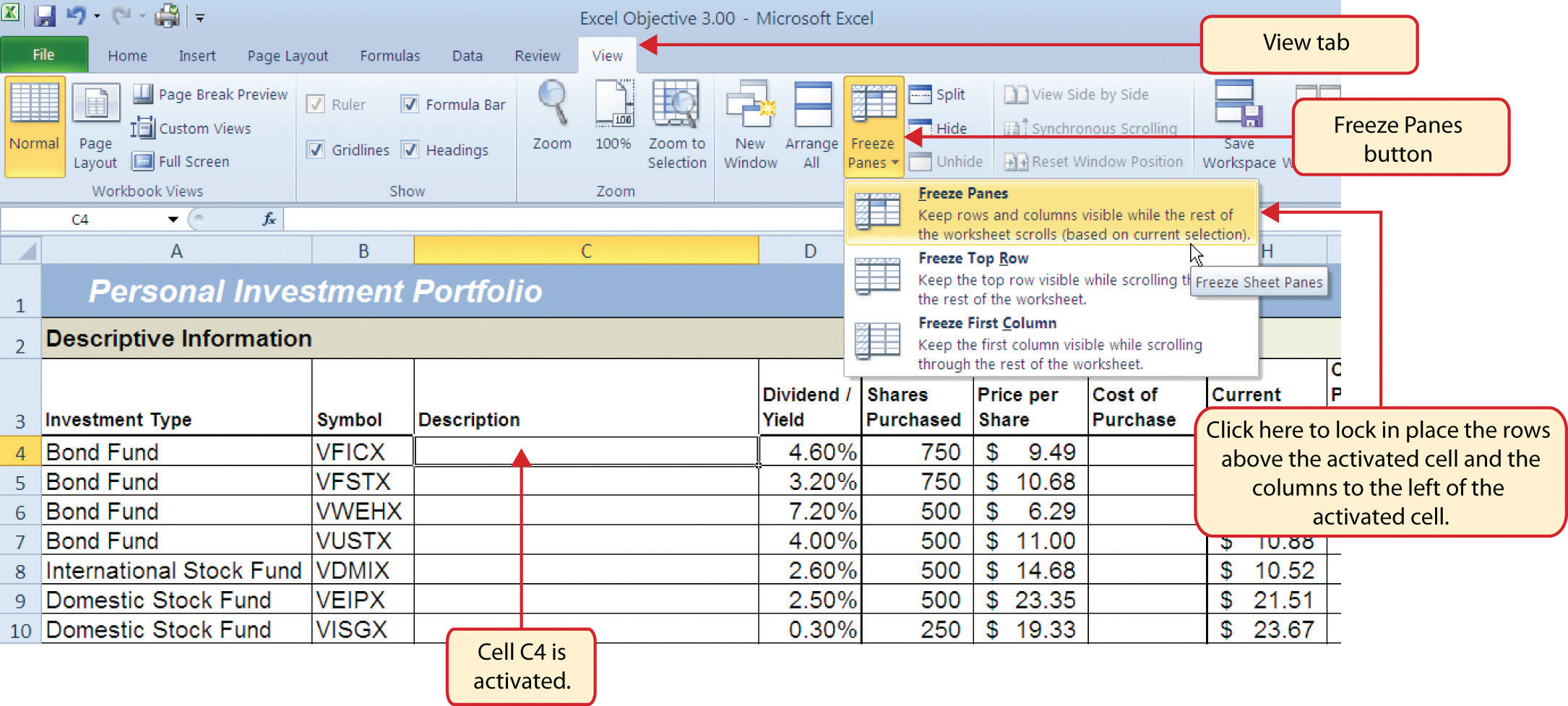Once you click the Freeze Panes option shown in Figure 3.3 "Freeze Panes Command", Columns A and B are locked in place as you scroll through the columns in the worksheet. Since this is a large worksheet, you may find it easier to navigate the columns by using the arrow keys on your keyboard. However, since rows 1 and 2 contain merged cells, make sure a cell location is activated below Row 2 before you begin using the arrow keys. Figure 3.4 "Freeze Panes Command Activated on the Investment Detail Worksheet" shows the appearance of the Investment Detail worksheet after the Freeze Panes command has been activated. To deactivate the Freeze Panes command, click the Freeze Panes button again and select the Unfreeze Panes option.

Figure 3.4 Freeze Panes Command Activated on the Investment Detail Worksheet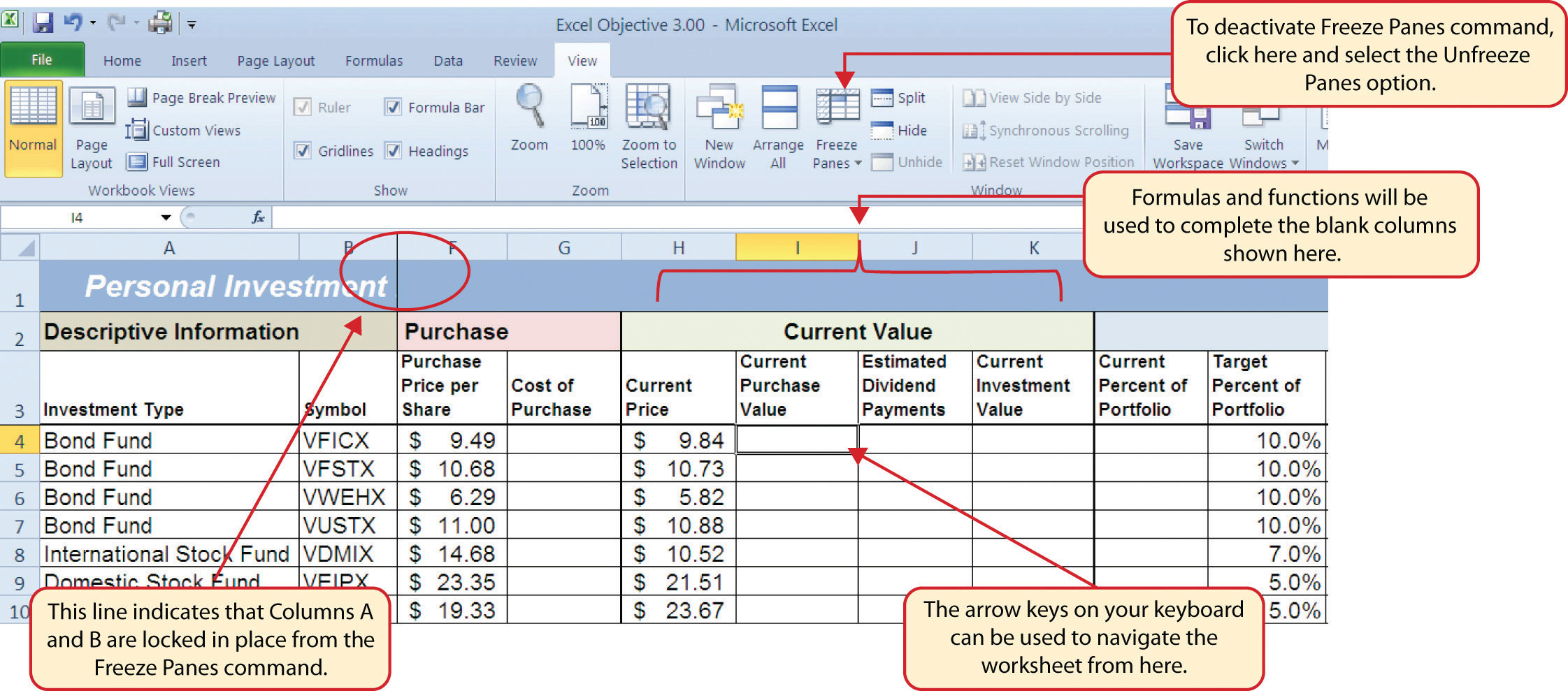## Formula and Functions Review

Follow-along file: Continue with Excel Objective 3.00. (Use file Excel Objective 3.01 if starting here.)

### Lesson Video: Formulas and Functions Review

We will begin developing the personal investment portfolio workbook by adding several formulas and functions. The formulas and functions we will add were illustrated in detail in Chapter 2 "Mathematical Computations". Therefore, the steps provided in this chapter will be brief. After the formulas and functions are added to the Investment Detail worksheet, we can add the logical and lookup functions. However, before proceeding, let’s review the investment type definitions in Table 3.1 "Investment Types in Column A of the Investment Detail Worksheet". Table 3.1 "Investment Types in Column A of the Investment Detail Worksheet" provides a definition for each of the investment types listed in Column A of the Investment Detail worksheet. This project assumes that the personal investment portfolio comprises four types of investments. The reason we include a variety of investment types in any portfolio is to manage our total risk, or potential of losing money. When building an investment portfolio, it is important to keep in mind that investments of all types can dramatically increase or decrease in value over a short period of time. Managing risk requires that your money is not concentrated in one type of investment.

Table 3.1 Investment Types in Column A of the Investment Detail Worksheet

Category Definition
Bond Fund A mutual fund consisting of a variety of bonds. The benefit of buying shares of a fund as opposed to a specific bond is that doing so allows you to spread your investment over several bonds instead of concentrating your investment in just one bond.
Domestic Stock Fund A mutual fund consisting of several domestic stocks. Buying shares of a stock mutual fund provides the benefit of investing your money over several stocks.
International Stock Fund Same as a domestic stock fund but contains a variety of non-US or foreign stocks.
Individual Stock The stock for one specific company. In addition to mutual funds, this chapter’s portfolio will include a few individual stocks for public companies. When you purchase shares of a specific company, such as IBM, you become a partial owner of that company.

We will begin adding formulas and functions to the Investment Detail worksheet in sections. If you scroll across all the columns in the worksheet, you will notice the worksheet includes five distinct sections. Four of the five sections contain columns that need to be completed with formulas and functions before we can add the logical and lookup functions. Table 3.2 "Definitions for Columns A through G of the Investment Detail Worksheet" contains definitions for each of the columns in the Descriptive Information section (Columns A through D) and the Purchase section (Columns E through G). It will be helpful to understand the purpose of these columns as we complete this worksheet.

Table 3.2 Definitions for Columns A through G of the Investment Detail Worksheet

Category Definition
Investment Type The type of investment with regard to bonds and stocks. A definition for each of the investment types used in this portfolio can be found in Table 3.1 "Investment Types in Column A of the Investment Detail Worksheet".
Symbol The symbol that represents a mutual fund or stock. This symbol can be used to research the profile or current trading price on any website that provides stock quotes.
Description The company name for an individual stock or a description of the type of investments made by a mutual fund.
Dividend/Yield The amount of interest earned on a bond or bond fund or the amount of earnings distributed per share for an individual stock or stock fund.
Shares Purchased The amount of shares purchased for a mutual fund or individual stock.
Purchase Price per Share The price paid for the shares purchased for the mutual funds and individual stocks in the portfolio.
Cost of Purchase The number of shares purchased multiplied by the purchase price per share. This represents your base investment and is used to determine how much money has been gained or lost.

The Descriptive Information section of the Investment Detail worksheet (Columns A through D) contains only one blank column, which will be completed using a lookup function. Therefore, we will proceed to the Purchase section (Columns E through G) where the Cost of Purchase column is blank. The following steps explain how to enter the formula into this column:

1. Click cell G4 on the Investment Detail worksheet.
2. Type an equal sign (=).
3. Enter a formula that multiplies the Shares Purchased (cell E4) by the Purchase Price per Share (cell F4).
4. Copy the formula in cell G4.
5. Highlight the range G5:G18.
6. Click the down arrow on the Paste button in the Home tab of the Ribbon.
7. Click the Formulas button from the list of options. This is the Paste Formulas command, which pastes only the formula without any associated formats for the copied cell location.
8. Click cell E19 on the Investment Detail worksheet.
9. Press and hold the ALT key on your keyboard, then press the equal sign (=). This is the shortcut for the Auto Sum feature.
10. Press the ENTER key on your keyboard.
11. Click cell G19 on the Investment Detail worksheet.
12. Repeat step 9.
13. Press the ENTER key on your keyboard.

Figure 3.5 "Completed Formula in the Cost of Purchase Column" shows the formula that was entered into cell G4 in the Purchase section of the Investment Detail worksheet. You can also see the results of the formula after it is pasted into the range G5:G18. The Paste Formulas option was used to paste the formula into this range so the borders would not be altered.

Figure 3.5 Completed Formula in the Cost of Purchase Column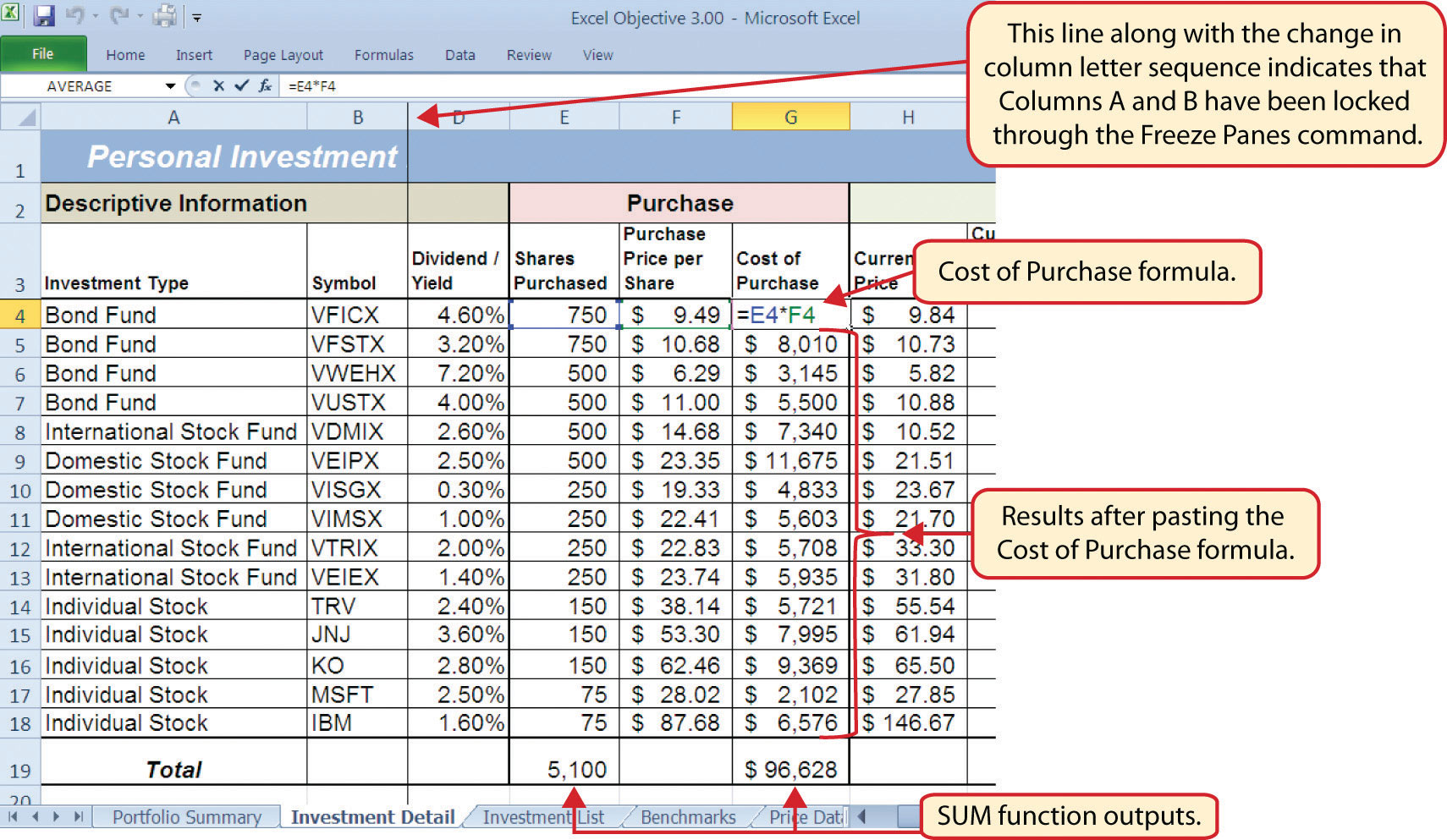Table 3.3 "Definitions for Columns H through K of the Investment Detail Worksheet" shows the definitions for the Current Value section (Columns H through K) of the Investment Detail worksheet.

Table 3.3 Definitions for Columns H through K of the Investment Detail Worksheet

Category Definition
Current Price The current price of an individual stock or the current net asset value of a mutual fund.
Current Purchase Value The number of shares purchased multiplied by the current price.
Estimated Dividend Payments The estimated amount of money paid for the interest on a bond fund or the dividends paid on a stock or stock fund. The future value function is used to estimate these payments. For an actual portfolio, real monetary distributions can be added to the current purchase value of the investment to calculate the total value of an investment.
Current Investment Value The current purchase value plus the estimated dividend payments. The current investment value is compared with the cost of purchase to determine how much money is gained or lost.

We will add a basic formula to the Current Purchase Value and Current Investment Value columns. For the Estimated Dividend Payments column, we will use the FV (future value) function to estimate the dividend payments. The following explains how we add the FV function to the Estimated Dividend Payments column:

1. Click cell J4 and type an equal sign (=).
2. Type the function name FV followed by an open parenthesis (().
3. Click cell D4, type a forward slash (/) for division, and then type 12. This divides the rate in the Dividend/Yield column by 12. The length of ownership of an investment is expressed in terms of months in Column Q. Therefore, the rate for the FV function must be expressed in terms of months by dividing the annual rate by 12.
4. Type a comma.
5. Click cell Q4, which contains the number of months owned or the term of the future value calculation.
6. Type a comma followed by a zero (,0). We are not calculating an annuity or periodic investment in this example, so the PMT argument will be defined with a zero. Type a comma to advance the function to the Pv argument.
7. Type a minus sign () and click cell G4. This is the cost of the investment purchase previously calculated.
8. Type a closing parenthesis ()).
9. Type a minus sign () and click cell G4. By itself, the FV function is calculating the total value of the investment with dividends or interest earned. To show only the amount of dividends or interest earned, we subtract the cost of the investment purchase in G4 from the result of the FV function.
10. Press the ENTER key on your keyboard.
11. Adjust the decimal places for the output of the FV function to zero.
12. Copy the FV function in cell J4 and paste it into the range J5:J18 using the Paste Formulas command.

Figure 3.6 "Completed FV Function in the Estimated Dividend Payments Column" shows the completed FV function in cell J4 of the Estimated Dividend Payments column. It is important to reduce the decimal places to zero after you enter the function into cell J4. Excel does not display the result of the function until the decimal places are removed because of the column width.

Figure 3.6 Completed FV Function in the Estimated Dividend Payments Column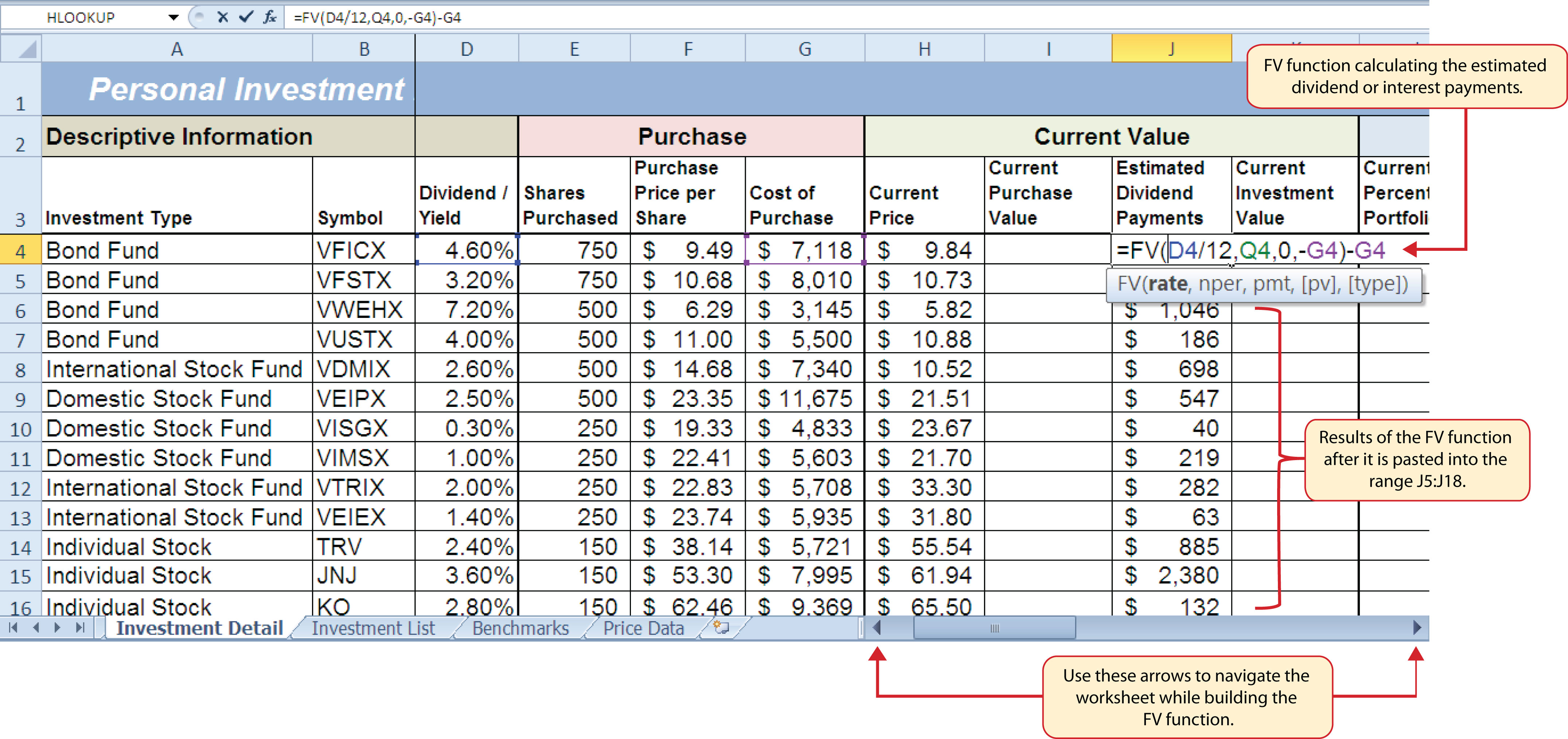The following steps explain how to add the formulas for the Current Purchase Value and Current Investment Value columns:

1. Click cell I4 on the Investment Detail worksheet.
2. Enter a formula that multiplies the Current Price in cell H4 by the Shares Purchased in cell E4.
3. Copy the formula in cell I4 and paste it into the range I5:I18 using the Paste Formulas command.
4. Click cell K4 on the Investment Detail worksheet.
5. Enter a formula that adds the Current Purchase Value in cell I4 to the Estimated Dividend Payments in cell J4.
6. Copy the formula in cell K4 and paste it into the range K5:K18 using the Paste Formulas command.
7. Click cell K19 on the Investment Detail worksheet.
8. Enter a SUM function that adds the values in the range K4:K18.

Figure 3.7 "Completed Current Value Section of the Investment Detail Worksheet" shows the completed columns of the Current Value section in the Investment Detail worksheet. The formula used to calculate the Current Investment Value illustrates why we used the FV function to calculate the estimated dividend or interest payments for an investment. Investments that earn interest or dividends can achieve growth in two ways. The first way is through interest or dividend payments. The second way is through changes in the price paid for the investment. The formula used to calculate the Current Purchase Value is taking the number of shares purchased for each investment and multiplying it by the current market price. Therefore, the Current Investment Value takes into account any changes in the investment price by adding the purchase value at the current market price to any dividends or interest payments earned.

Figure 3.7 Completed Current Value Section of the Investment Detail Worksheet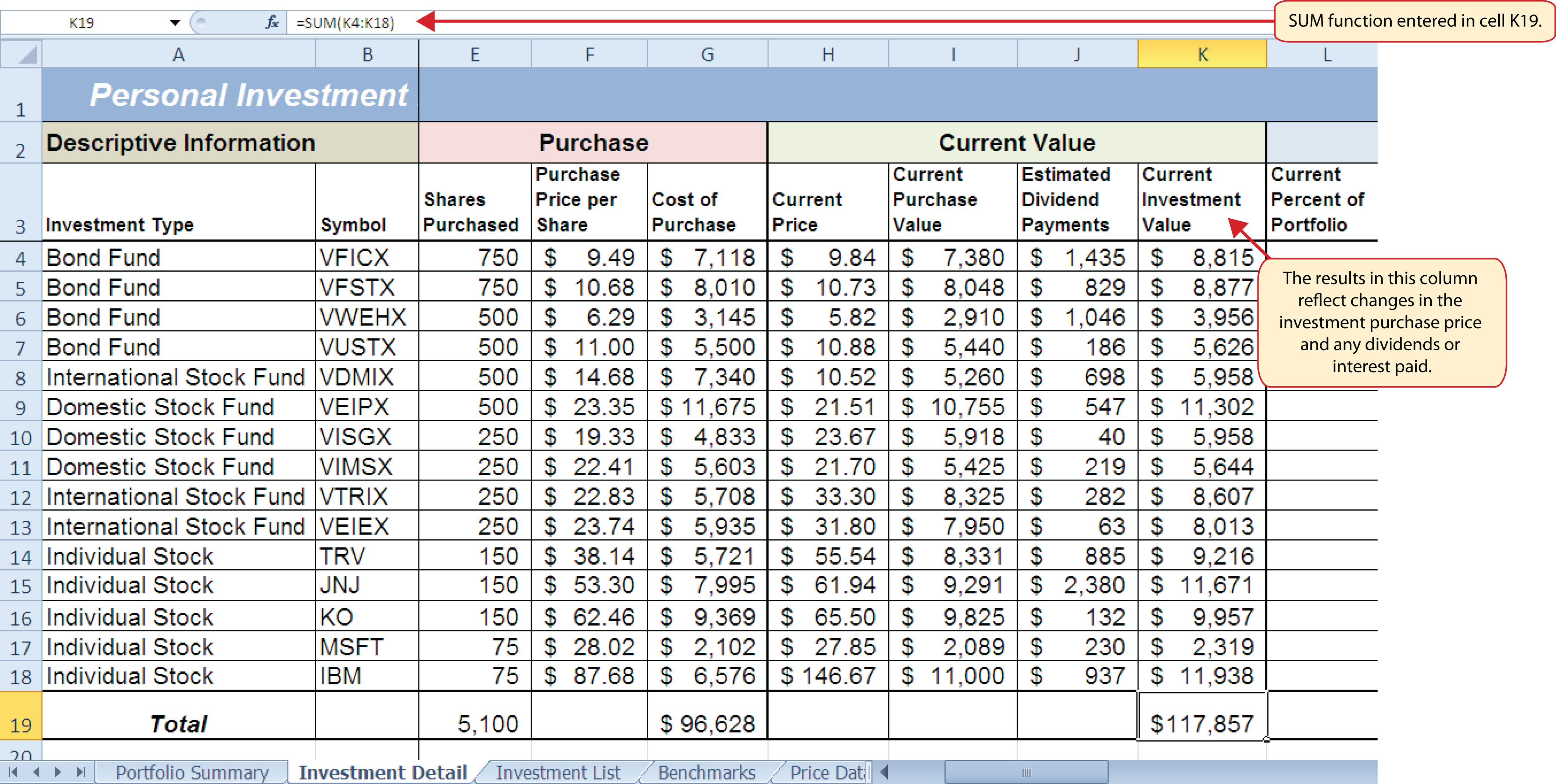Table 3.4 "Definitions for Columns L through R of the Investment Detail Worksheet" provides definitions for the Percent of Portfolio section of the Investment Detail worksheet (Columns L through R).

Table 3.4 Definitions for Columns L through R of the Investment Detail Worksheet

Category Definition
Current Percent of Portfolio The current investment value divided by the total current value of the investment portfolio.
Target Percent of Portfolio The planned percentage each investment is intended to have for the entire portfolio.
Current vs. Target The difference between the Current Percent of Portfolio column and the Target Percent of Portfolio column.
Rebalance Indicator Shows which investments do not match the target percentage of the portfolio. For example, as one investment increases in value due to an increase in market price, it will comprise a greater percentage of the portfolio. This may require that some shares of this asset be sold and invested in other areas that may have decreased in value. This is known as rebalancing the portfolio, and it helps you sell investments when prices are high and buy investments when prices are low.
Buy/Sell Indicator Based on the results of the Rebalance Indicator, a logical function is used to indicate whether an investment should be purchased or sold.
Months Owned Shows how many months an investment is owned. The length of ownership is expressed in terms of months since dividend payments on stock funds and interest payments on bond funds are distributed monthly.
Long/Short Indicator Shows whether an investment has been owned long enough to qualify as a long-term investment, which is greater than twelve months. The amount of taxes paid on the amount of money gained for a short-term investment is greater than a long-term investment. Therefore, there is a tax incentive to hold investments for more than twelve months.

The Percent of Portfolio section of the Investment Detail worksheet (Columns L through R) requires two formulas and one function. The following steps explain how we add them to the worksheet:

1. Click cell L4 in the Investment Detail worksheet.
2. Enter a formula that divides the Current Investment Value in cell K4 by the total in cell K19.
3. Place an absolute reference on cell K19 in the formula by placing the cursor in front of the column letter and pressing the F4 key on your keyboard.
4. Copy the formula and paste it into the range L5:L18 using the Paste Formulas command.
5. Click cell N4 in the Investment Detail worksheet.
6. Enter a formula that subtracts the Target Percent of Portfolio (cell M4) from the Current Percent of Portfolio (cell L4): L4−M4.
7. Copy the formula and paste it into the range N5:N18 using the Paste Formulas command.
8. Click cell Q19 in the Investment Detail worksheet.
9. Enter an AVERAGE function that calculates the average of the values in the range Q4:Q18.

Figure 3.8 "Percent of Portfolio Section of the Investment Detail Worksheet" shows the results of adding two formulas and a function to the Percent of Portfolio section of the Investment Detail worksheet. Notice the absolute reference added to the cell reference for K19 in the formula in the Current Percent of Portfolio column.

Figure 3.8 Percent of Portfolio Section of the Investment Detail Worksheet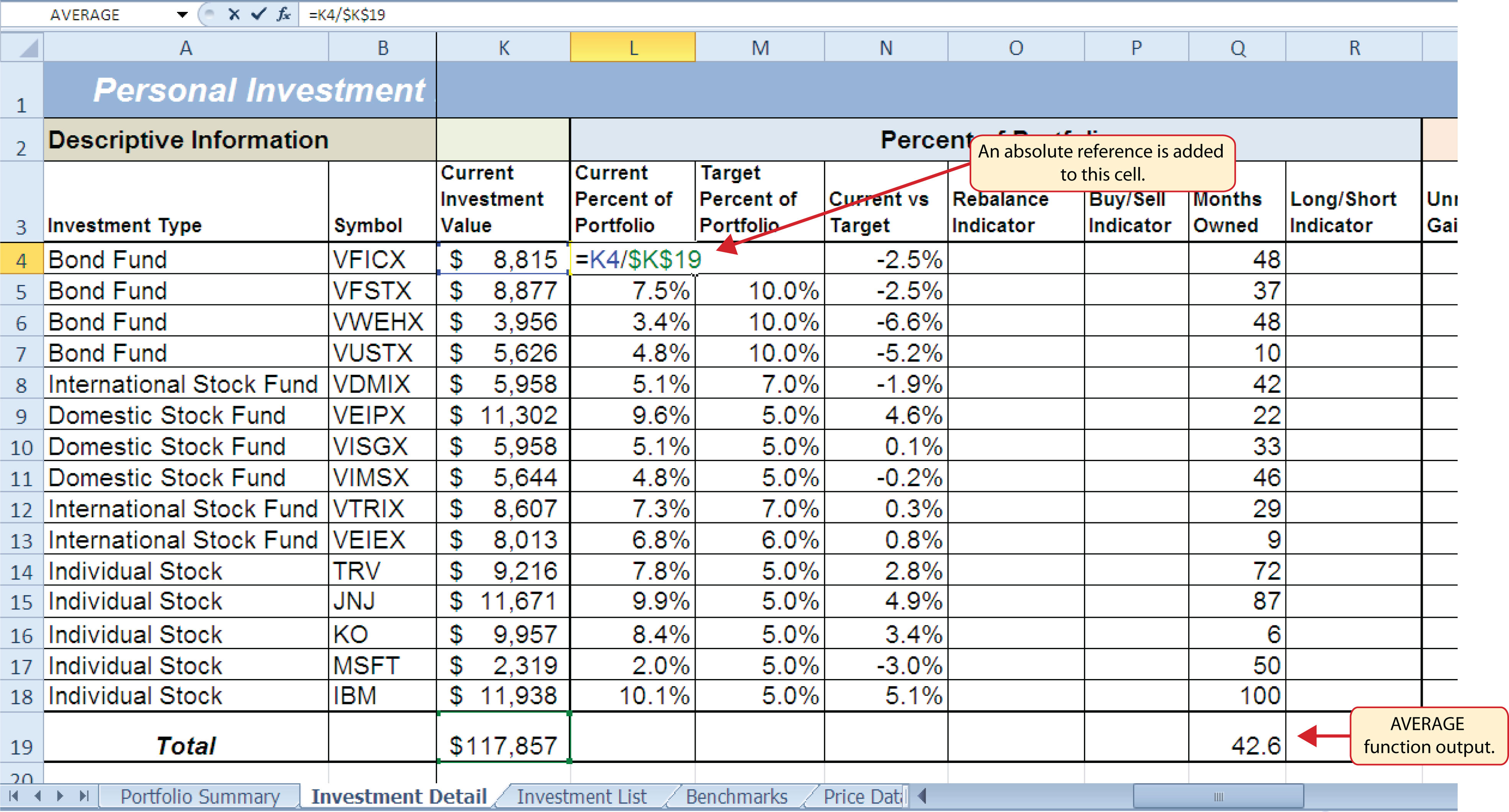Table 3.5 "Definitions for Columns S through X of the Investment Detail Worksheet" provides definitions for the columns in the Performance Analysis section of the Investment Detail worksheet.

Table 3.5 Definitions for Columns S through X of the Investment Detail Worksheet

Category Definition
Unrealized Gain/Loss The amount of money gained or lost on an investment. It is considered unrealized because the loss or gain does not actually occur until the investment is sold.
Percent Gain/Loss The percentage increase or decrease based on the unrealized gain/loss and the purchase value of an investment.
Target Annual Growth Rate The expected annual growth rate for an investment. All investments are expected to grow over time. The rate of growth depends on the amount of risk taken. Investments that are a higher risk are expected to pay a higher rate of return.
Actual Annual Growth Rate The percentage gain/loss divided by the amount of time an investment is owned expressed in terms of years.
Target vs. Actual Growth Rate The difference between the actual annual growth rate and the target annual growth rate.
Performance Indicator A logical function will be used to indicate which investments are underperforming with respect to the target vs. actual growth rate.

Most of the columns in the Performance Analysis section of the Investment Detail worksheet will be completed with formulas and functions. The following steps explain how we add them to the worksheet:

1. Click cell S4 on the Investment Detail worksheet.
2. Enter a formula that subtracts the value in the Cost of Purchase column (cell G4) from the value in the Current Investment Value column (cell K4): K4−G4.
3. Copy the formula and paste it into the range S5:S19 using the Paste Formulas command. Note that this formula will be used to calculate the output for the Total row in this column. The results of the formula are showing how much money has been earned or lost for each investment. It is important to note that these gains or losses do not actually happen unless the investment is sold.
4. Click cell T4 on the Investment Detail worksheet.
5. Enter a formula that divides the Unrealized Gain/Loss (cell S4) by the Cost of Purchase (cell G4): S4/G4.
6. Copy the formula in cell T4 and paste it into the range T5:T19 using the Paste Formulas command.
7. Click cell V4 on the Investment Detail worksheet.
8. Enter a formula that divides the Percent Gain/Loss (cell T4) by the result of dividing the Months Owned (cell Q4) by 12: T4/(Q4/12). Dividing the Months Owned value by 12 expresses the amount of time an investment has been owned in terms of years. The benchmark growth rates for most investments are expressed in terms of annual return rates. Therefore, this formula must first express the amount of time an investment has been owned in terms of years. Then the total percentage gain or loss for each investment is divided by the length of ownership in years to calculate the actual annual rate of return.
9. Copy the formula in cell V4 and paste it into the range V5:V19 using the Paste Formulas command.
10. Click cell W4 on the Investment Detail worksheet.
11. Enter a formula that subtracts the Target Annual Growth Rate (cell U4) from the Actual Annual Growth Rate (cell V4): V4−U4.
12. Copy the formula in cell W4 and paste it into the range W5:W18 using the Paste Formulas command.

Figure 3.9 "Performance Analysis Section of the Investment Detail Worksheet" shows the results of the formulas added to the Performance Analysis section of the Investment Detail worksheet. This completes the required formulas and functions necessary to add before moving on to the logical and lookup functions of the chapter.

Figure 3.9 Performance Analysis Section of the Investment Detail Worksheet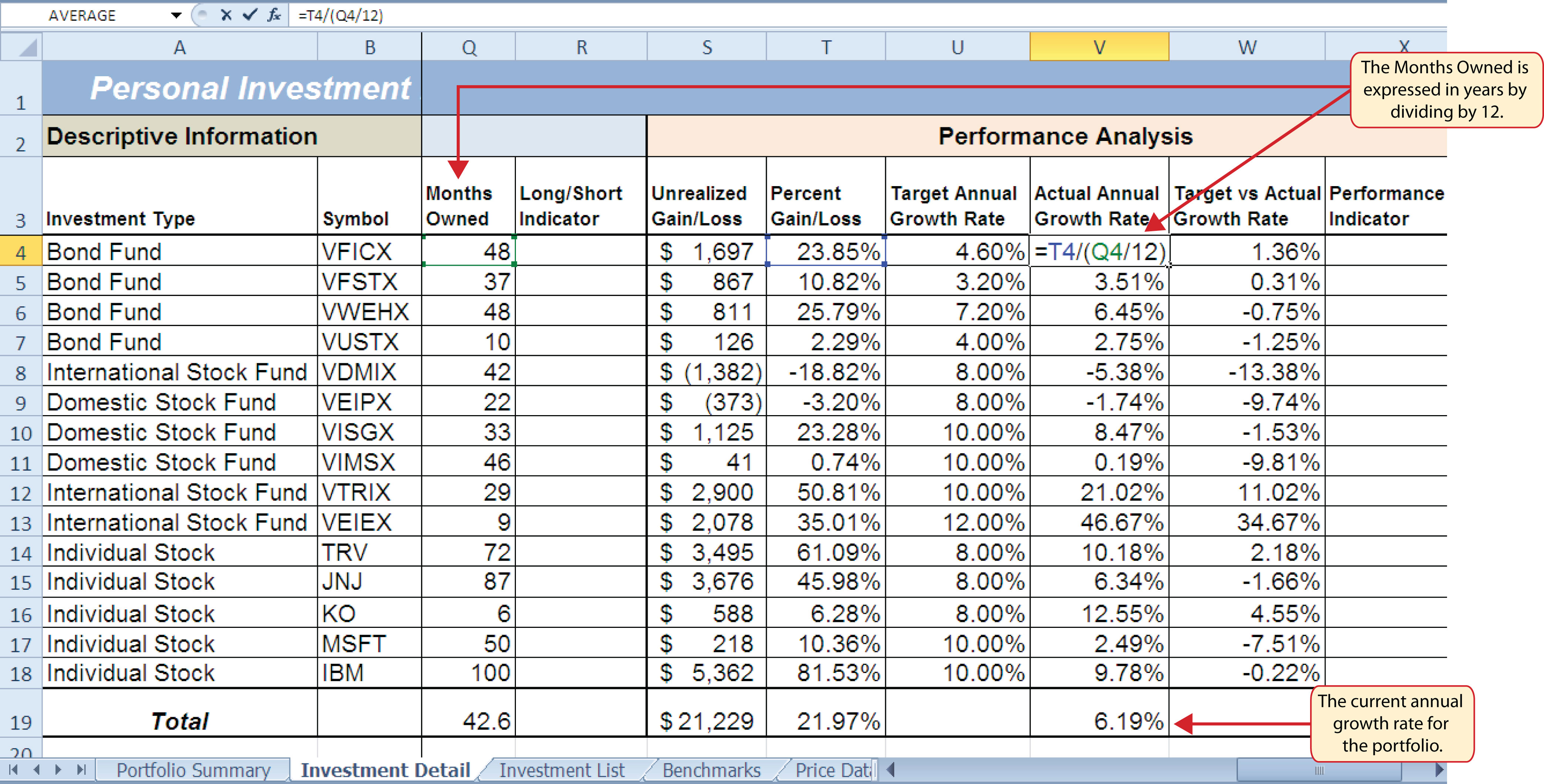## The Logical Test

Follow-along file: Continue with Excel Objective 3.00. (Use file Excel Objective 3.02 if starting here.)

### Lesson Video: The Logical Test

A key component for the logical functions that will be demonstrated in this section is the logical testAn expression used to evaluate the contents of a cell location. The logical test typically contains comparison operators such as equal to (=), greater than (>), less than (<), and so on. The results of the logical test can be either true or false. An example of a logical test is B8 >= 25, which is read as “if the value in cell B8 is greater than or equal to 25.”. A logical test is used in logical functions to evaluate the contents of a cell location. The results of the logical test can be either true or false. For example, the logical test C7 = 25 (read as “if the value in cell C7 is equal to 25”) can be either true or false depending on the value that is entered into cell C7. A logical test can be constructed with a variety of comparison operators, as shown in Table 3.6 "Comparison Operator Symbols and Definitions". These comparison operators will be used in the logical test arguments for the logical functions demonstrated in this chapter.

Table 3.6 Comparison Operator Symbols and Definitions

Symbol Definition
= Equal To
> Greater Than
> Less Than
< > Not Equal To
> = Greater Than or Equal To
< = Less Than or Equal To

A logical test will be used to evaluate the contents of a cell location in the Investment Detail worksheet. We will first demonstrate how the logical test is used to evaluate the contents of a cell location. Then we will use this logical test in the IF function, which will be demonstrated next. The following steps explain how the logical test is constructed:

1. Click cell R4 on the Investment Detail worksheet.
2. Type an equal sign (=).
3. Click cell Q4.
4. Type the greater than sign (>) followed by an equal sign (=).
5. Type the number 12. This completes the logical test, which is shown in Figure 3.10 "Logical Test Entered into the Investment Detail Worksheet". The logical test would be stated as: “If the value in cell Q4 is greater than or equal to 12.”

Figure 3.10 Logical Test Entered into the Investment Detail Worksheet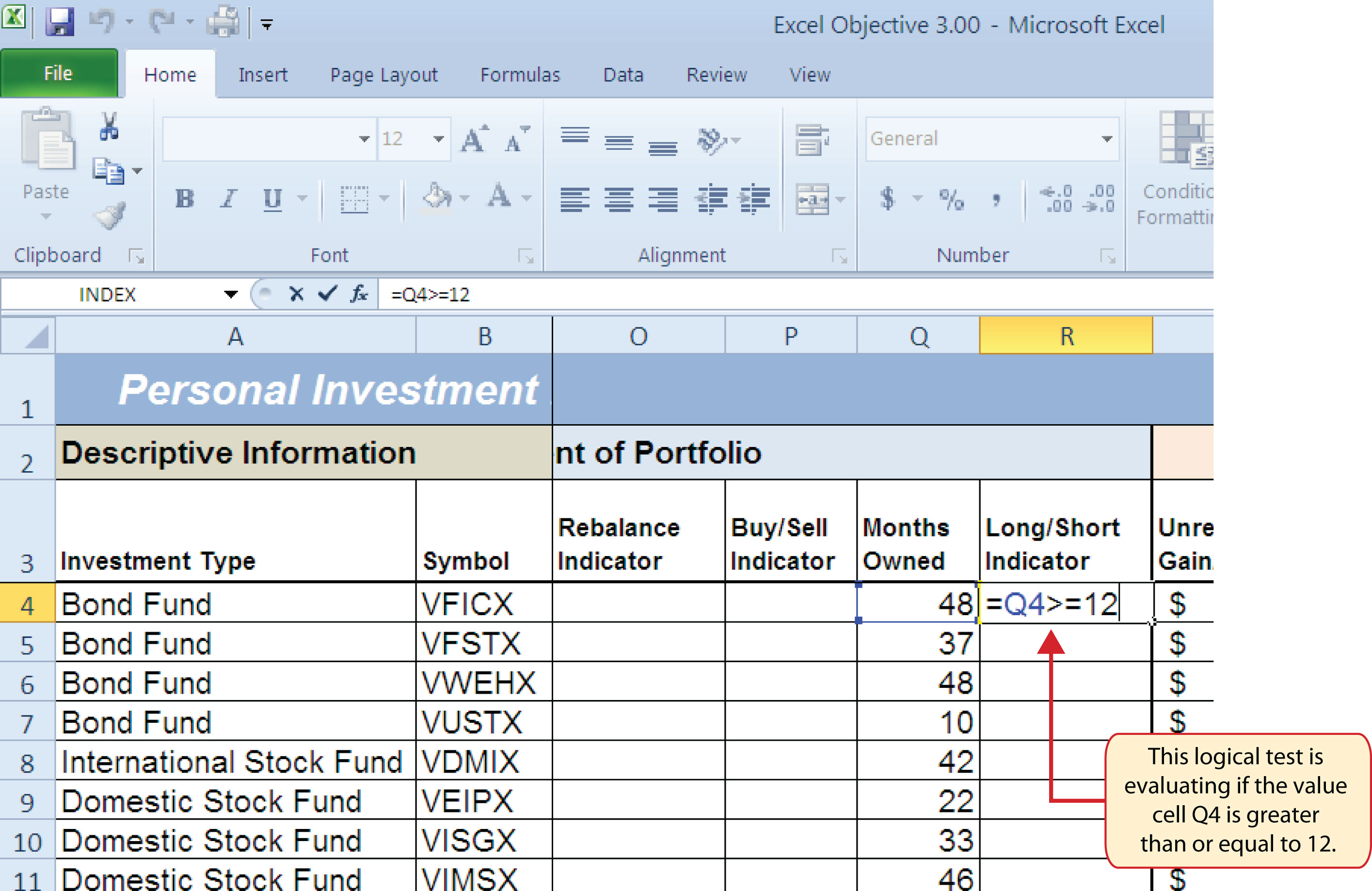6. Press the ENTER key on your keyboard. Notice that the output of the logical test is the word TRUE. This is because the value in cell Q4 is 48, which is greater than 12 (see Figure 3.11 "Output of the Logical Test").
7. Copy the logical test in cell R4 and paste it into the range R5:R18 using the Paste Formulas command.

Figure 3.11 "Output of the Logical Test" shows the results of the logical test after it is pasted into the range R5:R18. Notice that for any values that are less than 12 in the range Q4:Q18, the logical test produces an output of FALSE.

Figure 3.11 Output of the Logical Test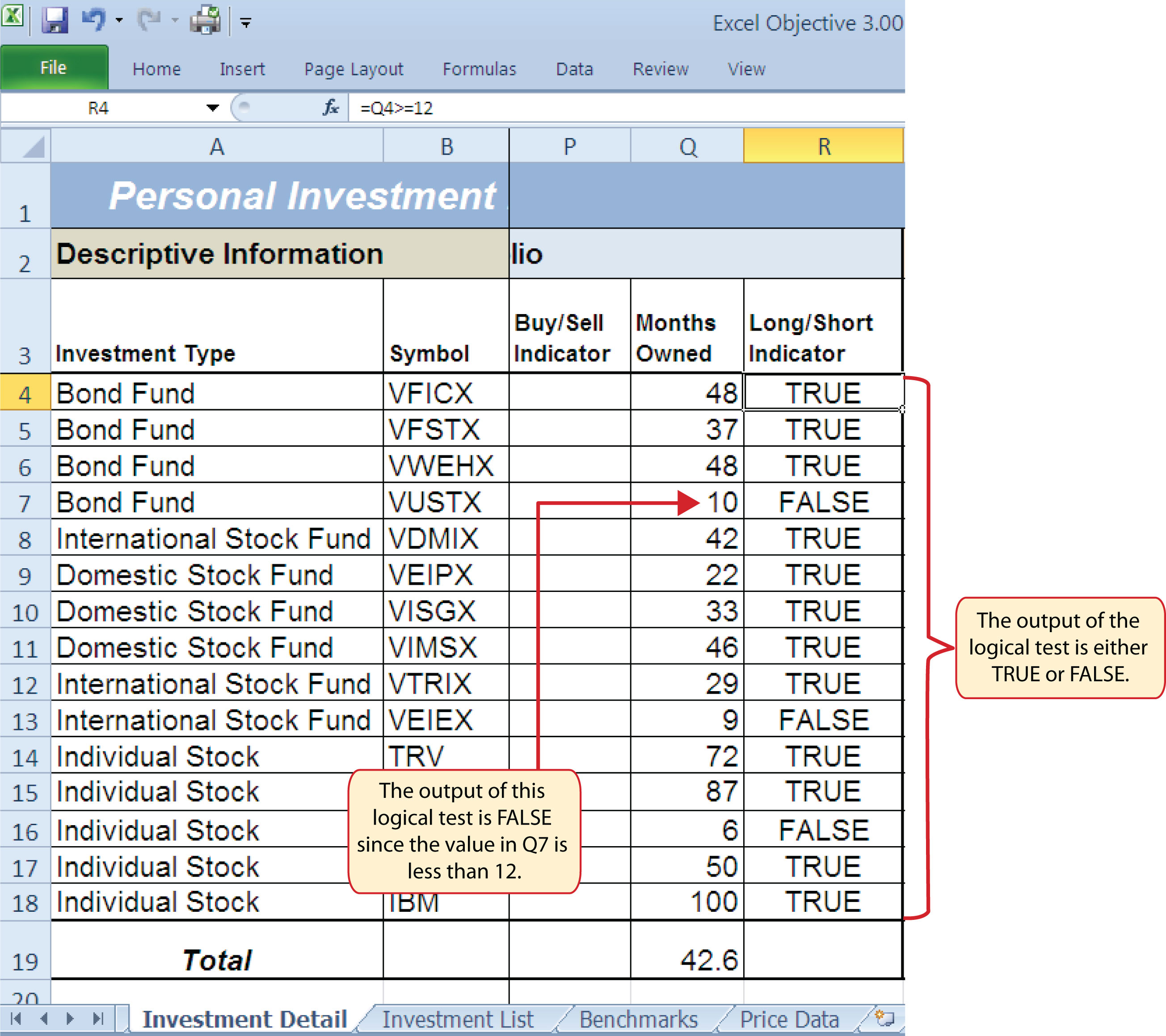## IF Function

Follow-along file: Continue with Excel Objective 3.00. (Use file Excel Objective 3.03 if starting here.)

### Lesson Video: IF Function

The IF function is used to produce a custom output based on the results of a logical test. If the results of the logical test are TRUE, the IF function can display a specific number or text, or perform a calculation. If the results of the logical test are FALSE, the IF function can display a different number or text, or perform a different calculation. The arguments of the IF function are defined in Table 3.7 "Arguments for the IF Function".

Table 3.7 Arguments for the IF Function

Argument Definition
Logical_test A test used to evaluate the contents of a cell location. This argument typically utilizes comparison operators, which are defined in Table 3.6 "Comparison Operator Symbols and Definitions". The results of the test can be either true or false. For example, the test C7>25 would be read as if C7 is greater than 25. If the number 30 is entered into cell C7, the logical test is true. If you are evaluating a cell that contains text data, the text in the logical test must be placed inside quotation marks. For example, if you wanted to test if the word Long is in cell C7, the logical test would be C7 = “Long”.
[Value_if_true] The output that will be displayed by the function or the calculation that will be performed by the function if the results of the logical test are true. This argument can be defined with a formula, function, number, or text. However, when defining this argument with a text output such as the word Long, it must be placed inside quotation marks (“Long”).
[Value_if_false] The output that will be displayed by the function or the calculation that will be performed by the function if the results of the logical test are false. This argument can be defined with a formula, function, number, or text. However, when defining this argument with a text output such as the word Long, it must be placed inside quotation marks (“Long”).

We will use the IF function in the Percent of Portfolio section of the Investment Detail worksheet. We will use the logical test that was previously demonstrated within the IF function to determine if an investment has been held for a short or long period of time. For tax purposes, an investment is considered short-term if it is held less than twelve months. This requires the investor to pay a higher tax percentage for any profit earned on the investment. An investment held twelve months or longer is considered a long-term investment. The following explains how the IF function is used to identify which investments are long term or short term:

1. Highlight the range R4:R18 on the Investment Detail worksheet and press the DELETE key on your keyboard. This will remove the logical test and allow us to replace it with an IF function.
2. Click cell R4 on the Investment Detail worksheet.
3. Click the Formulas tab on the Ribbon.
4. Click the Logical button in the Function Library group of commands.
5. Click the IF function from the list of functions (see Figure 3.12 "Selecting the IF Function from the Function Library"). This opens the Function Arguments dialog box.

Figure 3.12 Selecting the IF Function from the Function Library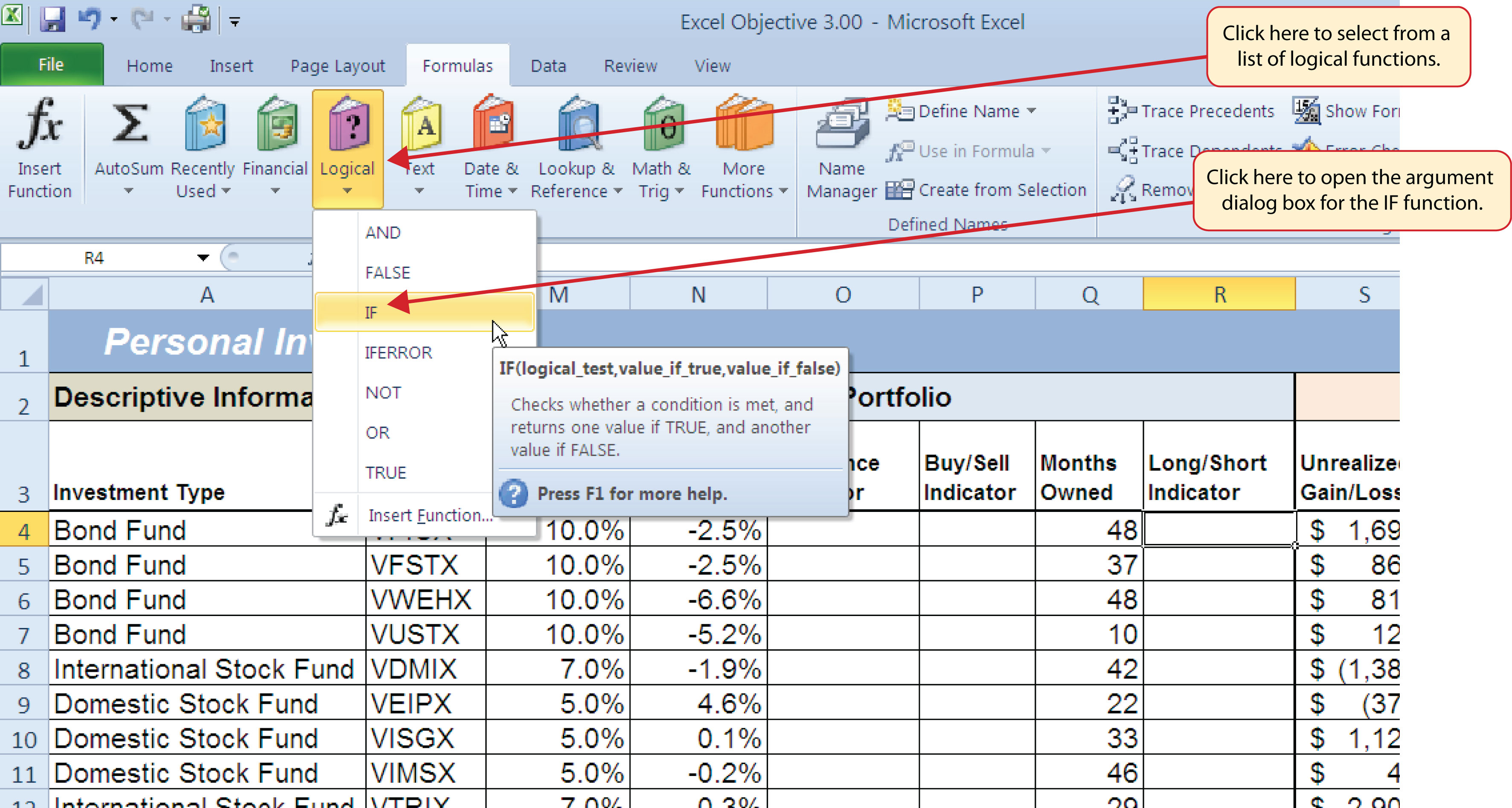6. Click the Collapse Dialog button next to the Logical_test argument (see Figure 3.13 "Logical_Test Argument Defined").
7. Click cell Q4 and press the ENTER key on your keyboard.
8. Type the greater than sign (>) followed by an equal sign (=).
9. Type the number 12.

Figure 3.13 "Logical_Test Argument Defined" shows the appearance of the IF Function Arguments dialog box after defining the Logical_test argument. Notice that next to the Logical_test input box, Excel shows that the results of the test are true. This makes sense given that the value in cell Q4 is 48, which is greater than 12.

Figure 3.13 Logical_Test Argument Defined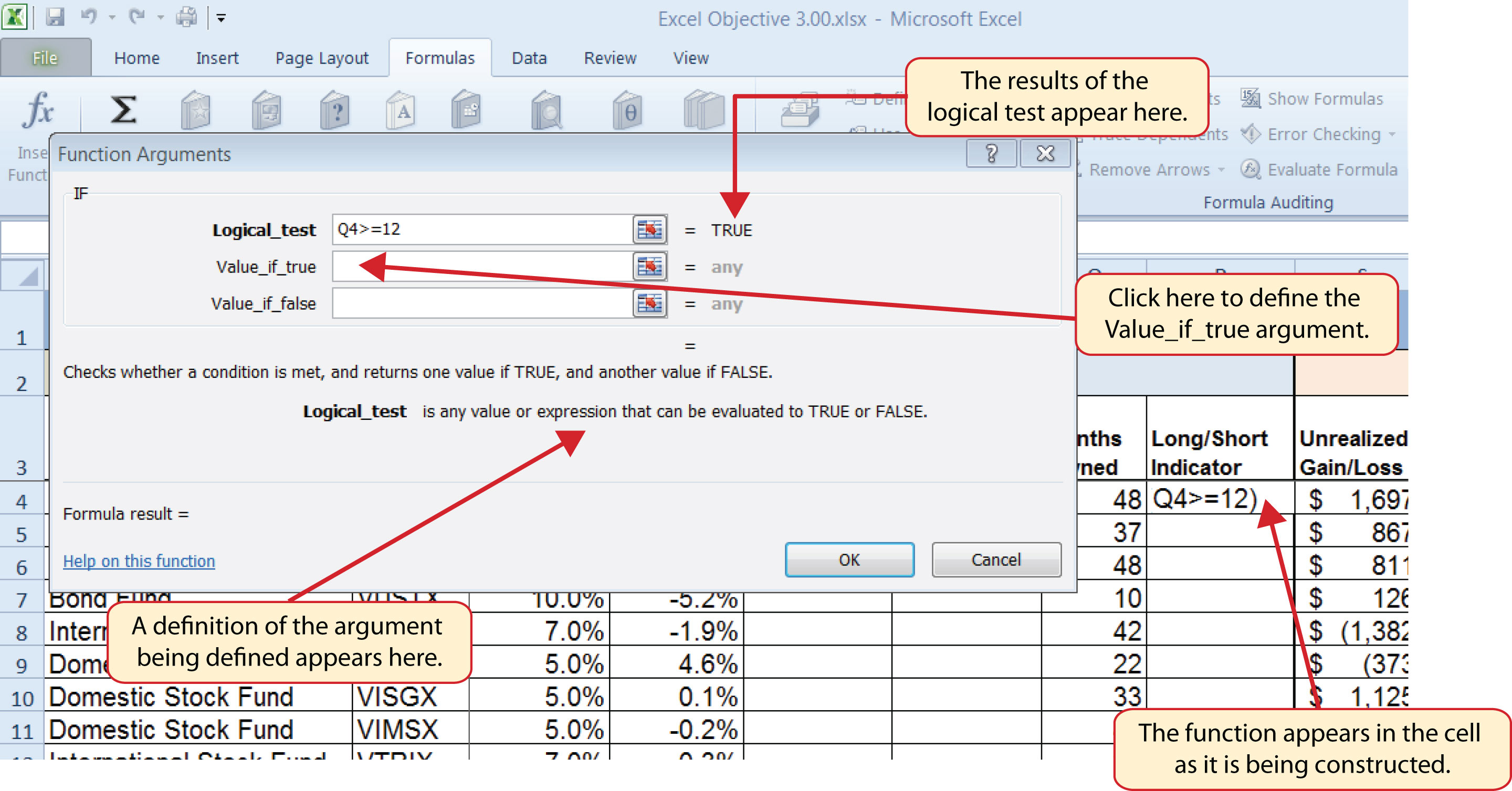10. Press the TAB key on your keyboard to advance to the next argument, which is Value_if_true.
11. Type the word Long in quotation marks. If you forget to put words or text in quotation marks using the Function Arguments dialog box, Excel will insert the quotation marks for you.
12. Press the TAB key on your keyboard to advance to the next argument, which is Value_if_false.
13. Type the word Short in quotation marks.
14. Click the OK button on the Function Arguments dialog box to complete the function.
15. Copy the IF function in cell R4 and paste it into the range R5:R18 using the Paste Formulas command.

### Integrity Check

Placing Text in Quotation Marks for Logical Functions

If you are using a logical function to evaluate text data in a cell location, or if you are using a logical function to output text data, the text must be placed inside quotation marks. For example, if you are using a logical function to evaluate whether the word Long is entered into cell B5, the logical test must appear as follows: B5= “Long”. If you omit the quotation marks, the function may produce an erroneous false result for the test.

Figure 3.14 "Completed Function Arguments Dialog Box for the IF Function" shows the completed Function Arguments dialog box for the IF function. Notice that the results of the function are displayed in the dialog box. Since the value in cell Q4 is greater than 12, the word Long will be displayed in cell R4.

Figure 3.14 Completed Function Arguments Dialog Box for the IF Function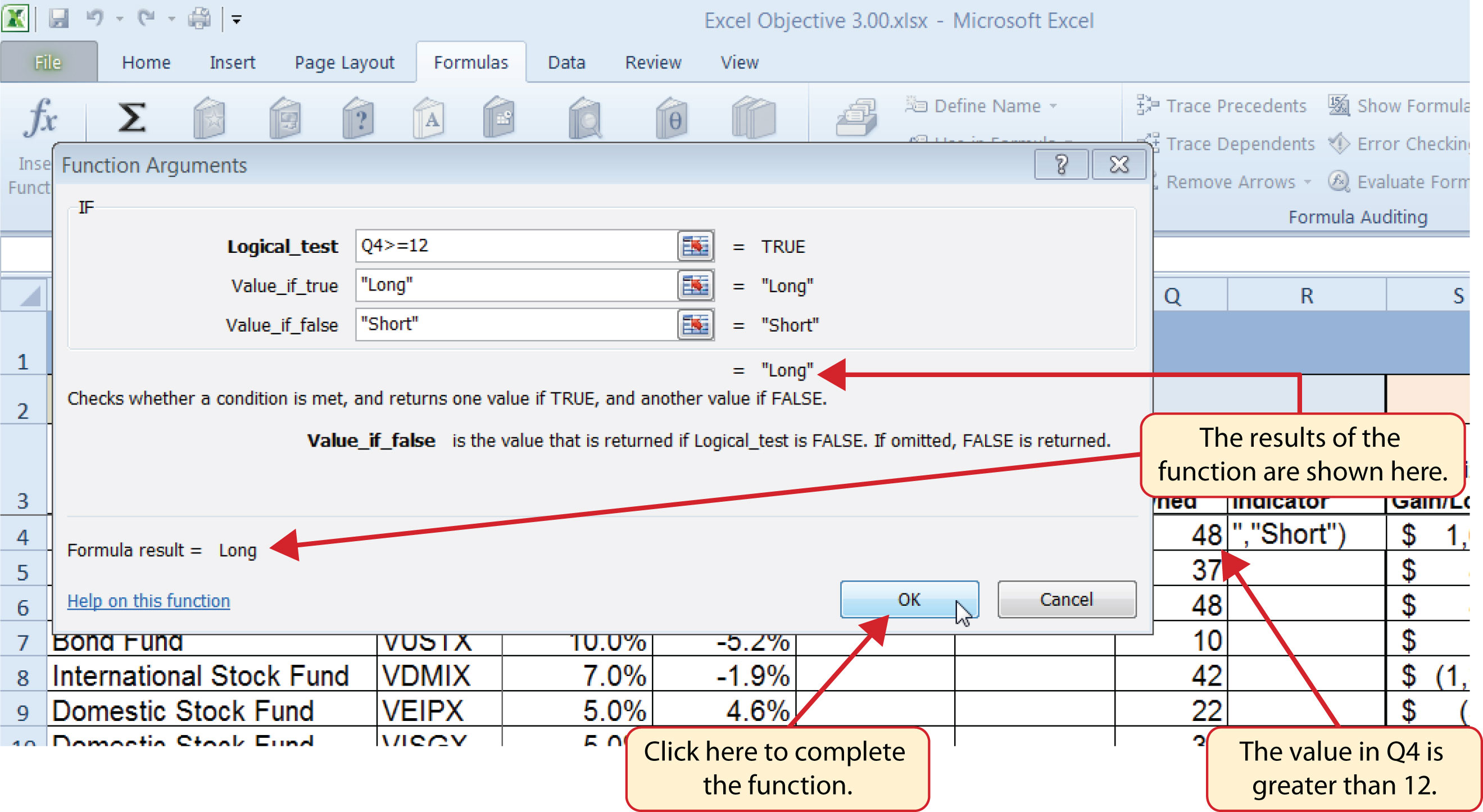Figure 3.15 "IF Function Output" shows the completed Long/Short Indicator column on the Investment Detail worksheet. Notice the word Short is displayed for any investment held less than twelve months.

Figure 3.15 IF Function Output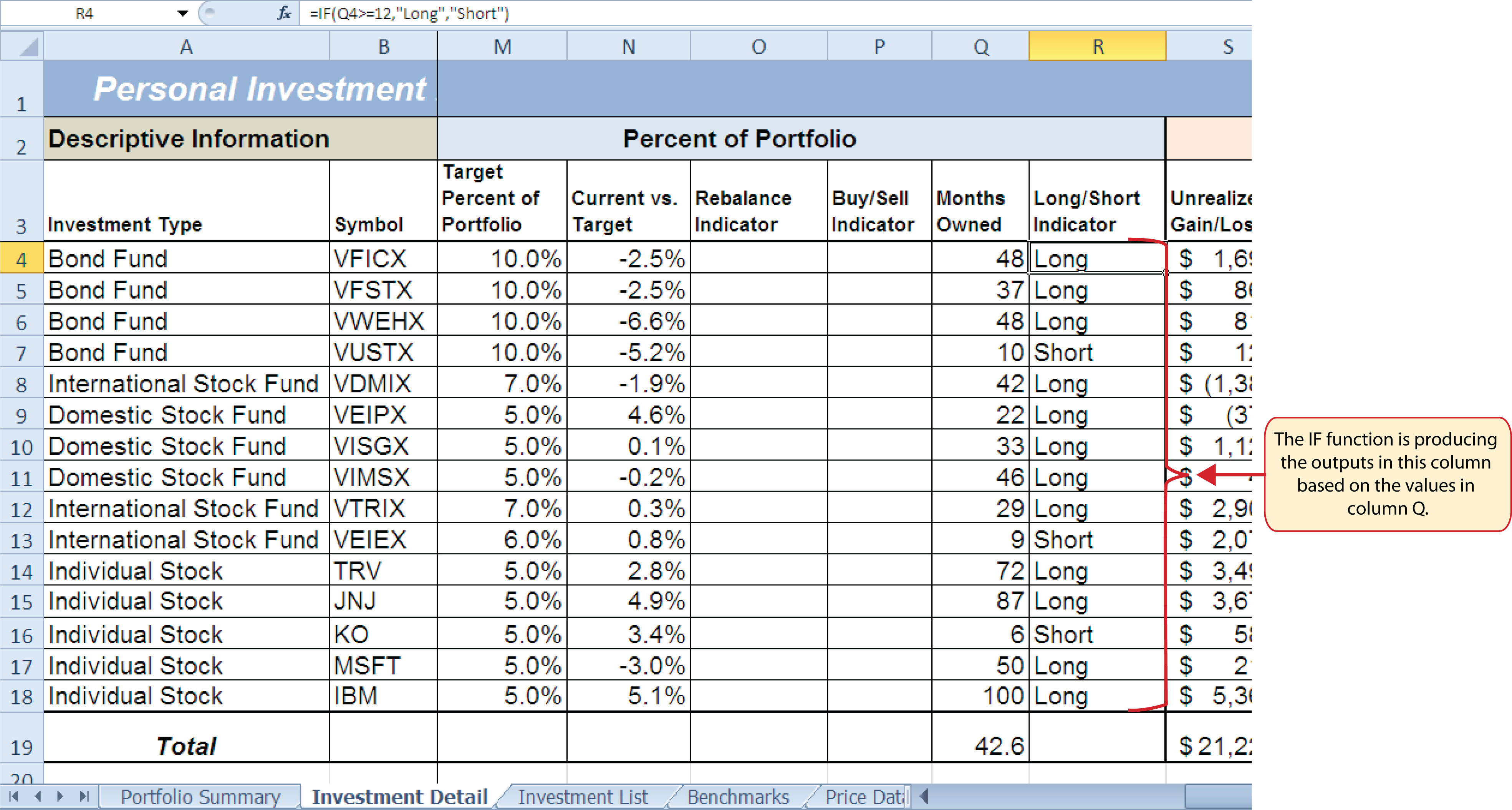### Skill Refresher: IF and Nested IF Function

1. Type an equal sign (=).
2. Type the function name IF followed by an open parenthesis (().
3. Define the logical_test argument to evaluate the contents of a cell location such that the result of the test is either true or false.
4. Define the value_if_true argument, which will be the output of the function if the results of the logical test are true.
5. Define the value_if_false argument, which will be the output of the function if the results of the logical test are false. This argument can also be defined by starting another IF function if you are nesting IF functions.
6. Type a closing parenthesis ()). In the case of nested IF functions, type a closing parenthesis for every IF function that was started.
7. Press the ENTER key on your keyboard.

## The OR Function

Follow-along file: Continue with Excel Objective 3.00. (Use file Excel Objective 3.04 if starting here.)

### Lesson Video: OR Function

The OR function is similar to the IF function in that it uses a logical test to evaluate the contents of a cell location. However, the OR function allows you to define several logical tests as opposed to just one. If one of the logical tests is true, the output of the function will be the word TRUE. If all the logical tests are false, the output of the function will be the word FALSE. This differs from the IF function because the output of the function is only the word TRUE or the word FALSE. As a result, the OR function is commonly used within the IF function to enable specific outputs to be defined.

We will use the OR function in the Performance Indicator column on the Investment Detail worksheet. The purpose of this column is to identify any investment where either the Unrealized Gain/Loss is less than zero or the Target vs. Actual Growth Rate is less than –1%. We will use the function in the logical test of an IF function so we can define a specific output based on the results of the OR function. However, we will first demonstrate how the OR function works by itself, which is outlined in the following steps:

1. Click cell X4 on the Investment Detail worksheet.
2. Type an equal sign (=).
3. Type the function name OR followed by an open parenthesis (().
4. Click cell S4 on the Investment Detail worksheet.
5. Type the less than symbol (<) followed by a zero. This completes the first logical test, which is evaluating if the value in cell S4 is less than zero.
6. Type a comma. This advances the function to a second logical test.
7. Click cell W4 on the Investment Detail worksheet.
8. Type the less than symbol (<) followed by −1%. Be sure to include the minus sign and percent symbol. This completes the second logical test, which is evaluating if the value in cell W4 is less than –1%.
9. Type a closing parenthesis ()) and press the ENTER key on your keyboard.
10. Copy the OR function in cell X4 and paste it into the range X5:X18 using the Paste Formulas command.

Figure 3.16 Completed OR Function by Itself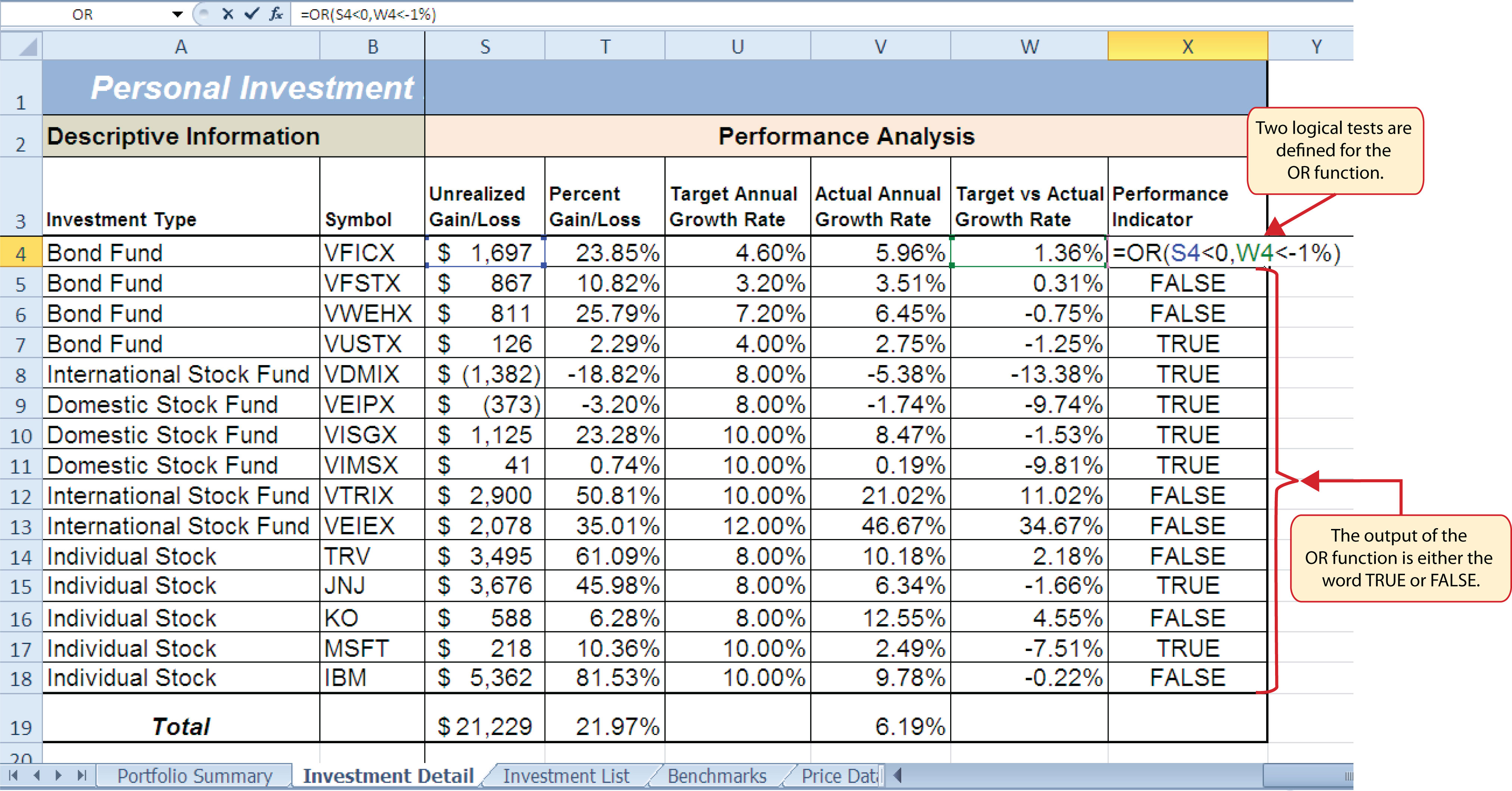Figure 3.16 "Completed OR Function by Itself" shows the construction and result of the OR function by itself. Notice that the only output of the function is the word TRUE or the word FALSE. If either the Unrealized Gain/Loss is less than zero or the Target vs. Actual Growth Rate is less than −1%, the function shows the word TRUE. However, these descriptions will not be helpful for the person using this worksheet. Displaying the words OK or Warning would be far more helpful in identifying investments that need to be evaluated. We can do this if we use the OR function in the logical test argument of the IF function. The following steps explain how to accomplish this:

1. Highlight the range X4:X18 on the Investment Detail worksheet and press the DELETE key on your keyboard. We are going to start over by creating an IF function.
2. Click cell X4 on the Investment Detail worksheet.
3. Type an equal sign (=).
4. Type the function name IF followed by an open parenthesis (().
5. Type the function name OR followed by an open parenthesis ((). The OR function is being placed into the logical_test argument of this IF function.
6. Click cell S4 on the Investment Detail worksheet.
7. Type the less than symbol (<) followed by a zero.
8. Type a comma. This advances the function to a second logical test.
9. Click cell W4 on the Investment Detail worksheet.
10. Type the less than symbol (<) followed by −1%.
11. Type a closing parenthesis ()).
12. Type an equal sign (=).
13. Type the word TRUE. Do not put the word inside quotation marks.
14. Type a comma. This completes the logical_test argument of the IF function. We can now go on to define the value_if_true and the value_if_false arguments. This will allow us to specify what the output of the function should be instead, using the OR function outputs of either TRUE or FALSE.
15. Type the word Warning. Be sure to enclose the word in quotation marks.
16. Type a comma. This will advance the function to the value_if_false argument.
17. Type the word OK. Be sure to enclose the word in quotation marks.
18. Type a closing parenthesis ())and press the ENTER key on your keyboard.
19. Copy the IF function in cell X4 and paste it into the range X5:X18 using the Paste Formulas command.

Figure 3.17 "OR Function in the Logical Test of the IF Function" shows the OR function within the logical_test argument of the IF function. The logical test of the IF function is now evaluating if the results of the OR function are true.

Figure 3.17 OR Function in the Logical Test of the IF Function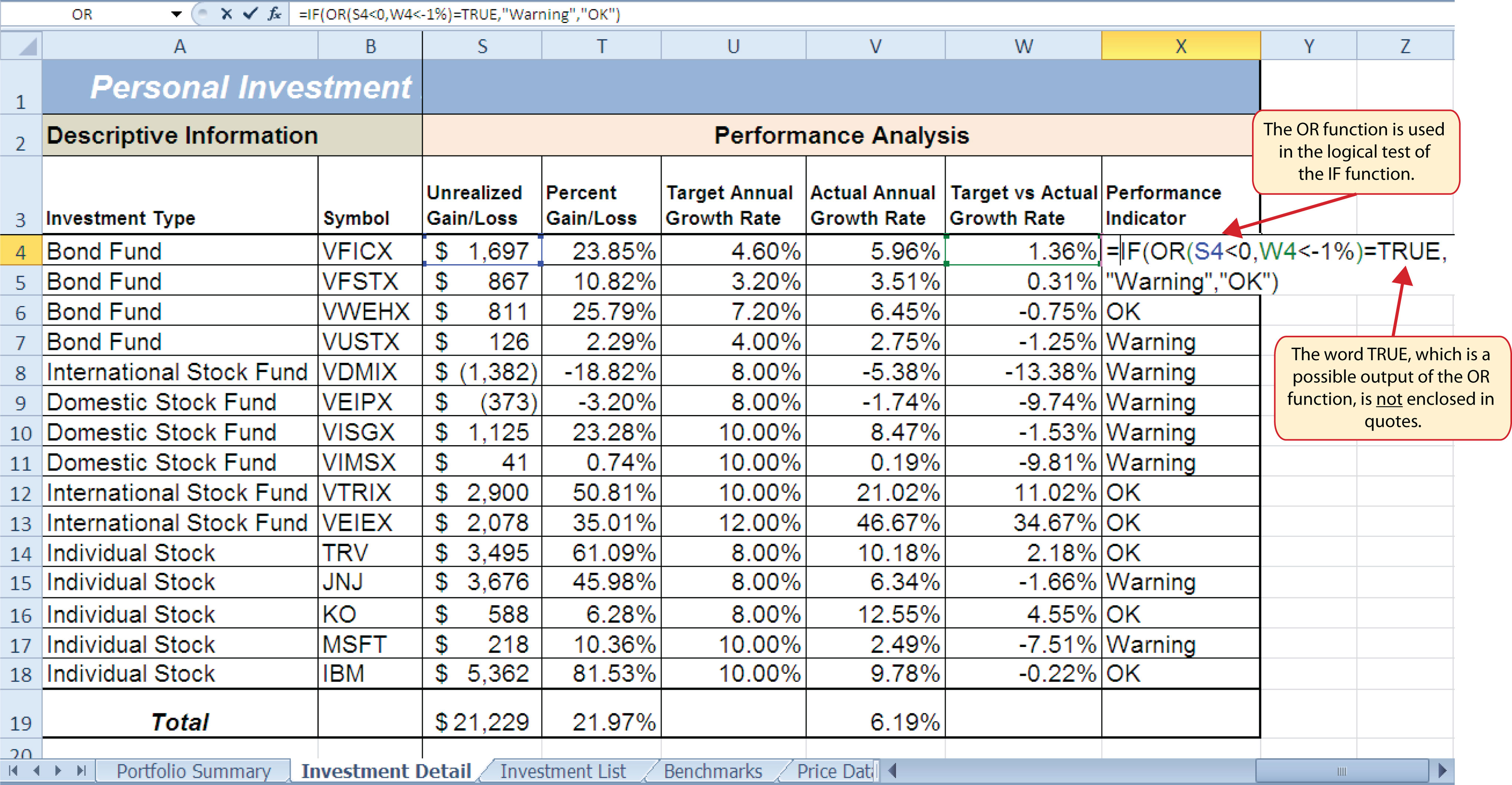### Skill Refresher: OR Function

1. Type an equal sign (=).
2. Type the function name OR followed by an open parenthesis (().
3. Define the logical_test argument to evaluate the contents of a cell location such that the result of the test is either true or false.
4. Define additional logical test arguments as needed. The output of the function will be TRUE if any of the logical tests are true.
5. Type a closing parenthesis ()).
6. Press the ENTER key on your keyboard.

## The AND Function

Follow-along file: Continue with Excel Objective 3.00. (Use file Excel Objective 3.05 if starting here.)

### Lesson Video: AND Function

The AND function is almost identical to the OR function in that it is composed of only logical tests and produces one of two possible outputs: TRUE or FALSE. However, all logical tests defined for the AND function must be true in order to produce a TRUE output. If one logical test is false, the function will produce a FALSE output. We will use the AND function to complete the Buy/Sell Indicator column on the Investment Detail worksheet. This column will show either the word Buy or the words Hold or Sell based on the results of the logical test argument of an IF function. We will use the AND function to define the logical test argument of the IF function. The following steps explain how to accomplish this:

1. Click cell P4 on the Investment Detail worksheet.
2. Type an equal sign (=).
3. Type the function name IF followed by an open parenthesis (().
4. Type the function name AND followed by an open parenthesis ((). The AND function is being placed into the logical_test argument of this IF function.
5. Click cell N4 and then type the less than symbol (<</b>).
6. Type a minus sign () followed by the number 1 and a percent symbol: (−1%).
7. Type a comma. This advances the AND function to the second logical test.
8. Click cell S4.
9. Type a greater than symbol (>) followed by an equal sign (=). These symbols are used to evaluate if the value in a cell location is greater than or equal to a target value.
10. Type a zero followed by a closing parenthesis ()).
11. Type an equal sign (=) followed by the word TRUE. Do not enclose the word in quotation marks.

Figure 3.18 "AND Function Placed in the Logical Test of an IF Function" shows the appearance of the AND function that has been added to the logical test of the IF function. The AND function will produce a true output if the value in cell N4 is <−1% and the value in cell S4 is greater than or equal to 0.

Figure 3.18 AND Function Placed in the Logical Test of an IF Function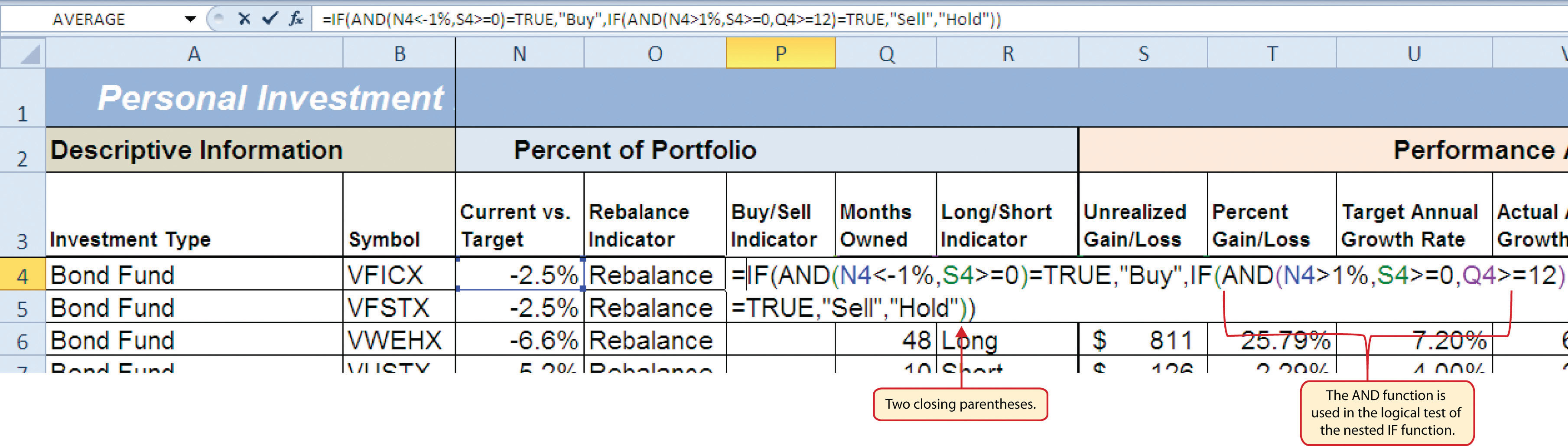12. Type a comma. This advances the IF function to the value_if_true argument.
13. Type the word Buy enclosed in quotation marks as shown in Figure 3.19 "Results of the AND Function in the Logical Test Argument of an IF Function". If the Current vs. Target value is less than −1% and the Unrealized Gain/Loss is greater than or equal to zero, the function will show the word Buy. In other words, if the investment is less than the desired percentage for the total portfolio and it is currently not losing money, we will buy more of that investment so it is in line with the target percentage of the portfolio.
14. Type a comma.
15. Type the words Hold or Sell enclosed in quotation marks as shown in Figure 3.19 "Results of the AND Function in the Logical Test Argument of an IF Function". For all other investments that are not designated with a Buy indicator, the function will show the words Hold or Sell. This indicates that an investment could either be held or sold.
16. Type a closing parenthesis ()) and press the ENTER key on your keyboard.
17. Copy the IF function in cell P4 and paste it into the range P5:P18 using the Paste Formulas command.
18. Increase the width of Column P to 12 points.

Figure 3.19 "Results of the AND Function in the Logical Test Argument of an IF Function" shows the results of the completed AND function within an IF function after it is copied and pasted into the range P5:P18.

Figure 3.19 Results of the AND Function in the Logical Test Argument of an IF Function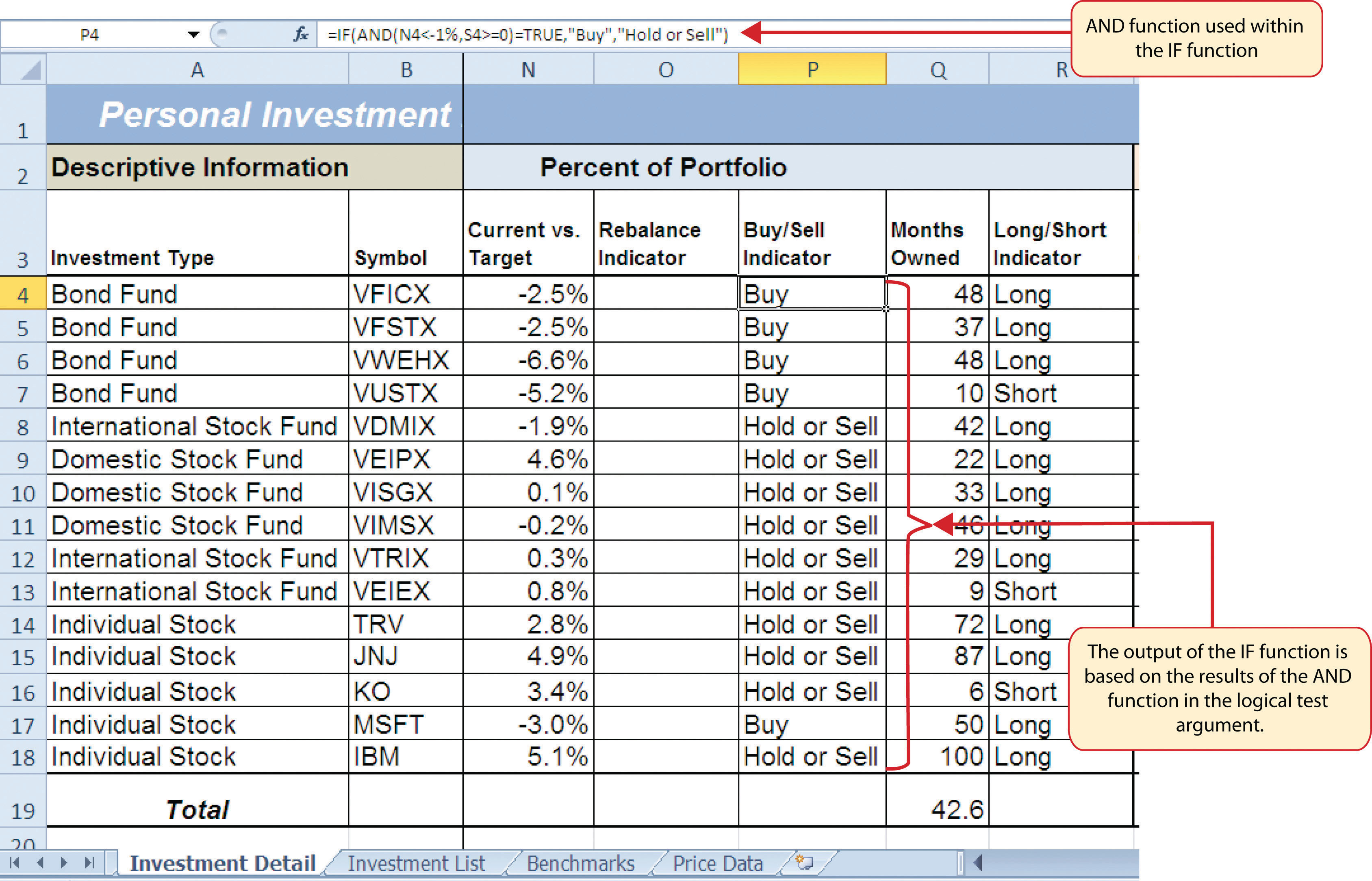### Skill Refresher: AND Function

1. Type an equal sign (=).
2. Type the function name AND followed by an open parenthesis (().
3. Define the logical_test argument to evaluate the contents of a cell location such that the result of the test is either true or false.
4. Define additional logical test arguments as needed. The output of the function will be TRUE if ALL of the logical tests are true.
5. Type a closing parenthesis ()).
6. Press the ENTER key on your keyboard.

## Nested IF Functions

Follow-along file: Continue with Excel Objective 3.00. (Use file Excel Objective 3.06 if starting here.)

### Lesson Video: Nested IF Functions

When constructing the IF function, the logical test can produce only two potential outcomes when evaluating the data in a cell. In addition, the function can produce only two possible outputs, which are defined in the value_if_true and value_if_false arguments. However, there may be situations when you need to test for several possible outcomes, which may require more than two possible outputs. To accomplish this, you need to create a nested IF functionUsed when more than two tests and two outputs are required when using the IF function. A nested IF function is when the value_if_true or value_if_false arguments of an IF function are defined with another IF function.. A nested IF function is when either the value_if_true or value_if_false arguments are defined with another IF function.

For the Personal Investment workbook, a nested IF function is required to complete the Rebalance Indicator column (Column O) on the Investment Detail worksheet (see Figure 3.19 "Results of the AND Function in the Logical Test Argument of an IF Function"). The purpose of this column is to indicate where the portfolio needs to be rebalanced. Looking at the Current vs. Target column (Column N) shown in Figure 3.19 "Results of the AND Function in the Logical Test Argument of an IF Function", you can see that several investments have a significant negative number where the investment value has fallen below the target percentage for the portfolio. Other investments have a significant positive number where the investment has exceeded the target percentage for the portfolio. For this portfolio, a number greater than 1% or less than –1% will be considered significant. Therefore, we will need to assess three possible outcomes when creating a logical test that evaluates the values in Column N. The first test will be if the value is greater than 1%. The second test will be if the value is less than –1%. The third test will be if both the first test and the second test are false. This is why we need to construct a nested IF function to produce the outputs in the Rebalance Indicator column. The following steps explain how to accomplish this:

1. Click cell O4 on the Investment Detail worksheet.
2. Type an equal sign (=).
3. Type the function name IF followed by an open parenthesis (().
4. Click cell N4.
5. Type the greater than symbol (>) followed by the number 1%. It is important to use the percent symbol (%) after the number 1. If you omit the percent symbol, Excel will test if the value in cell N4 is greater than 100%.
6. Type a comma.
7. Type the word Rebalance inside quotation marks. When using text data to define any of the arguments for the IF function, the text must be placed inside quotation marks.
8. Type a comma.
9. Start another IF function by typing the function name IF followed by an open parenthesis (().
10. Click cell N4.
11. Type the less than symbol (<) followed by −1%.
12. Type a comma.
13. Type the word Rebalance inside quotation marks.
14. Type a comma.
15. Type the word OK inside quotation marks.
16. Type two closing parentheses ())). Since two IF functions were started, there are two open parentheses in the function. As a result, we need to add two closing parentheses; otherwise, Excel will produce an error message stating that a closing parenthesis is missing.
17. Press the ENTER key on your keyboard.
18. Copy the nested IF function in cell O4 and paste it into the range O5:O18 using the Paste Formulas command.

### Integrity Check

Using Logical Functions to Evaluate Percentages

If you are using a logical function to evaluate percentages in a cell location, be sure to use the percent symbol when defining the logical test. For example, if you are testing cell location B5 to determine if the value is greater than 10%, the logical test should appear as follows: B5>10%. If you omit the percent sign, the logical test will evaluate cell B5 to see if the value is greater than 1000%. This may erroneously force the function to produce the value_if_false output. You can also convert the percentage to a decimal in the logical test. For example, in decimal form, the logical test can be constructed as follows: B5>.10.

Figure 3.20 "Completed Nested IF Function" shows how the completed nested IF function should appear in cell O4 of the Investment Detail worksheet. In addition, we see the results of the function after it was pasted into the range O5:O18. Notice that for any investment where the Current vs. Target value is between plus or minus 1%, the word OK appears.

Figure 3.20 Completed Nested IF Function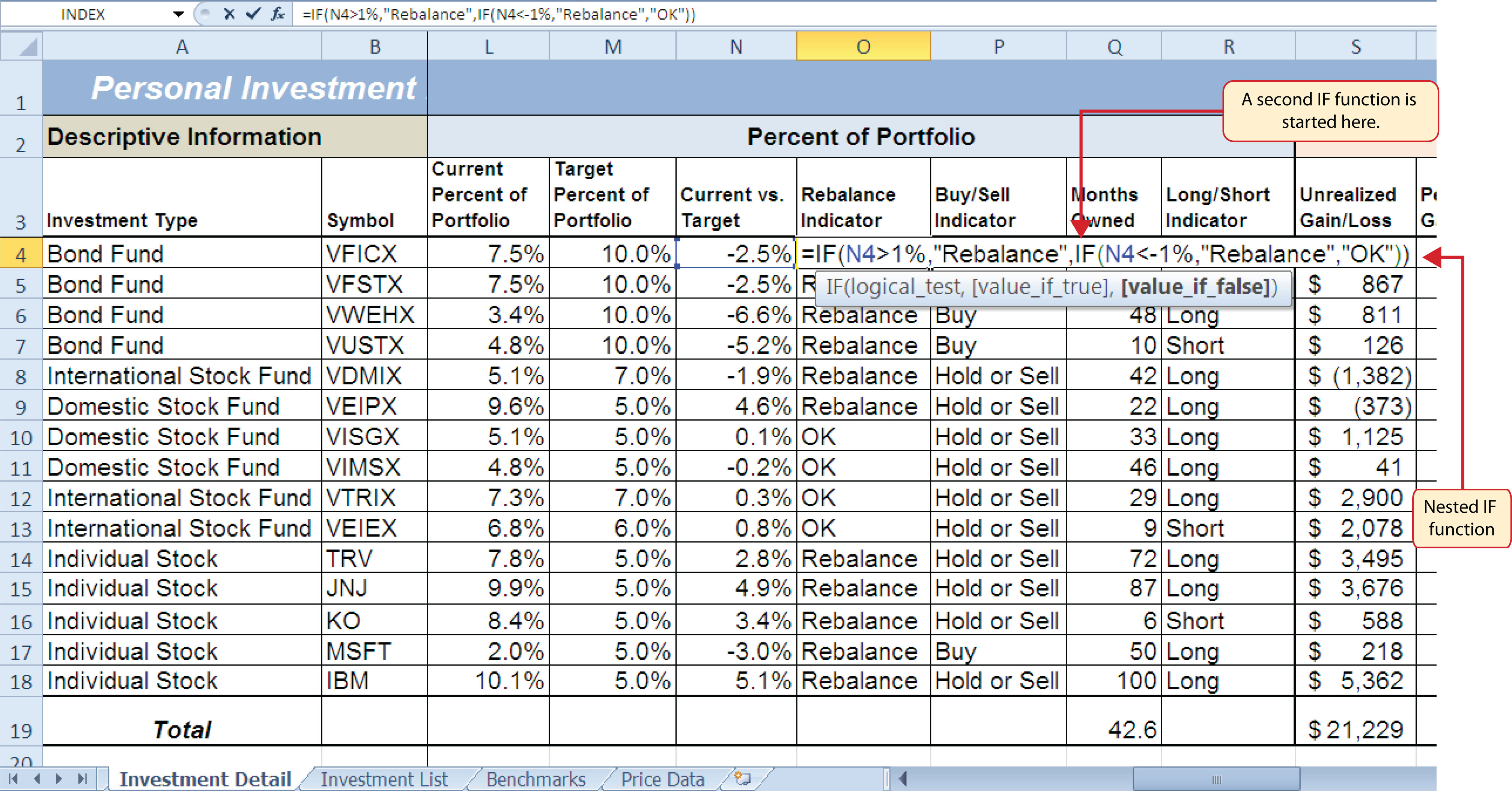### Why?

Use AND or OR functions within IF functions

The benefit of using the AND or OR functions within the IF function is that doing so reduces the need to construct lengthy nested IF functions. It becomes increasingly difficult to manage the accuracy of lengthy nested IF functions. The AND and OR functions allow you to test for a variety of conditions in a cell location, which can reduce the need to nest multiple IF functions. Examine the nested if function in cell O4 on the Investment Detail worksheet. Can you recreate this without nesting the IF function?

## Basic Conditional Formats

Follow-along file: Continue with Excel Objective 3.00. (Use file Excel Objective 3.07 if starting here.)

### Lesson Video: Basic Conditional Formats

A feature related to the skills used to create logical functions is conditional formatting. Conditional formatsAn Excel feature that applies formatting commands to cell locations based on the cell contents. A basic conditional formatting rule will utilize a logical test to evaluate the contents of a cell location. If the results of the logical test are true, Excel will apply the designated formatting commands to the cell location. allow you to apply a variety of formatting treatments based on the contents of a cell location. A logical test similar to the ones used in the IF, AND, and OR functions is used to evaluate the contents of a cell and apply a designated formatting treatment. For example, looking at Figure 3.20 "Completed Nested IF Function", you will notice that the Unrealized Gain/Loss column is formatted using the accounting number format. Negative numbers are enclosed in parentheses. However, to make these numbers stand out, we can use conditional formatting to change the font color to red. We will do this for the Unrealized Gain/Loss and Percent Gain/Loss columns. The following steps explain how conditional formats are applied to the cell locations in these columns:

1. Highlight the range S4:T18 on the Investment Detail worksheet.
2. Click the Conditional Formatting button in the Styles group of commands on the Home tab of the Ribbon.
3. Click the New Rule command from the list of options (see Figure 3.21 "Conditional Formatting Options List"). This will open the New Formatting Rule dialog box.

Figure 3.21 Conditional Formatting Options List4. At the top of the New Formatting Rule dialog box, you will find a list of options under the Select a Rule Type heading. Click the second option that states “Format only cells that contain.”
5. In the lower portions of the New Formatting Rule dialog box, you will see several drop-down boxes under the heading Edit the Rule Description. Make sure the first drop-down box is set to Cell Value.
6. Click the second drop-down box in the Edit the Rule Description section of the New Formatting Rule dialog box and select the “less than” option.
7. Click in the input box, which is next to the drop-down box that was set in the previous step, and type a zero. This completes the logical test of the conditional format, which is going to evaluate if the value in any of the cells in the range S4:T18 is less than zero.
8. Click the Format button, which is near the bottom of the New Formatting Rule dialog box. This will open the Format Cells dialog box.
9. Click the drop-down box in the Color section of the Format Cells dialog box and select the red square from the color palette (see Figure 3.22 "Format Cells Dialog Box").
10. Click the OK button at the bottom of the Format Cells dialog box.
11. Click the OK button at the bottom of the New Formatting Rule dialog box. This completes the Conditional Formatting rule that will be applied to cells in the range S4:T18.

Figure 3.22 "Format Cells Dialog Box" shows the Format Cells dialog box. This opens when the Format button is clicked on the New Formatting Rule dialog box. Notice the tabs running across the top of the dialog box. All formatting features in Excel are grouped by category, which can be accessed by clicking the related tab on the Format Cells dialog box. You will see some of the formatting commands in light grey. This indicates that these commands cannot be used with the Conditional Formatting feature. You can use the Format Cells dialog box to apply any formatting features by clicking the Format Cells dialog button on the Home tab of the Ribbon (see Figure 3.21 "Conditional Formatting Options List").

Figure 3.22 Format Cells Dialog Box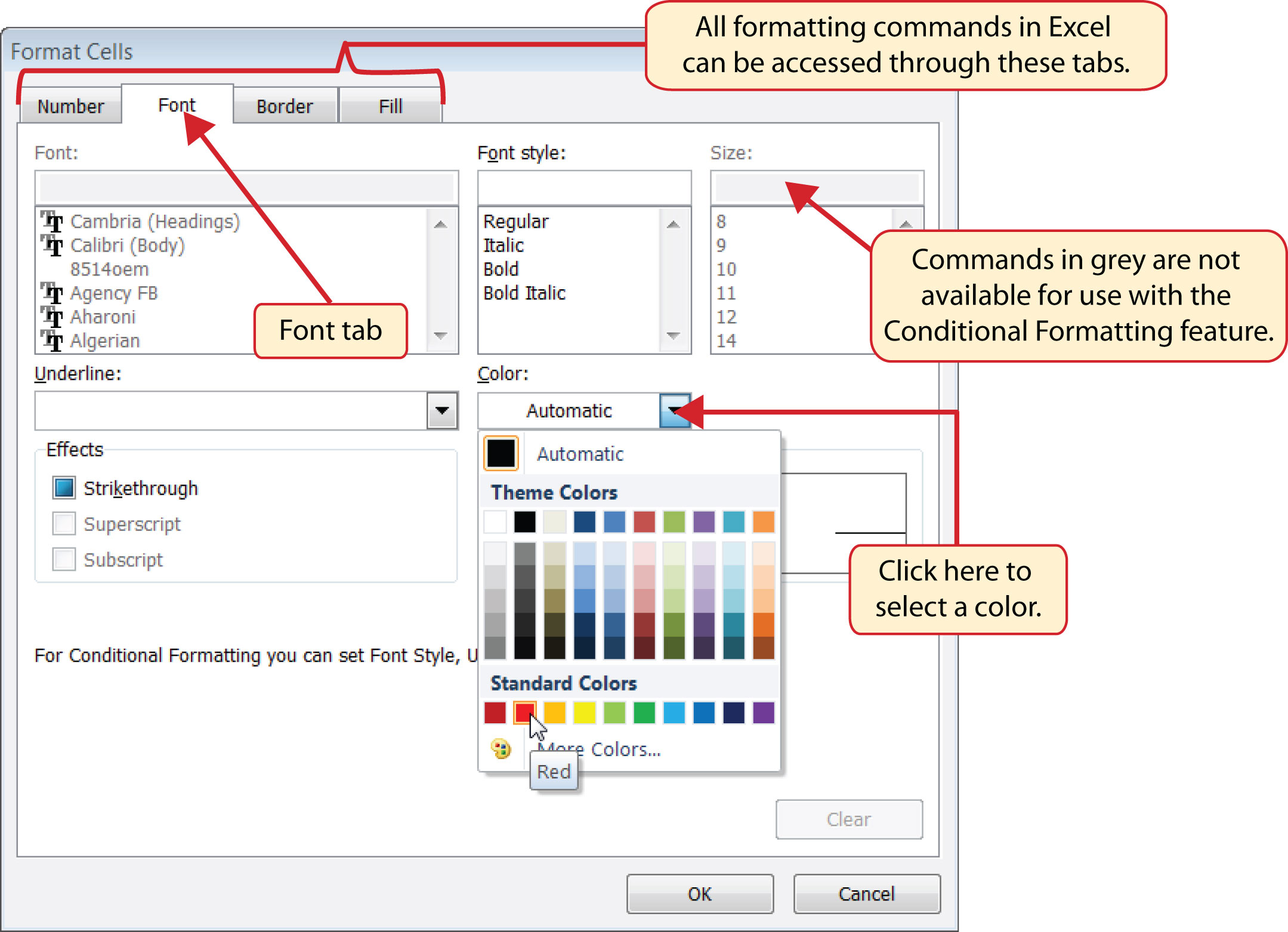### Mouseless Commands

Open the Format Cells Dialog Box

• Hold down the CTRL key while pressing the SHIFT key and the letter F key on your keyboard.

Figure 3.23 "New Formatting Rule Dialog Box" shows the final settings for the New Formatting Rule dialog box. It is important to note that the “Format only cells that contain” option was selected in the New Formatting Rule dialog box to set a basic logical test that can be used to apply formatting commands automatically based on the values in cell locations.

Figure 3.23 New Formatting Rule Dialog Box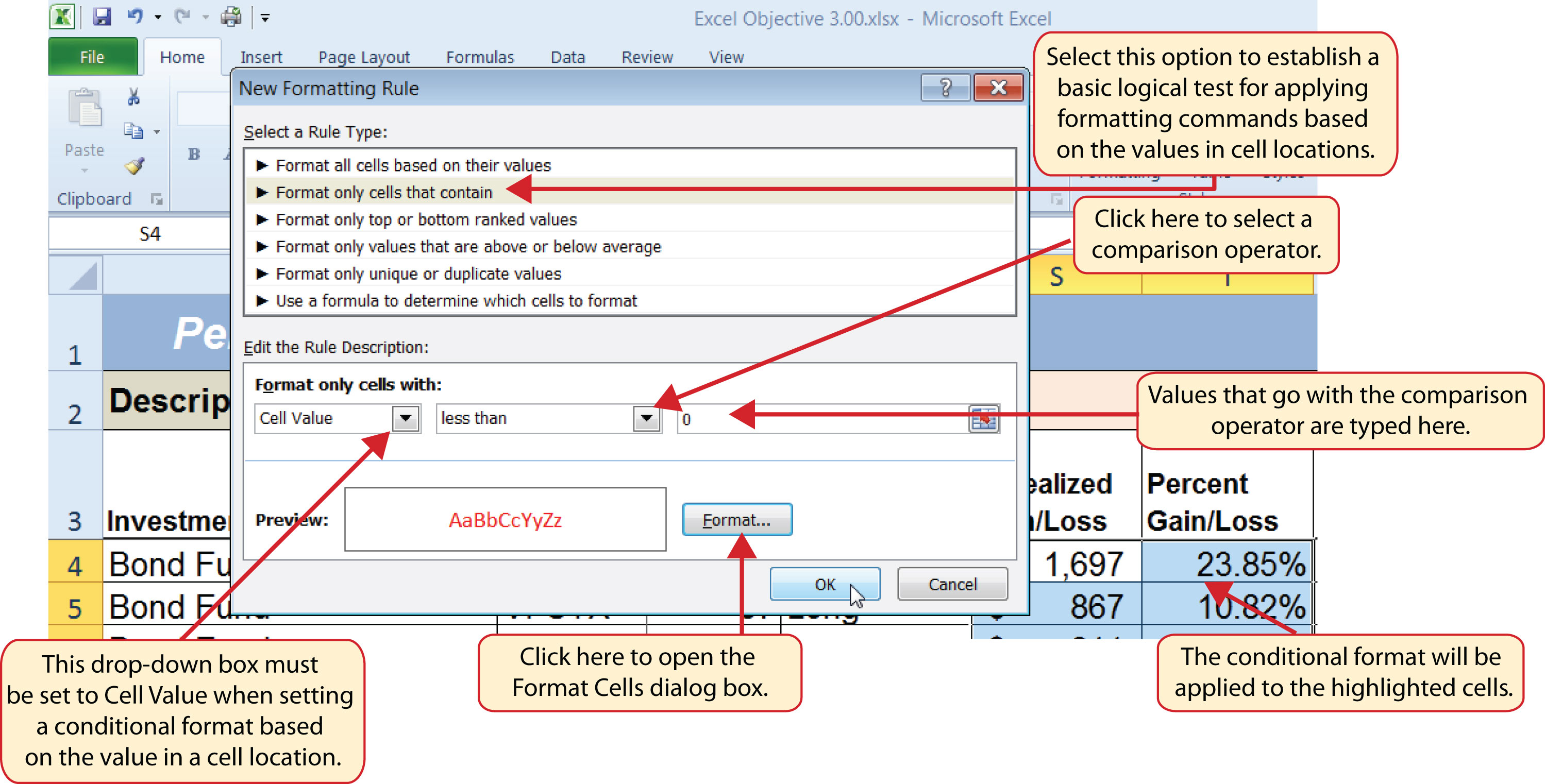Figure 3.24 "Conditional Format Applied to the Range S4:T18" shows the results of the conditional formatting rule that was applied to the range S4:T18. Notice the font color is automatically changed to red for negative numbers.

Figure 3.24 Conditional Format Applied to the Range S4:T18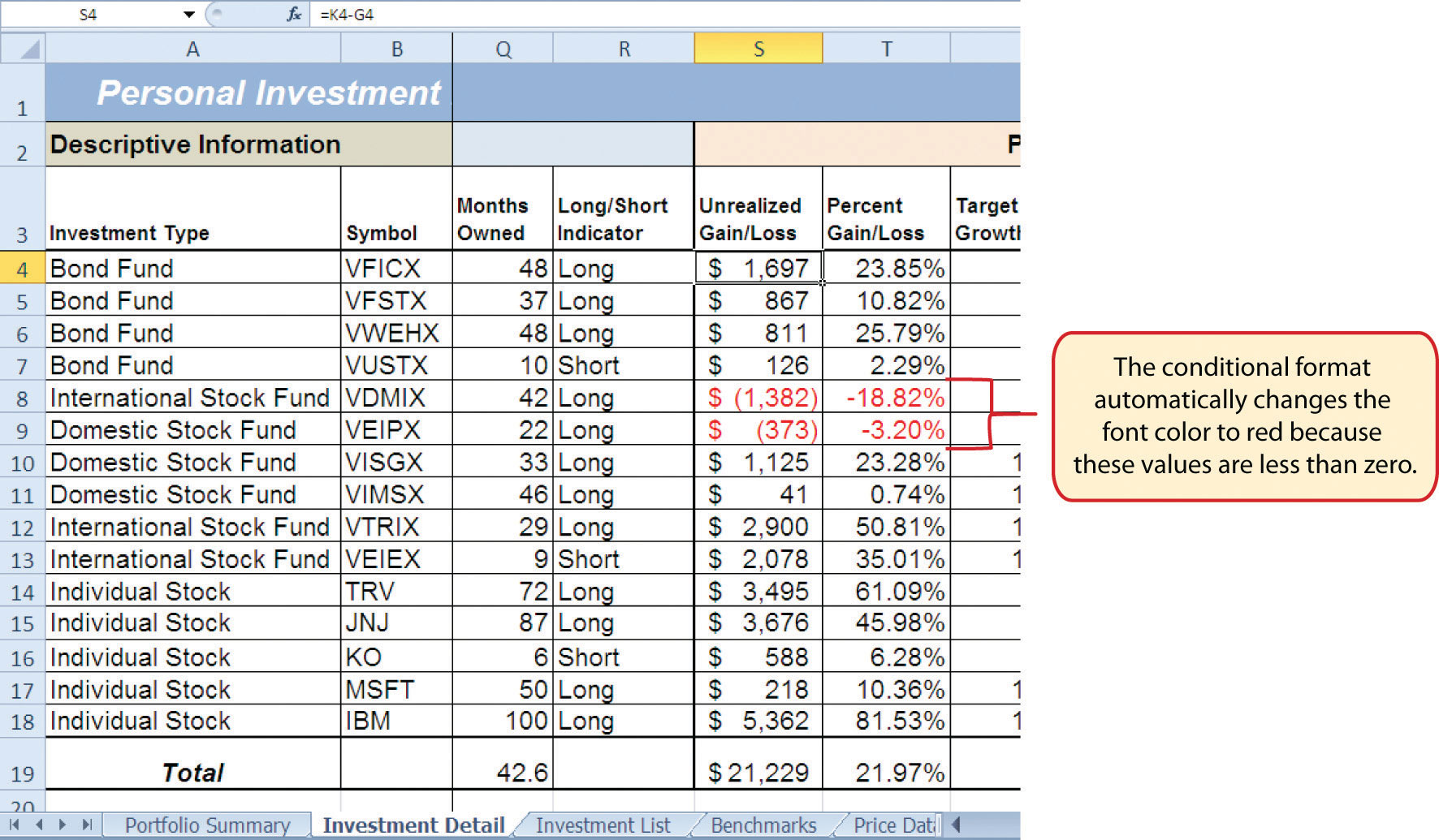### Skill Refresher: Conditional Formats (Cell Values)

1. Click a cell or highlight a range of cells where the conditional format will be applied.
2. Click the Home tab of the Ribbon.
3. Click the Conditional Formatting button.
4. Click the New Rule option from the drop-down list.
5. Click the “Format only cells that contain” rule type from the list at the top of the New Formatting Rule dialog box.
6. Select the type of contents you are evaluating in the first drop-down box near the bottom of the New Formatting Rule dialog box.
7. Select a comparison operator description in the second drop-down box near the bottom of the New Formatting Rule dialog box.
8. Enter a value in the input box next to the comparison operator box.
9. Click the Format button to set the format that will be applied to the selected cell locations.
10. Click the OK button at the bottom of the New Formatting Rule dialog box.

### Key Takeaways

• The Freeze Panes command should be used to lock column and row headings in place while scrolling through large worksheets.
• The IF function is used to evaluate the contents of a cell location using a logical test. Based on the results of the logical test, you designate a custom output or calculation to be performed by the function.
• When using text, or nonnumeric data, to define any argument of the IF function, it must be placed inside quotation marks.
• A nested IF function is used when more than one logical test and more than two outputs are required for a project. Either the Value_if_true or the Value_if_false arguments can be defined with an IF function.
• When using percentages in any logical test or formula, you must use the percent symbol (%) or convert the percentage to a decimal. For example, 10% can also be expressed as .10.
• The OR function is used when many logical tests are required to evaluate the contents of a cell location. The OR function will produce a TRUE output if one of the logical tests is true.
• The AND function is used when many logical tests are required to evaluate the contents of a cell location. The AND function will produce a TRUE output if all of the logical tests are true.
• To minimize the complexity of nested IF functions, the OR and AND functions should be used when possible to define the logical_test argument of the IF function.

### Exercises

1. Assume the value in cell B12 is 25. Any value greater than or equal to 25 is OK, and any value below 25 is too low. Which of the following IF functions will provide an accurate result?

1. =IF(B12>25,OK,TOO LOW)
2. =IF(B12>25, “TOO LOW”, “OK”)
3. =IF(B12=25 OR B12>25, “OK”, “TOO LOW”)
4. =IF(B12>=25, “OK”, “TOO LOW”)
2. Assume the value in cell C4 is 5 and the value in D4 is 2. If the value in C4 is greater than 10, or if the value in D4 is greater than or equal to 2, the output should read OK. Otherwise, the output should read LOW. Which of the following IF functions will provide an accurate result?

1. =IF(C4>10 or D4>2 or D4=2, “OK”, “LOW”)
2. =IF(OR(C4>10,D4>2,=2)=TRUE, “OK”, “LOW”)
3. =IF(OR(D4>=2,C4>10)=TRUE, “OK”, “LOW”)
4. =IF(C4>10, D4>=2, “OK”, “LOW”)
3. Assume the value in cell A2 is 0 and the value in B2 is 1%. If the value in A2 is equal to 0 and the value in B2 is greater than 1%, then the output of the function should be OK. Otherwise, the output of the function should be REBAL. Which of the following IF functions will provide an accurate result?

1. =IF(A2=0, “OK”,IF(B2>1%, “OK”, “REBAL”))
2. =IF(AND(A2=0,B2>1)=TRUE, “OK”, “REBAL”)
3. =IF(AND(A2=0,B2>.01)=TRUE, “OK”, “REBAL”)
4. Both a and c are correct.
4. Assume the value in cell E3 is 5. If the value in cell E3 is less than 0, the font color of the text should be red. If the value in cell E3 is greater than or equal to 0, the font color should remain black. When establishing a conditional format for cell E3, which rule type should be selected in the New Formatting Rule dialog box?

1. Format all cells based on their values
2. Format only cells that contain
3. Format only top or bottom ranked values
4. Use a formula to determine which cells to format

## 3.2 Statistical IF Functions

### Learning Objectives

1. Use the COUNTIF function to count selected nonblank cells in a range based on one criteria argument.
2. Use the AVERAGEIF function to calculate the average of selected cells in a range based on the values in an alternate range of cells and one criteria argument.
3. Use the SUMIF function to calculate the sum of selected cells in a range based on the values in an alternate range of cells and one criteria argument.
4. Use the COUNTIFS function to count selected nonblank cells in a range based on more than one criteria argument that utilizes a logical test.
5. Use the AVERAGEIFS function to calculate the average of selected cells in a range based on the values in an alternate range of cells using more than one criteria argument that utilizes a logical test.
6. Use the SUMIFS function to calculate the sum of selected cells in a range based on the values in an alternate range of cells using more than one criteria argument that utilizes a logical test.

This section will demonstrate the use of statistical IF functions. Statistical IF functionsStatistical functions that provide the ability to evaluate the contents in a cell location before including it in a mathematical calculation. Cell locations can be selected from a range based on specific criteria or a logical test. provide you with the ability to evaluate the contents in a cell location before including them in a mathematical calculation. This allows you to selectively include targeted cell locations when executing statistical calculations such as sum, average, count, and so on. We will use several statistical IF functions to construct the Portfolio Summary worksheet shown in Figure 3.1 "Completed Personal Investment Portfolio Workbook", which contains two main sections. The Total Summary section (Rows 2 through 8) shows an overview for all investments in the portfolio by investment type. This will allow us to compare the growth performance among the Bond Funds, Domestic Stock Funds, and others. The Poor Performing Investments section (Rows 9 through 15) will provide an overview of poor performing investments by investment type. For the purposes of this exercise, we will define a poor performing investment as one where the growth rate is below the target growth rate by more than 1% (see Column W on the Investment Detail worksheet). The statistical IF functions will allow us to establish criteria to select targeted investments that can be included in the calculations for each section of the Portfolio Summary worksheet.

## The COUNTIF Function

Follow-along file: Continue with Excel Objective 3.00. (Use file Excel Objective 3.08 if starting here.)

### Lesson Video: COUNTIF Function

The COUNTIF function differs from the regular COUNT function in two ways. First, the regular COUNT function counts only the number of cells in a range that contain numeric data. The COUNTIF function counts the number of cells in a range that contain numeric or text data. Second, the COUNTIF function allows you to selectively count the cells in a range based on specific criteria.

The COUNTIF function contains two arguments: range and criteria. The range argument is defined with the range of cells that will be counted. The criteria argument is defined with the criteria that will be used to decide if a cell in the range should be included in the output of the function. The following steps explain how we can use the COUNTIF function to calculate the number of investments by investment type on the Portfolio Summary worksheet:

1. Click cell B4 on the Portfolio Summary worksheet.
2. Click the Formulas tab of the Ribbon.
3. Click the More Functions button in the Function Library group of commands.
4. Place the mouse pointer over the Statistical option from the drop-down list.
5. Click the scroll down arrow on the second drop-down list to find the COUNTIF function (see Figure 3.25 "Selecting the COUNTIF Function from the Function Library").
6. Click the COUNTIF function. This will open the Function Arguments dialog box.

Figure 3.25 Selecting the COUNTIF Function from the Function Library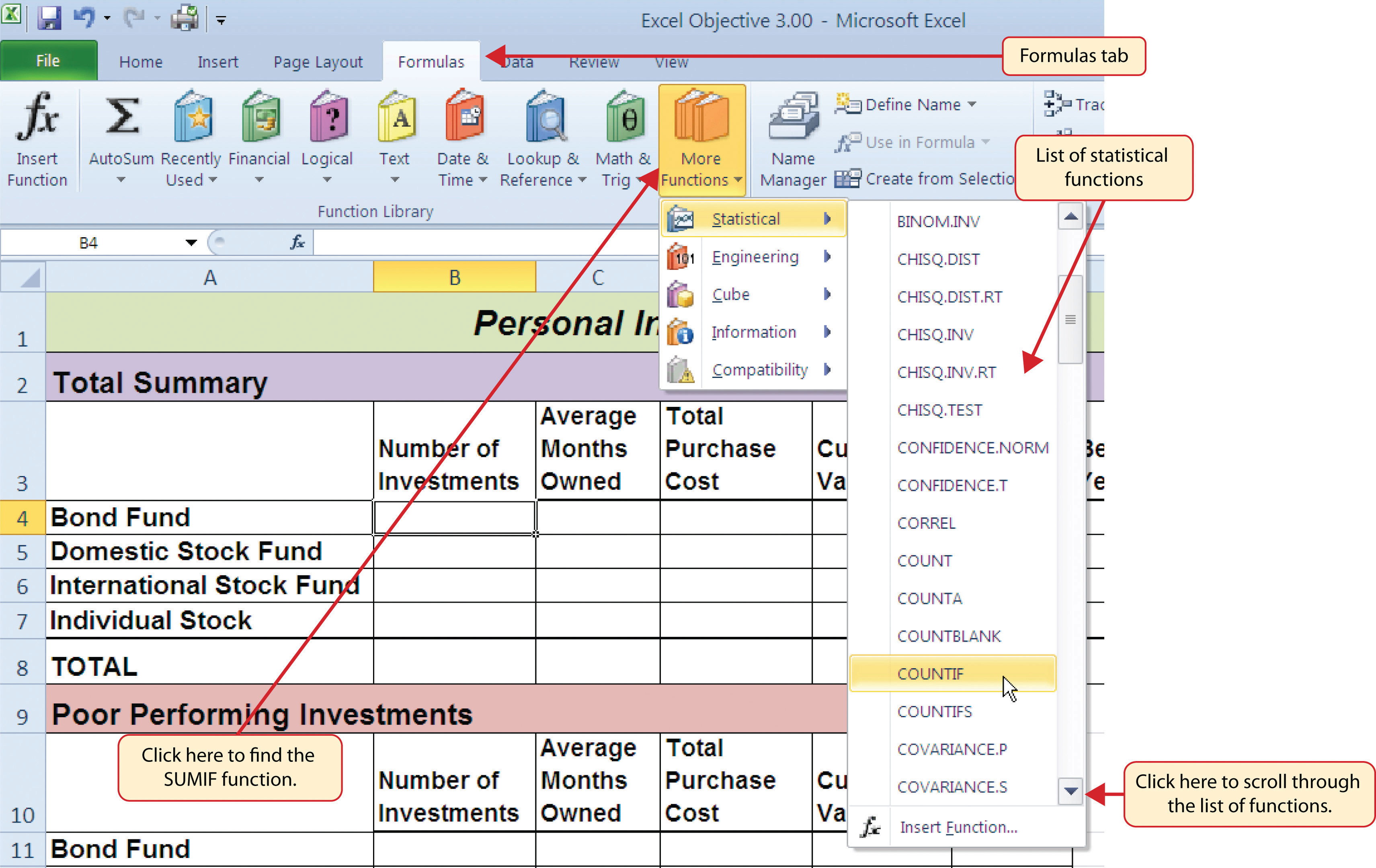7. Click the Collapse Dialog button next to the Range argument on the Function Arguments dialog box (see Figure 3.26 "Completed Function Arguments Dialog Box for the COUNTIF Function").
8. Click the Investment Detail worksheet tab.
9. Highlight the range A4:A18 on the Investment Detail worksheet and press the ENTER key on your keyboard.
10. Click in the Range argument input box and place an absolute reference on the range A4:A18. This is done by typing a dollar sign (\$) in front of the column letter and row number for both cells in the range. Alternatively, place the insertion point after the 4 in cell reference A4 and press the F4 key on your keyboard. Then place the insertion point after the 8 in cell reference A18 and press the F4 key. This will add the \$ to the appropriate positions automatically.
11. Press the TAB key on your keyboard to advance to the next argument, which is the Criteria argument. Then type the cell location A4. The criteria for the function will be the investment type entered into cell A4 on the Portfolio Summary worksheet.
12. Click the OK button at the bottom of the Function Arguments dialog box. Figure 3.26 "Completed Function Arguments Dialog Box for the COUNTIF Function" shows the completed Function Arguments dialog box for the COUNTIF function. Notice the absolute references that were placed on each cell location in the range that was used to define the Range argument. The Criteria argument is defined with the cell A4, which means the function will only count cell locations in the range A4:A18 where the contents in the cell match the contents in cell A4.

Figure 3.26 Completed Function Arguments Dialog Box for the COUNTIF Function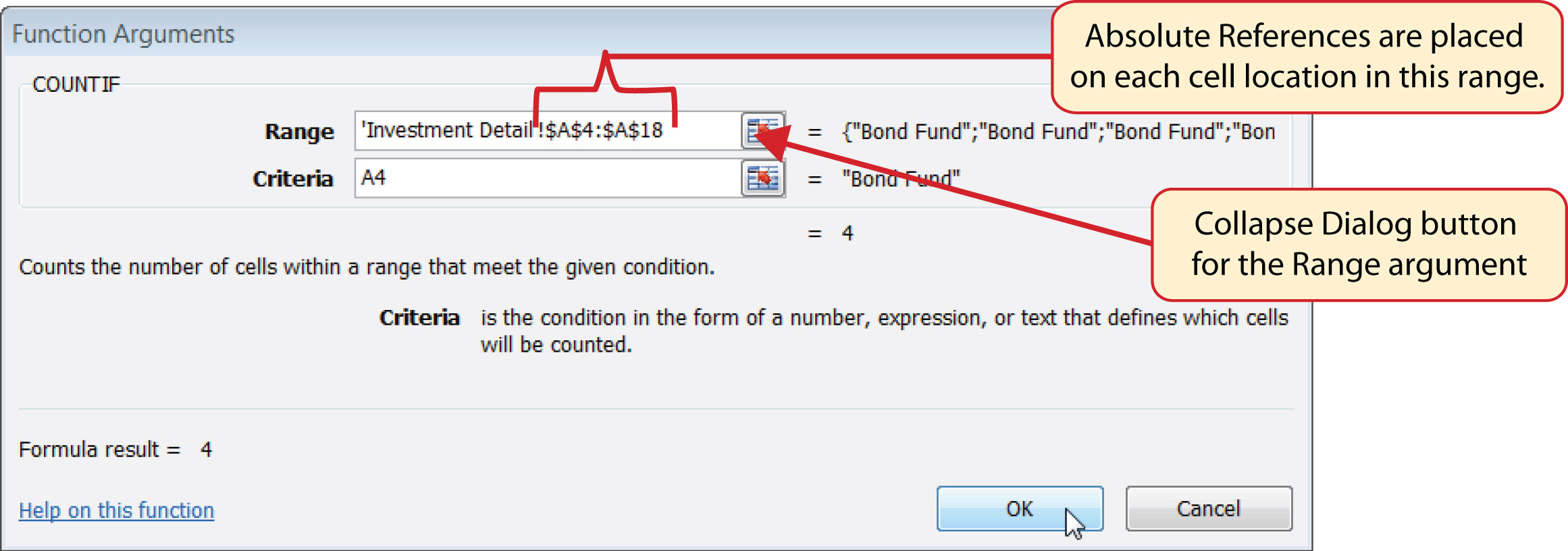13. Copy the function in cell B4 and paste it into the range B5:B7 using the Paste Formulas command.
14. Enter a SUM function in cell B8 that sums the values in the range B4:B7.

Figure 3.27 "COUNTIF Function Output in the Portfolio Summary Worksheet" shows the results of the COUNTIF function after it is pasted into the range B5:B7. Because of relative referencing, the cell location used in the criteria argument is changed after the function is pasted into the range B5:B7. For example, in cell B6, the function is counting the cell locations in the range A4:A18 where the contents match the contents of cell A6. This allows you to use the function to count the number of investments per investment type. As shown in the figure, the range B4:B7 now shows the number of investments in this portfolio by investment type.

Figure 3.27 COUNTIF Function Output in the Portfolio Summary Worksheet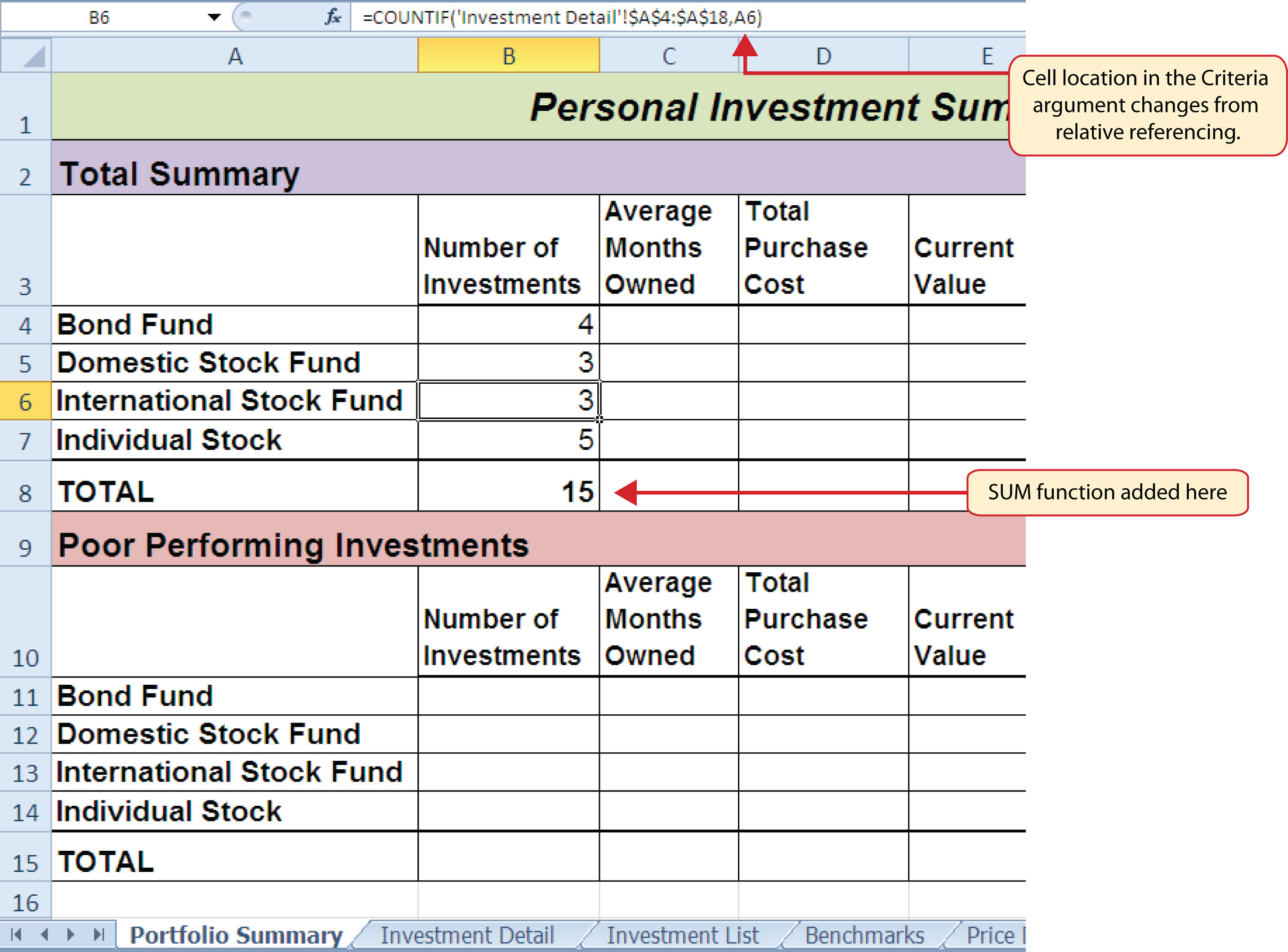### Skill Refresher: COUNTIF Function

1. Type an equal sign (=).
2. Type the function name COUNTIF followed by an open parenthesis (().
3. Define the range argument with a range of cells that will be counted.
4. Type a comma.
5. Define the criteria argument with a cell location, number, text, or logical test. Text and logical tests must be enclosed in quotation marks.
6. Type a closing parenthesis ()).
7. Press the ENTER key on your keyboard.

## The AVERAGEIF Function

Follow-along file: Continue with Excel Objective 3.00. (Use file Excel Objective 3.09 if starting here.)

### Lesson Video: AVERAGEIF Function

The AVERAGEIF function performs the identical mathematical calculation as the regular AVERAGE function. However, similar to the COUNTIF function, it allows you to define criteria that will select cells in a range that will be used in the function output. The AVERAGEIF function differs from the COUNTIF function in that it allows you to define two cell ranges instead of one. The first range pertains to the criteria that will be used to select cells for the function output. The second range contains the values that will be used to calculate the arithmetic mean. Table 3.8 "Arguments for the AVERAGEIF and SUMIF Functions" provides definitions for the arguments contained in the AVERAGEIF and SUMIF functions.

Table 3.8 Arguments for the AVERAGEIF and SUMIF Functions

Argument Definition
Range Range of cells that will be evaluated by the criteria argument.
Criteria Criteria that will be used to evaluate the range of cells that is used to define the Range argument. This argument can be defined with a cell location, formula, number, text, or logical test. Note that text and logical tests must be enclosed in quotation marks.
[Average_range] or [Sum_range] Range of cells that will be used to calculate the average when using the AVERAGEIF function, or the sum when using the SUMIF function. This argument is enclosed in brackets because it does not always need to be defined. If this argument is omitted, the function will use the range of cells in the Range argument to calculate the output.

The AVERAGEIF function will be used in the Portfolio Summary worksheet to calculate the average length of time that investments for each investment type are held. The following steps explain how to add this function to the worksheet:

1. Click cell C4 on the Portfolio Summary worksheet.
2. Click the Formulas tab on the Ribbon.
3. Click the More Functions button in the Function Library group of commands.
4. Place the mouse pointer over the Statistical option from the drop-down list (see Figure 3.25 "Selecting the COUNTIF Function from the Function Library").
5. Click the AVERAGEIF function, which will be near the top of the list of functions. This opens the Function Arguments dialog box.
6. Click the Collapse Dialog button next to the Range argument on the Function Arguments dialog box (see Figure 3.28 "Defined Arguments for the AVERAGEIF Function").
7. Click the Investment Detail worksheet tab.
8. Highlight the range A4:A18 on the Investment Detail worksheet and press the ENTER key on your keyboard.
9. Click in the Range argument input box and place an absolute reference on the range A4:A18. This is done by typing a dollar sign (\$) in front of the column letter and row number for both cells in the range.
10. Press the TAB key on your keyboard to advance to the Criteria argument and type the cell location A4. The criteria for the function will be the investment type entered into cell A4 on the Portfolio Summary worksheet.
11. Click the Collapse Dialog button next to the Average_range argument on the Function Arguments dialog box (see Figure 3.28 "Defined Arguments for the AVERAGEIF Function").
12. Click the Investment Detail worksheet tab.
13. Highlight the range Q4:Q18 on the Investment Detail worksheet and press the ENTER key on your keyboard.
14. Click in the Average_range argument input box and place an absolute reference on the range Q4:Q18. This is done by typing a dollar sign (\$) in front of the column letter and row number for both cells in the range.
15. Click the OK button at the bottom of the Function Arguments dialog box.

Figure 3.28 "Defined Arguments for the AVERAGEIF Function" shows the Function Arguments dialog box for the AVERAGEIF function that will be input into cell C4. Notice that absolute references are placed on the cell locations used to define the Range and Average_range arguments. The function will evaluate the cells in the range A4:A18 using the value that exists in cell A4 on the Portfolio Summary worksheet. When a cell in the range A4:A18 meets the criteria, the function will pull the cell location in the same row from the range Q4:Q18 and include it in the average calculation.

Figure 3.28 Defined Arguments for the AVERAGEIF Function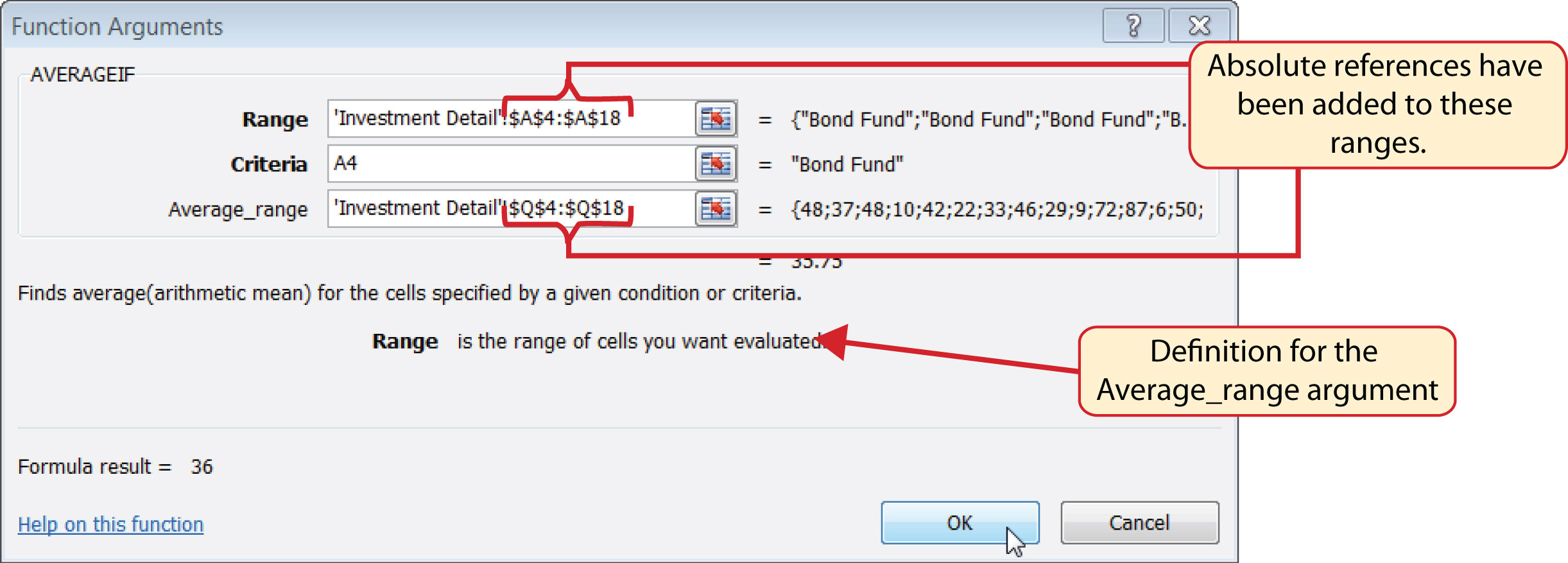16. Copy the function in cell C4 and paste it into the range C5:C7 using the Paste Formulas option.
17. Type an equal sign (=) in cell C8.
18. Click the Investment Detail worksheet tab. Then click cell Q19 and press the ENTER key on your keyboard.

Figure 3.29 "AVERAGEIF Function Output on the Portfolio Summary Worksheet" shows the output of the AVERAGEIF function in the Average Months Owned column on the Portfolio Summary worksheet. The function calculates the average months owned in Column Q on the Investment Detail worksheet where the investment type is equal to the description entered in the range A4:A7 on the Portfolio Summary worksheet.

Figure 3.29 AVERAGEIF Function Output on the Portfolio Summary Worksheet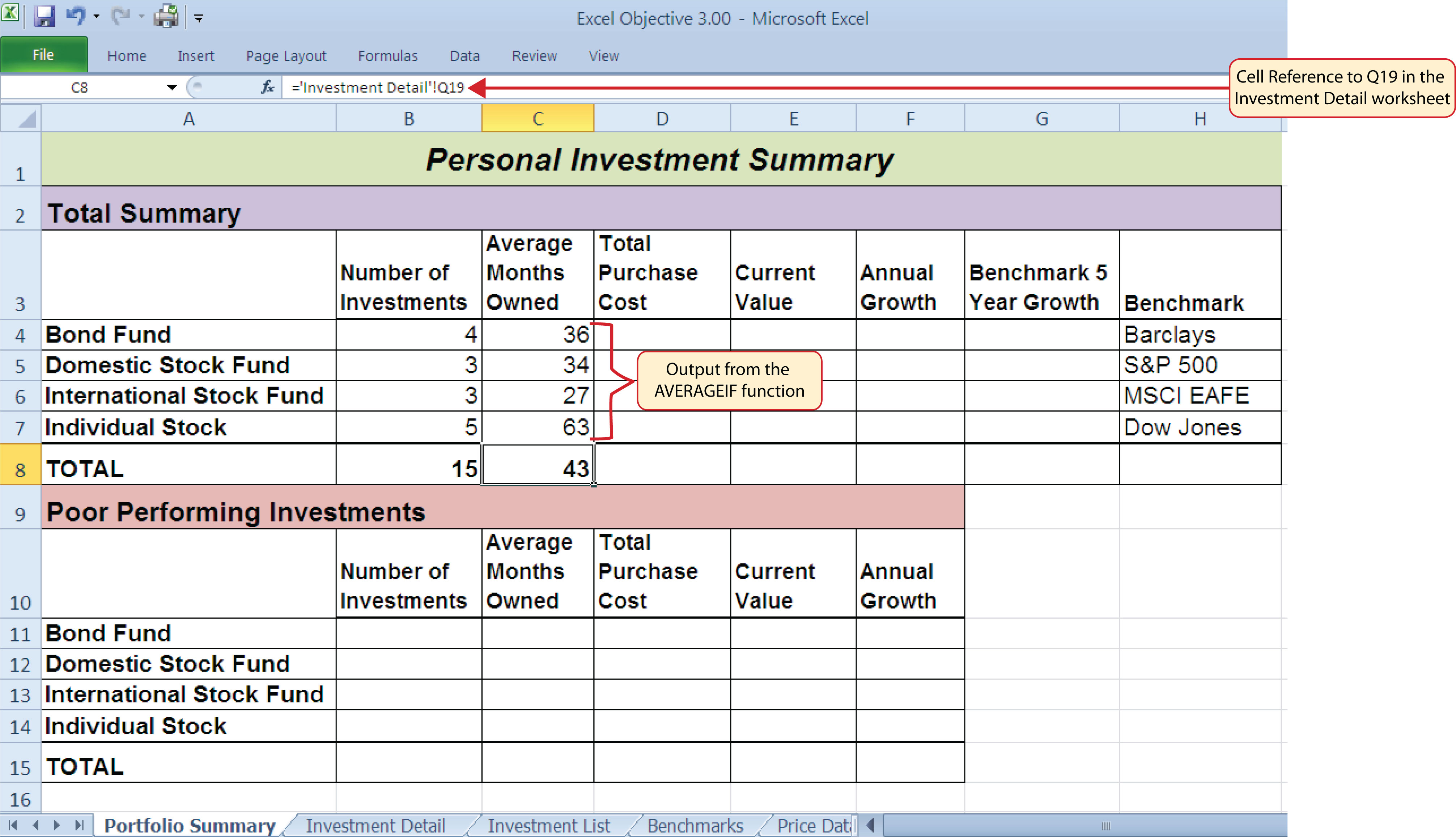### Integrity Check

Matching Row Numbers for the Range and Average_range (or Sum_range) Arguments

When defining the Average_range argument for the AVERAGEIF function or the Sum_range argument for the SUMIF function, it is good practice to make sure the row numbers match the row numbers used in the Range argument. For example, if the Range argument is defined with the range A4:A12, the range used to define the Average_range or Sum_range argument should begin with Row 4 and end with Row 12. If the row numbers in these two arguments do not match, Excel will include the values only in the rows used to define the Range argument. For example, if the Range argument is defined with the range A4:A12 and the Average_range (or Sum_range) argument is defined with the range D4:D20, only the values in cells D4:D12 will be included in the function output.

### Skill Refresher: AVERAGEIF Function

1. Type an equal sign (=).
2. Type the function name AVERAGEIF followed by an open parenthesis (().
3. Define the range argument with a range of cells that will be evaluated using the criteria argument.
4. Type a comma.
5. Define the criteria argument with a cell location, number, text, or logical test. Text and logical tests must be enclosed in quotation marks.
6. Type a comma.
7. Define the Average_range argument with a range that contains values to be averaged. Excel will use the range argument to calculate the average if this argument is omitted.
8. Type a closing parenthesis ()).
9. Press the ENTER key on your keyboard.

## The SUMIF Function

Follow-along file: Continue with Excel Objective 3.00. (Use file Excel Objective 3.10 if starting here.)

### Lesson Video: SUMIF Function

The SUMIF function performs the same mathematical calculation as the regular SUM function. However, similar to the AVERAGEIF function, this function allows you to select specific cells from a range that will be used in the output. The arguments for the SUMIF function are identical to the AVERAGEIF function (see Table 3.8 "Arguments for the AVERAGEIF and SUMIF Functions"). We will use the SUMIF function in two columns on the Portfolio Summary worksheet. The first column will show the total investment cost for each investment type. The second column will show the total current value for each investment type. This will allow us to calculate the total annual growth rate for each investment type. The following steps explain how we will use this function to complete the first column:

1. Click cell D4 on the Portfolio Summary worksheet.
2. Click the Formulas tab on the Ribbon.
3. Click the Math & Trig button in the Function Library group of commands (see Figure 3.25 "Selecting the COUNTIF Function from the Function Library").
4. Select the SUMIF function from the drop-down list. Use the scroll bar to scroll down to find the SUMIF function.
5. Click the Collapse Dialog button next to the Range argument on the Function Arguments dialog box (see Figure 3.30 "Defined Arguments for the First SUMIF Function on the Portfolio Summary Worksheet").
6. Click the Investment Detail worksheet tab.
7. Highlight the range A4:A18 on the Investment Detail worksheet and press the ENTER key on your keyboard.
8. Click in the Range argument input box and place an absolute reference on the range A4:A18. This is done by typing a dollar sign (\$) in front of the column letter and row number for both cells in the range.
9. Press the TAB key on your keyboard to advance to the Criteria argument and type the cell location A4. The criteria for the function will be the investment type entered into cell A4 on the Portfolio Summary worksheet.
10. Click the Collapse Dialog button next to the Sum_range argument on the Function Arguments dialog box (see Figure 3.30 "Defined Arguments for the First SUMIF Function on the Portfolio Summary Worksheet").

Figure 3.30 Defined Arguments for the First SUMIF Function on the Portfolio Summary Worksheet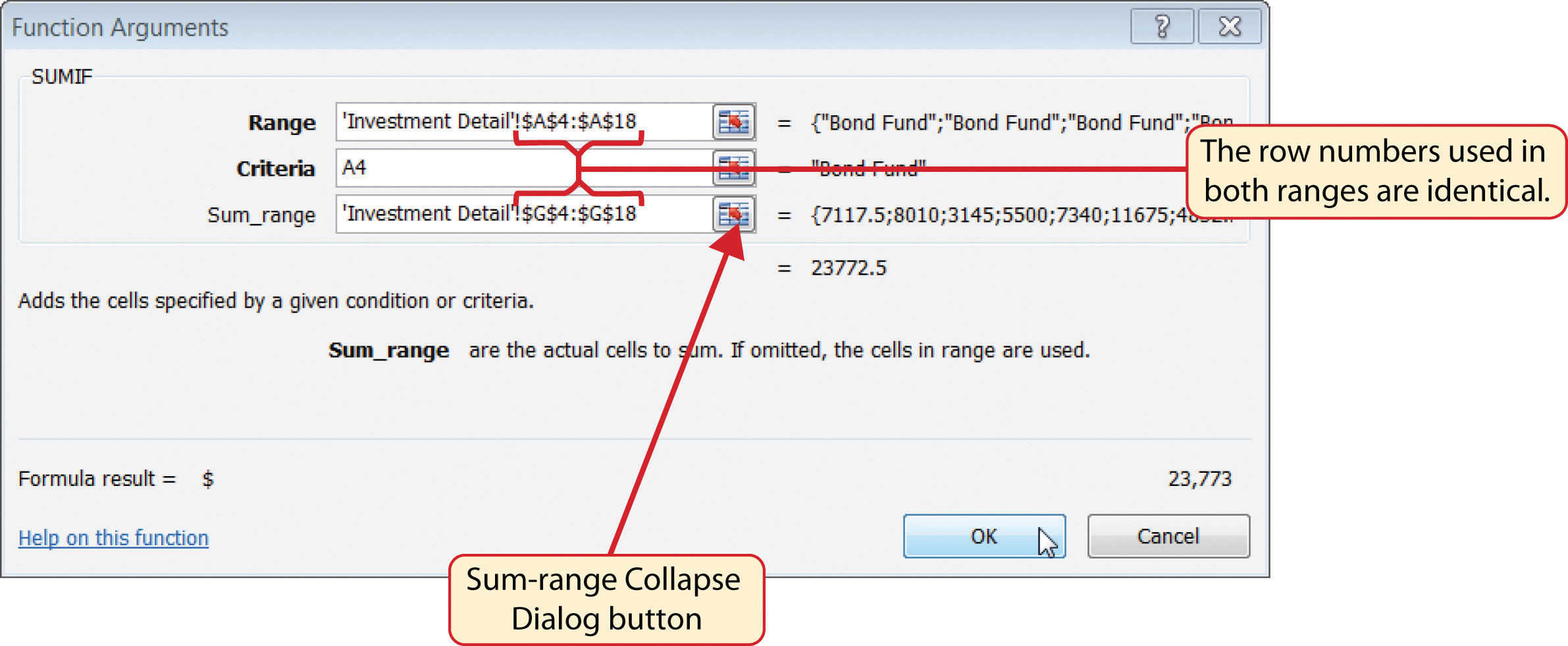11. Click the Investment Detail worksheet tab.
12. Highlight the range G4:G18 on the Investment Detail worksheet and press the ENTER key on your keyboard.
13. Click in the Sum_range argument input box and place an absolute reference on the range G4:G18. This is done by typing a dollar sign (\$) in front of the column letter and row number for both cells in the range.
14. Click the OK button at the bottom of the Function Arguments dialog box.
15. Copy the function in cell D4 and paste it into the range D5:D7 using the Paste Formulas option.
16. Enter a regular SUM function into cell D8 on the Portfolio Summary worksheet to calculate the sum of the values in the range D4:D7.

Figure 3.30 "Defined Arguments for the First SUMIF Function on the Portfolio Summary Worksheet" shows how the SUMIF arguments were defined for the Total Purchase Cost column on the Portfolio Summary worksheet. Notice that the row numbers are identical in the range used to define the Range argument and the Sum_range argument.

The following steps explain how to add the SUMIF function to the second column on the Portfolio Summary worksheet:

1. Click cell E4 on the Portfolio Summary worksheet.
2. Click the Formulas tab on the Ribbon.
3. Click the Math & Trig button in the Function Library group of commands (see Figure 3.25 "Selecting the COUNTIF Function from the Function Library").
4. Select the SUMIF function from the drop-down list. Use the scroll bar to scroll down to find the SUMIF function.
5. Click the Collapse Dialog button next to the Range argument on the Function Arguments dialog box (see Figure 3.30 "Defined Arguments for the First SUMIF Function on the Portfolio Summary Worksheet").
6. Click the Investment Detail worksheet tab.
7. Highlight the range A4:A18 on the Investment Detail worksheet and press the ENTER key on your keyboard.
8. Click in the Range argument input box and place an absolute reference on the range A4:A18. This is done by typing a dollar sign (\$) in front of the column letter and row number for both cells in the range.
9. Press the TAB key on your keyboard to advance to the Criteria argument and type the cell location A4.
10. Click the Collapse Dialog button next to the Sum_range argument on the Function Arguments dialog box (see Figure 3.30 "Defined Arguments for the First SUMIF Function on the Portfolio Summary Worksheet").
11. Click the Investment Detail worksheet tab.
12. Highlight the range K4:K18 on the Investment Detail worksheet and press the ENTER key on your keyboard.
13. Click in the Sum_range argument input box and place an absolute reference on the range K4:K18. This is done by typing a dollar sign (\$) in front of the column letter and row number for both cells in the range.
14. Click the OK button at the bottom of the Function Arguments dialog box.
15. Copy the function in cell E4 and paste it into the range E5:E7 using the Paste Formulas option.
16. Enter a regular SUM function into cell E8 on the Portfolio Summary worksheet to calculate the sum of the values in the range E4:E7.

Figure 3.31 "SUMIF Function Outputs in the Portfolio Summary Worksheet" shows the results of the SUMIF function in the Total Purchase Cost and Current Value columns in the Portfolio Summary worksheet.

Figure 3.31 SUMIF Function Outputs in the Portfolio Summary Worksheet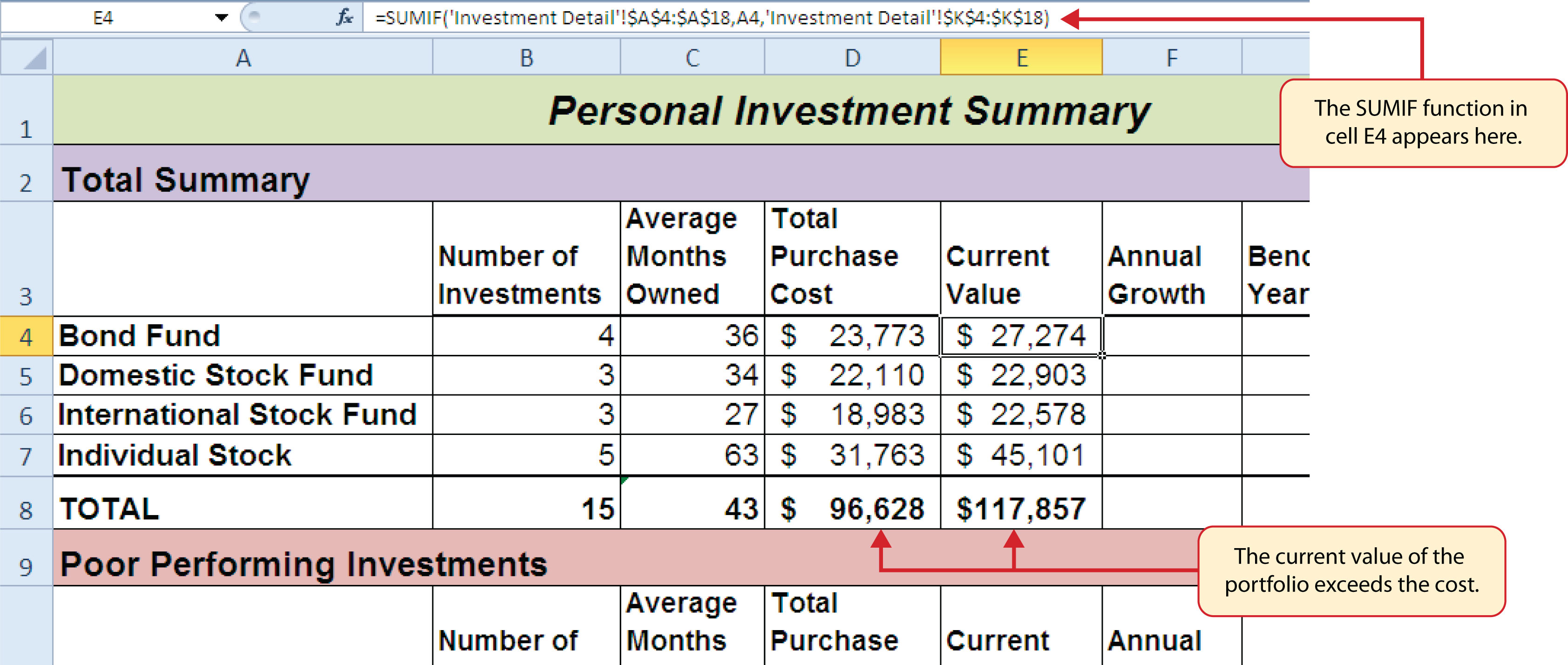A formula can now be added to show the annual growth for each investment category. The following steps explain how to add this formula to the Portfolio Summary worksheet:

1. Click cell F4 on the Portfolio Summary worksheet.
2. Type an equal sign (=) followed by two open parenthesis ((().
3. Click cell E4 and type a minus sign ().
4. Click cell D4 and type a closing parenthesis ()).
5. Type a slash (/) for division and click cell D4.
6. Type a closing parenthesis ()). This completes the first part of the formula, which is calculating the growth rate between the Total Purchase Cost (cell D4) and the Current Value (cell E4).
7. Type a slash (/) for division followed by an open parenthesis (().
8. Click cell C4, which is the Average Months Owned.
9. Type a slash (/) for division and the number 12. This part of the formula converts the number of months owned to years by dividing it by 12. This result is being divided into the growth rate, which will then show the average growth per year.
10. Type a closing parenthesis ()) and press the ENTER key on your keyboard.
11. Copy the formula in cell F4 and paste it into the range F5:F8 using the Paste Formulas command.

Figure 3.32 "Completed Annual Growth Column in the Portfolio Summary Worksheet" shows the results of the statistical IF functions that were added to the Total Summary section of the Portfolio Summary worksheet. The statistical IF functions used on this worksheet allowed us to group the details in the Investment Detail worksheet by investment type. Once this was accomplished, we added a formula to show the annual growth rate by investment type.

Figure 3.32 Completed Annual Growth Column in the Portfolio Summary Worksheet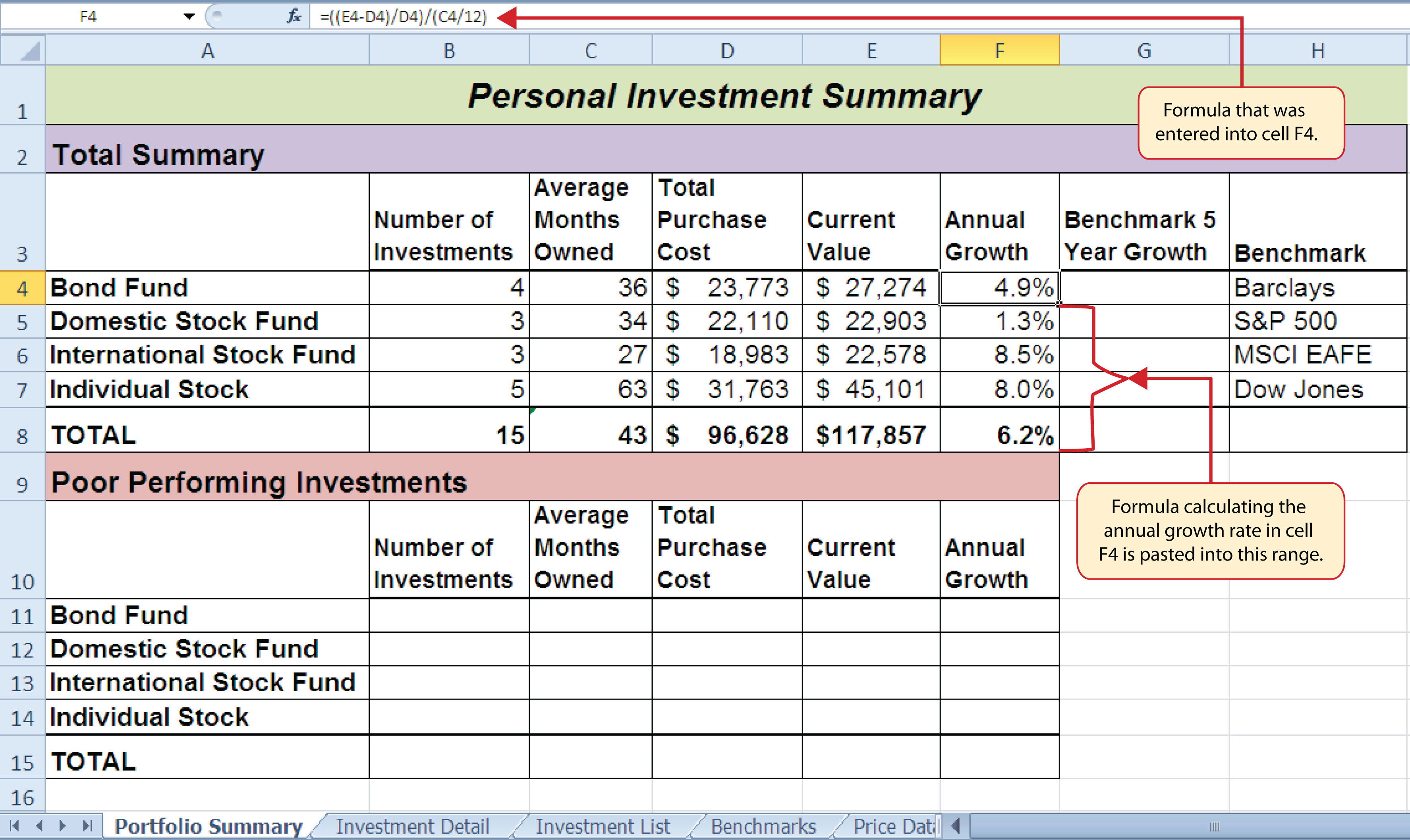### Skill Refresher: SUMIF Function

1. Type an equal sign (=).
2. Type the function name SUMIF followed by an open parenthesis (().
3. Define the range argument with a range of cells that will be evaluated using the criteria argument.
4. Type a comma.
5. Define the criteria argument with a cell location, number, text, or logical test. Text and logical tests must be enclosed in quotation marks.
6. Type a comma.
7. Define the Sum_range argument with a range that contains values to be summed. Excel will use the range argument to calculate the sum if this argument is omitted.
8. Type a closing parenthesis ()).
9. Press the ENTER key on your keyboard.

## The COUNTIFS Function

Follow-along file: Continue with Excel Objective 3.00. (Use file Excel Objective 3.11 if starting here.)

### Lesson Video: COUNTIFS Function

Up to this point, the statistical IF functions that were demonstrated provided the ability to define one criteria or logical test used to select cells from a targeted range. The next set of statistical functions that will be demonstrated provides the ability to define multiple sets of criteria for selecting cells from a targeted range. We will begin with the COUNTIFS function.

It is easy to distinguish the difference between a statistical IF function that allows one criteria argument to be defined and one that allows multiple criteria arguments. If the IF at the end of the function name is plural, you can define multiple sets of criteria arguments. Therefore, the COUNTIFS function provides the option of defining multiple sets of criteria for selecting cells from a targeted range that will be used in the function output. The arguments for the COUNTIFS function are established in pairs. For example, the first arguments for the function are Criteria_range1 and Criteria1. The function will use the Criteria1 argument to select cells in the Criteria_range1 argument. A second pair of arguments, Criteria_range2 and Criteria2, can be defined to select a subset of cell locations that were selected in the Criteria_range1 and Criteria1 arguments. This process can be repeated for several pairs of criteria arguments. We will continue to work on the Portfolio Summary worksheet by adding the COUNTIFS function to count the number of poor performing investments by investment type. The following steps explain how to add this function to the worksheet:

1. Click cell B11 on the Portfolio Summary worksheet.
2. Click the Formulas tab of the Ribbon.
3. Click the More Functions button in the Function Library group of commands.
4. Place the mouse pointer over the Statistical option from the drop-down list.
5. Click the scroll down arrow on the second drop-down list to find the COUNTIFS function (see Figure 3.25 "Selecting the COUNTIF Function from the Function Library").
6. Click the COUNTIFS function. This will open the Function Arguments dialog box.
7. Click the Collapse Dialog button next to the Criteria_range1 argument on the Function Arguments dialog box (see Figure 3.33 "Defined Arguments for the COUNTIFS Function").
8. Click the Investment Detail worksheet tab.
9. Highlight the range A4:A18 on the Investment Detail worksheet and press the ENTER key on your keyboard.
10. Click in the Criteria_range1 argument input box and place an absolute reference on the range A4:A18. This is done by typing a dollar sign (\$) in front of the column letter and row number for both cells in the range.
11. Press the TAB key on your keyboard to advance to the Criteria1 argument and type the cell location A11. The criteria for the function will be the investment type that is entered into cell A11 on the Portfolio Summary worksheet. You will notice that when you define this argument, the Criteria_range2 argument will appear on the Function Arguments dialog box.
12. Click the Collapse Dialog button next to the Criteria_range2 argument on the Function Arguments dialog box (see Figure 3.33 "Defined Arguments for the COUNTIFS Function").
13. Click the Investment Detail worksheet tab.
14. Highlight the range W4:W18 on the Investment Detail worksheet and press the ENTER key on your keyboard.
15. Click in the Criteria_range2 argument input box and place an absolute reference on the range W4:W18. This is done by typing a dollar sign (\$) in front of the column letter and row number for both cells in the range.
16. Press the TAB key on your keyboard to advance to the Criteria2 argument.
17. Type an open quotation mark followed by the logical test <−1% and then type a closing quotation mark (“<−1%”). The second criterion for this function is a logical test that will identify cell locations where the value is less than −1%. For the purposes of this exercise, a poor performing investment is one that is below the target growth rate by more than 1%.
18. Click the OK button at the bottom of the Function Arguments dialog box.
19. Copy the function in cell B11 and paste it into the range B12:B14 using the Paste Formulas command.
20. Enter a SUM function in cell B15 on the Portfolio Summary worksheet that sums the values in the range B11:B14.

### Why?

Use Statistical IF Functions for a Summary Worksheet

When creating a summary worksheet that summarizes detailed data from other worksheets, such as the Portfolio Summary worksheet in Figure 3.32 "Completed Annual Growth Column in the Portfolio Summary Worksheet", it is best to use statistical IF functions. If data is added to the detailed worksheet that is being summarized, the statistical IF functions will automatically include the new data in the summary worksheet. For example, suppose a row is added below Row 7 on the Investment Detail worksheet (see Figure 3.24 "Conditional Format Applied to the Range S4:T18") and another bond investment is added. The statistical IF functions will automatically pick up the new investment and include it in the Portfolio Summary worksheet. If regular statistical functions or formulas are used, the summary worksheet can easily become inaccurate if new data is added to the detailed worksheet or if the sort order is changed.

Figure 3.33 "Defined Arguments for the COUNTIFS Function" shows the completed arguments for the COUNTIFS function in the Function Arguments dialog box. Notice the scroll bar that appears on the right side of the Function Arguments dialog box. This allows you to scroll through and define multiple pairs of criteria arguments for the function.

Figure 3.33 Defined Arguments for the COUNTIFS Function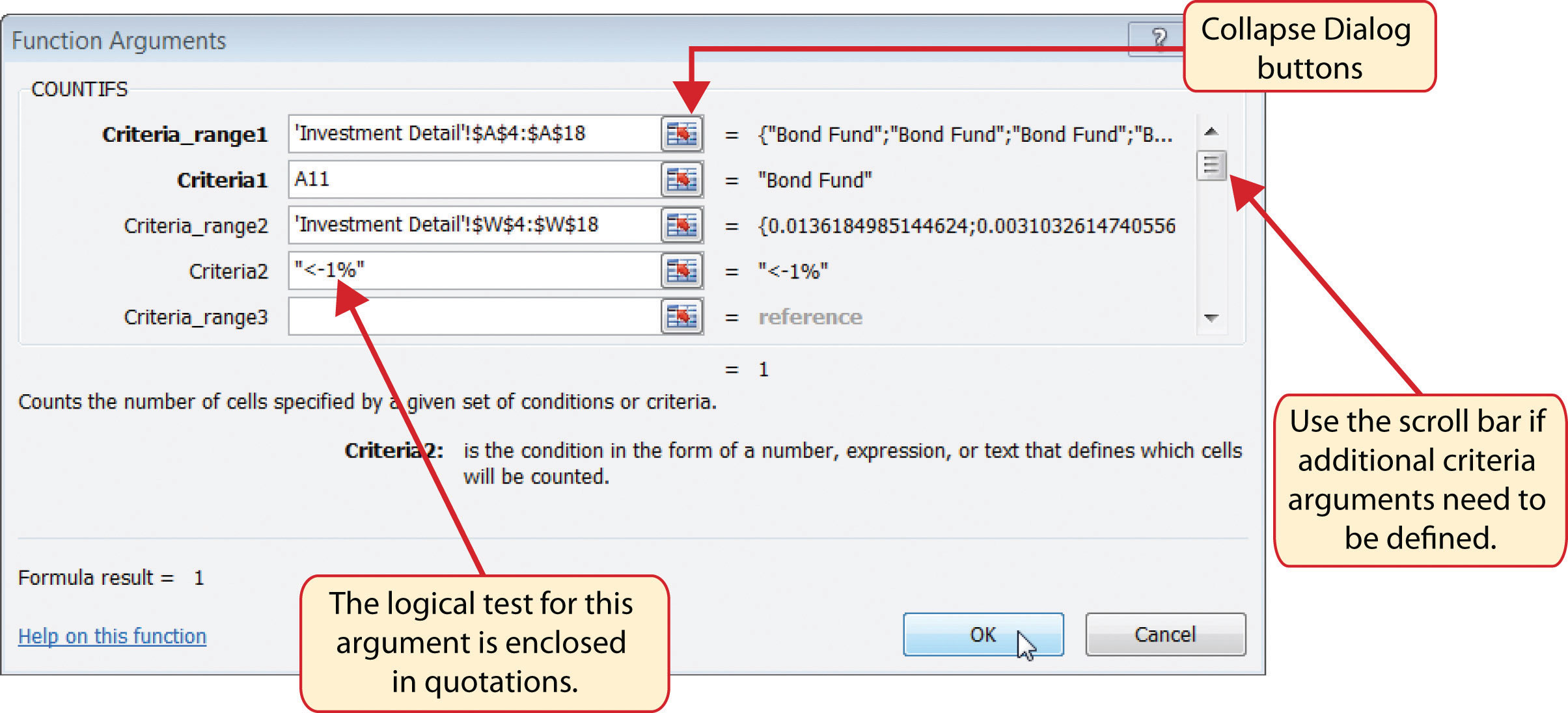Figure 3.34 "Outputs for the COUNTIFS Functions" shows the output of the COUNTIFS function on the Portfolio Summary worksheet. The criteria established for the COUNTIFS function shows that there are a total of seven investments that are underperforming in the portfolio.

Figure 3.34 Outputs for the COUNTIFS Functions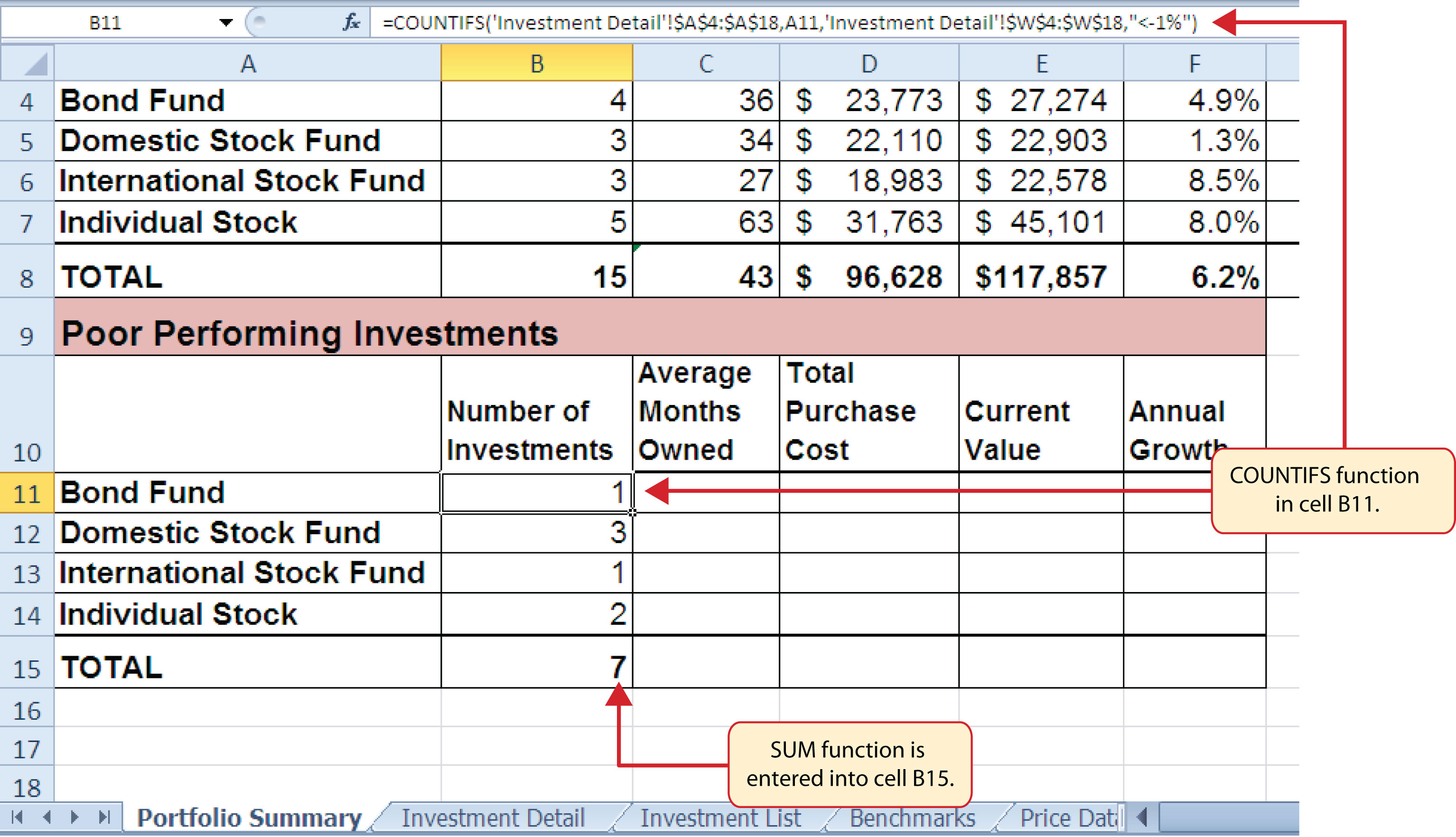### Skill Refresher: COUNTIFS Function

1. Type an equal sign (=).
2. Type the function name COUNTIFS followed by an open parenthesis (().
3. Define the Criteria_range1 argument with a range of cells that will be counted.
4. Type a comma.
5. Define the Criteria1 argument with a cell location, number, text, or logical test. Text and logical tests must be enclosed in quotation marks. This argument will be used to select cells from the Criteria_range1 argument.
6. Type a comma and then repeat steps 3, 4, and 5 to define as many pairs of arguments as needed.
7. Type a closing parenthesis ()).
8. Press the ENTER key on your keyboard.

## The AVERAGEIFS Function

Follow-along file: Continue with Excel Objective 3.00. (Use file Excel Objective 3.12 if starting here.)

### Lesson Video: AVERAGEIFS Function

The AVERAGEIFS function is similar to the COUNTIFS function in that multiple sets of criteria can be defined instead of one. However, the arguments for the AVERAGEIFS function are slightly different from those for the COUNTIFS function. Table 3.9 "Arguments for the AVERAGEIFS and SUMIFS Functions" provides definitions for the arguments of the AVERAGEIFS and the SUMIFS functions.

Table 3.9 Arguments for the AVERAGEIFS and SUMIFS Functions

Argument Definition
Average_range or Sum_range Range of cells that contain values to be averaged when using the AVERAGEIFS function or summed when using the SUMIFS function. Note that the AVERAGEIFS or SUMIFS functions will only select values from the range used to define this argument if all criteria pairs are true.
Criteria_range1 Range of cells that will be evaluated based on the Criteria1 argument to determine which cells in the Average_range or Sum_range arguments will be included in the output of the function.
Criteria1 Criteria that will be used to evaluate the range of cells used to define the Criteria_range1 argument. This argument can be defined with a cell location, formula, number, text, or logical test. Note that text and logical tests must be enclosed in quotation marks.
Criteria_range2 Optional argument that defines a second range of cells that will be evaluated based on the Criteria2 argument to determine which cells in the Average_range or Sum_range arguments will be included in the output of the function. Additional Criteria_rangeN arguments can be defined as needed.
Criteria2 Criteria that will be used to evaluate the range of cells used to define the Criteria_range2 argument. This argument can be defined with a cell location, formula, number, text, or logical test. Note that text and logical tests must be enclosed in quotation marks. Additional CriteriaN arguments can be defined as needed.

The AVERAGEIFS function will be used to calculate the average months of ownership for poor performing investments in the portfolio. The following steps explain how to add this function to the Portfolio Summary worksheet:

1. Click cell C11 on the Portfolio Summary worksheet.
2. Click the Formulas tab on the Ribbon.
3. Click the More Functions button in the Function Library group of commands.
4. Place the mouse pointer over the Statistical option from the drop-down list (see Figure 3.25 "Selecting the COUNTIF Function from the Function Library").
5. Click the AVERAGEIFS function near the top of the list of functions. This will open the Function Arguments dialog box.
6. Click the Collapse Dialog button next to the Average_range argument on the Function Arguments dialog box (see Figure 3.35 "Completed Arguments for the AVERAGEIFS Function").
7. Click the Investment Detail worksheet tab.
8. Highlight the range Q4:Q18 on the Investment Detail worksheet and press the ENTER key on your keyboard. Selected cells from this range will be averaged by the function based on the defined criteria in ensuing arguments.
9. Click in the Average_range argument input box and place an absolute reference on the range Q4:Q18. This is done by typing a dollar sign (\$) in front of the column letter and row number for both cells in the range.
10. Click the Collapse Dialog button next to the Criteria_range1 argument on the Function Arguments dialog box (see Figure 3.35 "Completed Arguments for the AVERAGEIFS Function").
11. Click the Investment Detail worksheet tab.
12. Highlight the range A4:A18 on the Investment Detail worksheet and press the ENTER key on your keyboard. Once the Criteria_range1 argument is defined, the Criteria1 argument will appear in the Function Arguments dialog box.
13. Click in the Criteria_range1 argument input box and place an absolute reference on the range A4:A18. This is done by typing a dollar sign (\$) in front of the column letter and row number for both cells in the range.
14. Press the TAB key on your keyboard to advance to the Criteria1 argument and type the cell location A11. The criteria for the function will be the investment type entered into cell A11 on the Portfolio Summary worksheet. You will notice that as you define this argument, the Criteria_range2 argument will appear on the Function Arguments dialog box.
15. Click the Collapse Dialog button next to the Criteria_range2 argument on the Function Arguments dialog box (see Figure 3.35 "Completed Arguments for the AVERAGEIFS Function").
16. Click the Investment Detail worksheet tab.
17. Highlight the range W4:W18 on the Investment Detail worksheet and the press the ENTER key on your keyboard.
18. Click in the Criteria_range2 argument input box and place an absolute reference on the range W4:W18. This is done by typing a dollar sign (\$) in front of the column letter and row number for both cells in the range.
19. Press the TAB key on your keyboard to advance to the Criteria2 argument.
20. Type an open quotation mark followed by the logical test <−1% and then type a closing quotation mark (“<−1%”). The second criterion for this function is a logical test that will identify cell locations where the value is less than −1%. For the purposes of this exercise, a poor performing investment is one that is below the target growth rate by more than 1%.
21. Click the OK button at the bottom of the Function Arguments dialog box.
22. Copy the function in cell C11 and paste it into the range C12:C14 using the Paste Formulas command.

Figure 3.35 "Completed Arguments for the AVERAGEIFS Function" shows the defined arguments for the AVERAGEIFS function. Notice that two sets of criteria arguments are defined. Values that meet all criteria defined in the function will be selected from the range Q4:Q18.

Figure 3.35 Completed Arguments for the AVERAGEIFS Function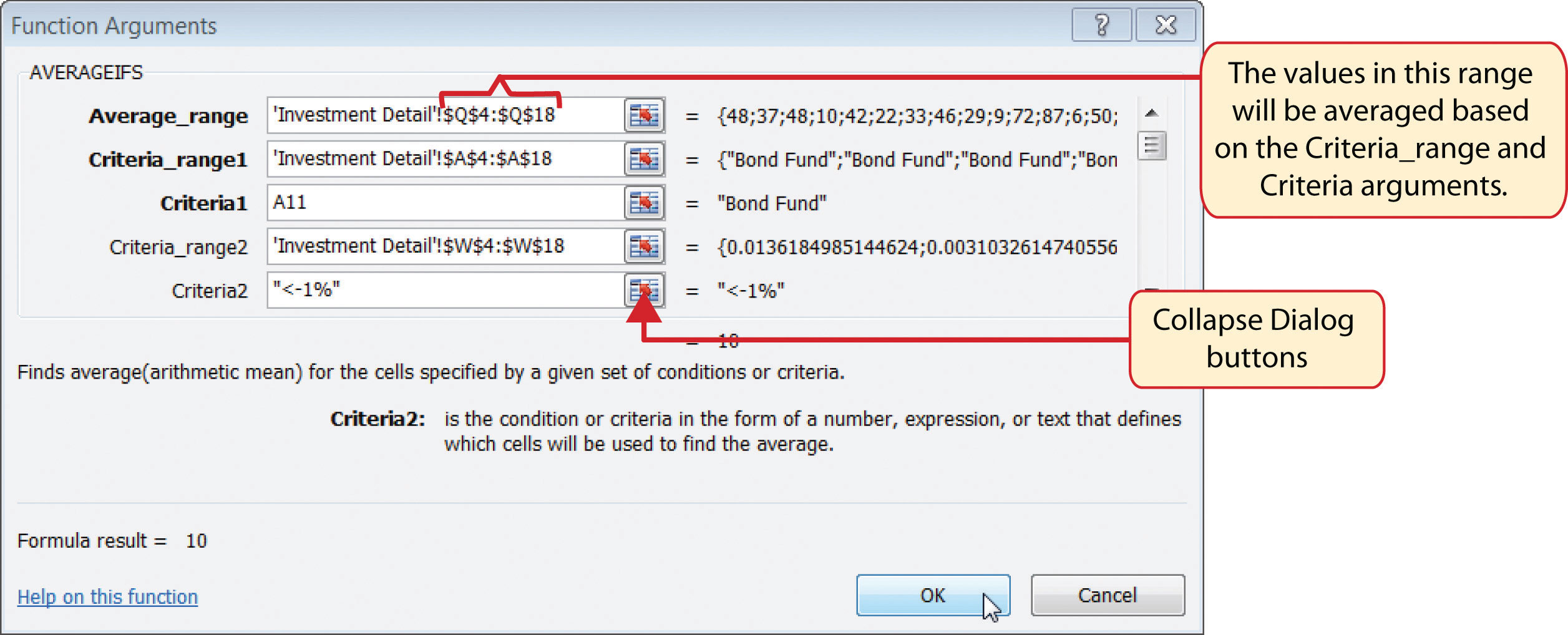23. Enter an AVERAGEIF function in cell C15 by typing an equal sign (=) followed by the function name AVERAGEIF followed by an open parenthesis (().
24. Click the Investment Detail worksheet tab and highlight the range W4:W18 to define the Range argument. Type a comma.
25. Type the following to define the criteria argument: “<–1%”. Type a comma.
26. Highlight the range Q4:Q18 to define the [Average_range] argument. Then type a closing parenthesis ()) and press the ENTER key on your keyboard.

Figure 3.36 "Results of the AVERAGEIFS Function" shows the results of the AVERAGEIFS function in the Portfolio Summary worksheet. The function shows the average months of ownership for the poor performing investments by investment type.

Figure 3.36 Results of the AVERAGEIFS Function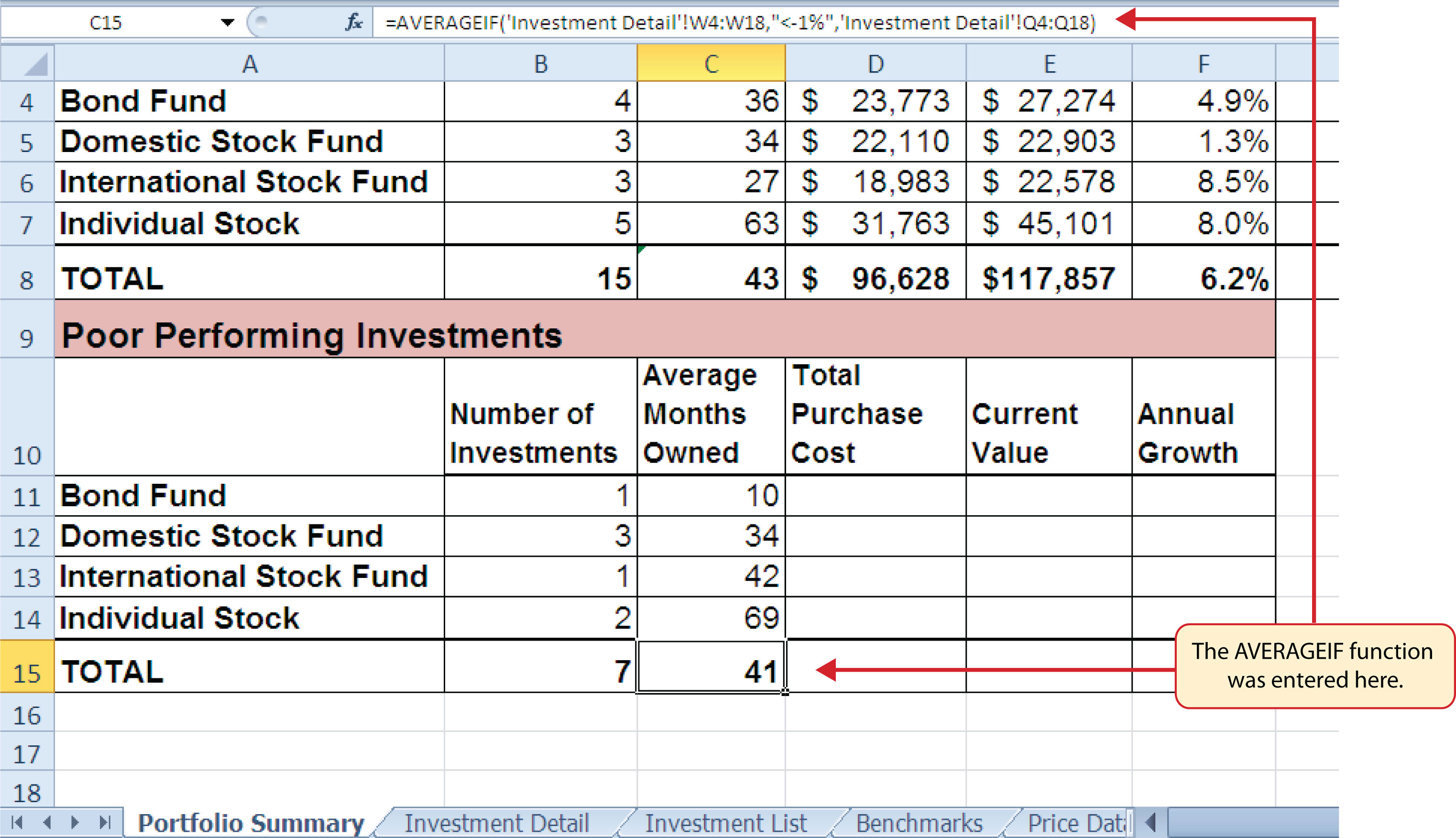### Skill Refresher: AVERAGEIFS Function

1. Type an equal sign (=).
2. Type the function name AVERAGEIFS followed by an open parenthesis (().
3. Define the Average_range argument with a range of cells that contain values to be averaged.
4. Type a comma.
5. Define the Criteria_range1 argument with a range of cells that will be evaluated based on the criteria used to define the Criteria1 argument.
6. Type a comma.
7. Define the Criteria1 argument with a cell location, number, text, or logical test. Text and logical tests must be enclosed in quotation marks.
8. Repeat steps 4, 5, 6, and 7 to define as many pairs of criteria arguments as needed.
9. Type a closing parenthesis ()).
10. Press the ENTER key on your keyboard.

## The SUMIFS Function

Follow-along file: Continue with Excel Objective 3.00. (Use file Excel Objective 3.13 if starting here.)

### Lesson Video: SUMIFS Function

The SUMIFS function is similar to the AVERAGEIFS function in that multiple criteria arguments can be defined to select cells from a targeted range (see Table 3.9 "Arguments for the AVERAGEIFS and SUMIFS Functions"). The function will use the selected cells from this targeted range to calculate a total or sum. The SUMIFS function will be used to complete the Total Purchase Cost and Current Value columns in the Poor Performing Investments section of the Portfolio Summary worksheet. The following steps explain how to construct this function for the Total Purchase Cost column:

1. Click cell D11 on the Portfolio Summary worksheet.
2. Click the Formulas tab on the Ribbon.
3. Click the Math & Trig button in the Function Library group of commands (see Figure 3.25 "Selecting the COUNTIF Function from the Function Library").
4. Select the SUMIFS function from the drop-down list. Use the scroll bar to scroll down to find the SUMIFS function. This will open the Function Arguments dialog box.
5. Click the Collapse Dialog button next to the Sum_range argument on the Function Arguments dialog box (see Figure 3.37 "Completed Arguments for the SUMIFS Function").
6. Click the Investment Detail worksheet tab.
7. Highlight the range G4:G18 on the Investment Detail worksheet and press the ENTER key on your keyboard. Selected cells from this range will be summed based on the defined criteria in ensuing arguments.
8. Click in the Sum_range argument input box and place an absolute reference on the range G4:G18. This is done by typing a dollar sign (\$) in front of the column letter and row number for both cells in the range.
9. Click the Collapse Dialog button next to the Criteria_range1 argument on the Function Arguments dialog box (see Figure 3.37 "Completed Arguments for the SUMIFS Function").
10. Click the Investment Detail worksheet tab.
11. Highlight the range A4:A18 on the Investment Detail worksheet and press the ENTER key on your keyboard. Once the Criteria_range1 argument is defined, the Criteria1 argument will appear in the Function Arguments dialog box.
12. Click in the Criteria_range1 argument input box and place an absolute reference on the range A4:A18. This is done by typing a dollar sign (\$) in front of the column letter and row number for both cells in the range.
13. Press the TAB key on your keyboard to advance to the Criteria1 argument and type the cell location A11. The criteria for the function will be the investment type that is entered into cell A11 on the Portfolio Summary worksheet. You will notice that as soon as you define this argument, the Criteria_range2 argument will appear on the Function Arguments dialog box.
14. Click the Collapse Dialog button next to the Criteria_range2 argument on the Function Arguments dialog box (see Figure 3.37 "Completed Arguments for the SUMIFS Function").
15. Click the Investment Detail worksheet tab.
16. Highlight the range W4:W18 on the Investment Detail worksheet and press the ENTER key on your keyboard.
17. Click in the Criteria_range2 argument input box and place an absolute reference on the range W4:W18. This is done by typing a dollar sign (\$) in front of the column letter and row number for both cells in the range.
18. Press the TAB key on your keyboard to advance to the Criteria2 argument.
19. Type an open quotation mark followed by the logical test <−1% and then type a closing quotation mark (“<−1%”). The second criterion for this function is a logical test that will identify cell locations where the value is less than −1%. For the purposes of this exercise, a poor performing investment is one that is below the target growth rate by more than 1%.
20. Click the OK button at the bottom of the Function Arguments dialog box.
21. Copy the function in cell D11 and paste it into the range D12:D14 using the Paste Formulas command.
22. Enter a regular SUM function in cell D15 on the Portfolio Summary worksheet that sums the values in the range D11:D14.

Figure 3.37 "Completed Arguments for the SUMIFS Function" shows the defined arguments for the SUMIFS function. Values that meet all criteria defined in the function will be selected from the range G4:G18. Notice that the results for each argument of the function are shown along the right side of the Collapse Dialog buttons.

Figure 3.37 Completed Arguments for the SUMIFS Function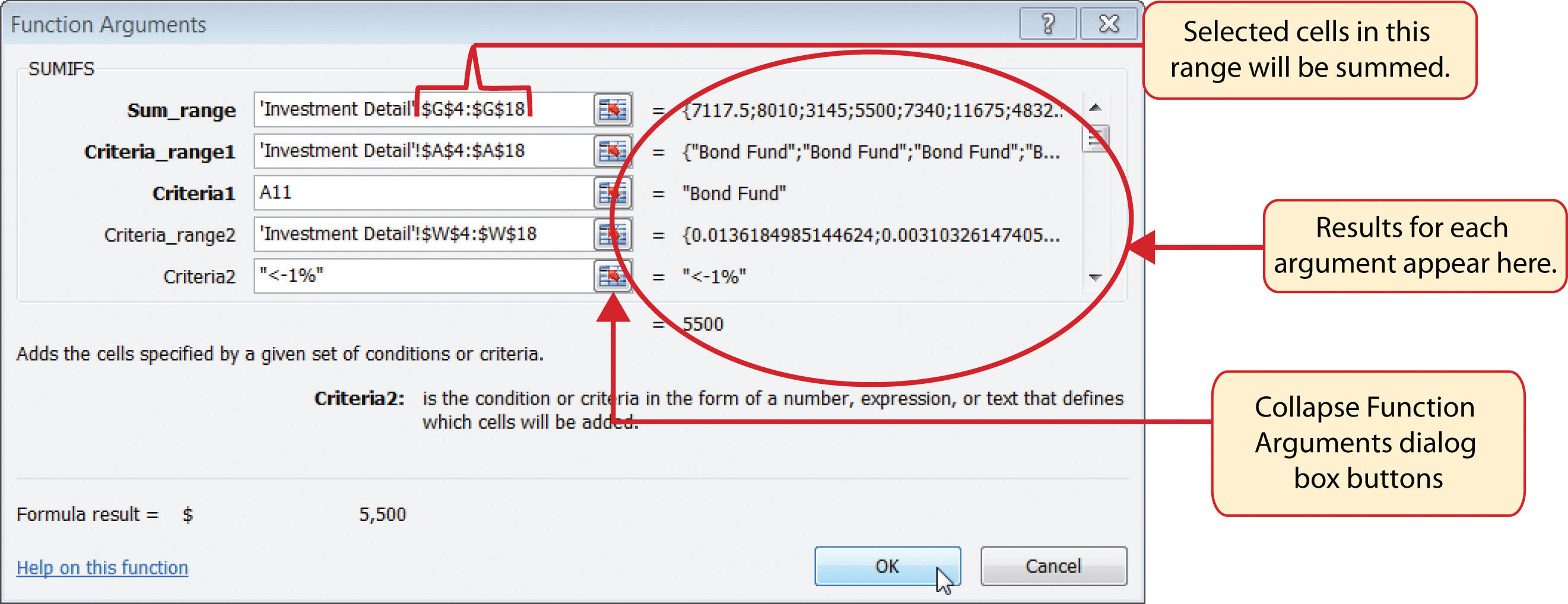Figure 3.38 "SUMIFS Function Output for the Total Purchase Cost Column" shows the results of the SUMIFS function used to complete the Total Purchase Cost column. In total, over \$45,000 was invested in funds and stocks that are not meeting the performance goals of the portfolio.

Figure 3.38 SUMIFS Function Output for the Total Purchase Cost Column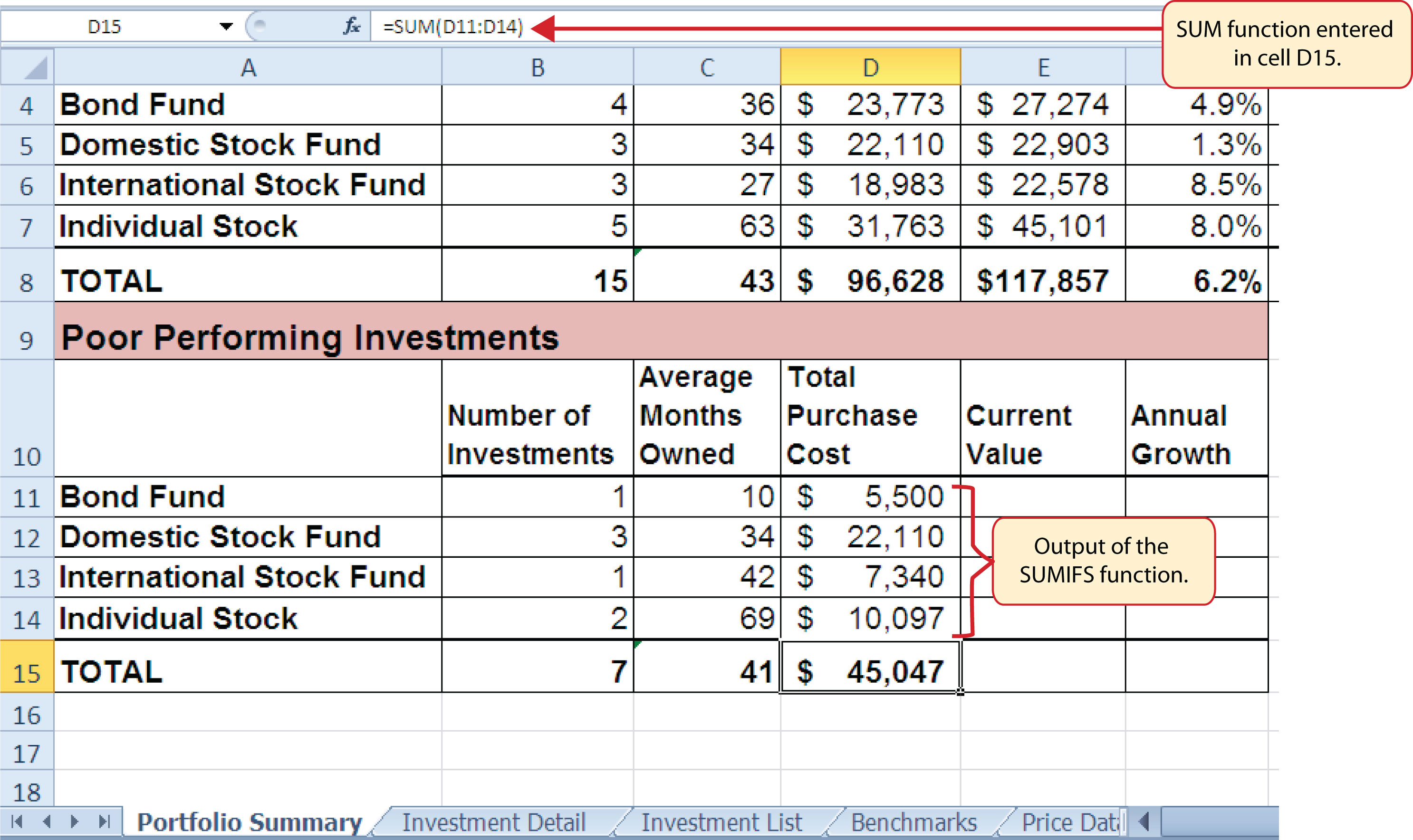In addition to the Total Purchase Cost column, the SUMIFS function will also be used to complete the Current Value column for the Poor Performing Investments section on the Portfolio Summary worksheet. The following steps explain how to add the function to the worksheet to complete this column:

1. Click cell E11 on the Portfolio Summary worksheet.
2. Click the Formulas tab on the Ribbon.
3. Click the Math & Trig button in the Function Library group of commands (see Figure 3.25 "Selecting the COUNTIF Function from the Function Library").
4. Select the SUMIFS function from the drop-down list. Use the scroll bar to scroll down to find the SUMIFS function. This will open the Function Arguments dialog box.
5. Click the Collapse Dialog button next to the Sum_range argument on the Function Arguments dialog box (see Figure 3.39 "Completed Function Arguments Dialog Box for the Second SUMIFS Function").
6. Click the Investment Detail worksheet tab.
7. Highlight the range K4:K18 on the Investment Detail worksheet and press the ENTER key on your keyboard. Selected cells from this range will be summed based on the defined criteria in ensuing arguments.
8. Click in the Sum_range argument input box and place an absolute reference on the range K4:K18. This is done by typing a dollar sign (\$) in front of the column letter and row number for both cells in the range.
9. Click the Collapse Dialog button next to the Criteria_range1 argument on the Function Arguments dialog box (see Figure 3.39 "Completed Function Arguments Dialog Box for the Second SUMIFS Function").
10. Click the Investment Detail worksheet tab.
11. Highlight the range A4:A18 on the Investment Detail worksheet and press the ENTER key on your keyboard. Once the Criteria_range1 argument is defined, the Criteria1 argument will appear in the Function Arguments dialog box.
12. Click in the Criteria_range1 argument input box and place an absolute reference on the range A4:A18. This is done by typing a dollar sign (\$) in front of the column letter and row number for both cells in the range.
13. Press the TAB key on your keyboard to advance to the Criteria1 argument and type the cell location A11. The criteria for the function will be the investment type entered into cell A11 on the Portfolio Summary worksheet.
14. Click the Collapse Dialog button next to the Criteria_range2 argument on the Function Arguments dialog box (see Figure 3.39 "Completed Function Arguments Dialog Box for the Second SUMIFS Function").
15. Click the Investment Detail worksheet tab.
16. Highlight the range W4:W18 on the Investment Detail worksheet and press the ENTER key on your keyboard.
17. Click in the Criteria_range2 argument input box and place an absolute reference on the range W4:W18. This is done by typing a dollar sign (\$) in front of the column letter and row number for both cells in the range.
18. Press the TAB key on your keyboard to advance to the Criteria2 argument.
19. Type an open quotation mark followed by the logical test <−1% and then type a closing quotation mark (“<−1%”). The second criterion for this function is a logical test that will identify cell locations where the value is less than −1%. For the purposes of this exercise, a poor performing investment is one that is below the target growth rate by more than 1%.
20. Click the OK button at the bottom of the Function Arguments dialog box.
21. Copy the function in cell E11 and paste it into the range E12:E14 using the Paste Formulas command.
22. Enter a regular SUM function in cell E15 on the Portfolio Summary worksheet that sums the values in the range E11:E14 (see Figure 3.40 "SUMIFS Function Output for the Current Value Column").

Figure 3.39 Completed Function Arguments Dialog Box for the Second SUMIFS Function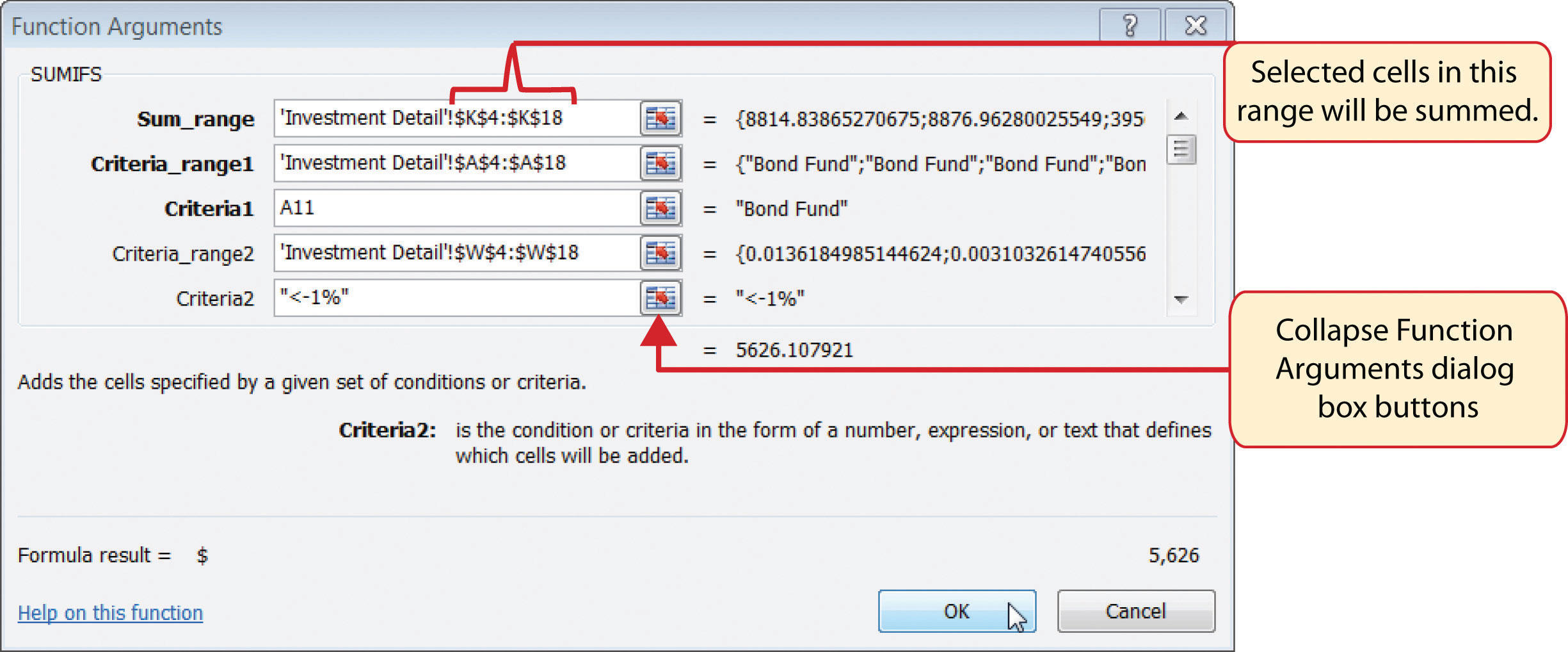Figure 3.40 SUMIFS Function Output for the Current Value Column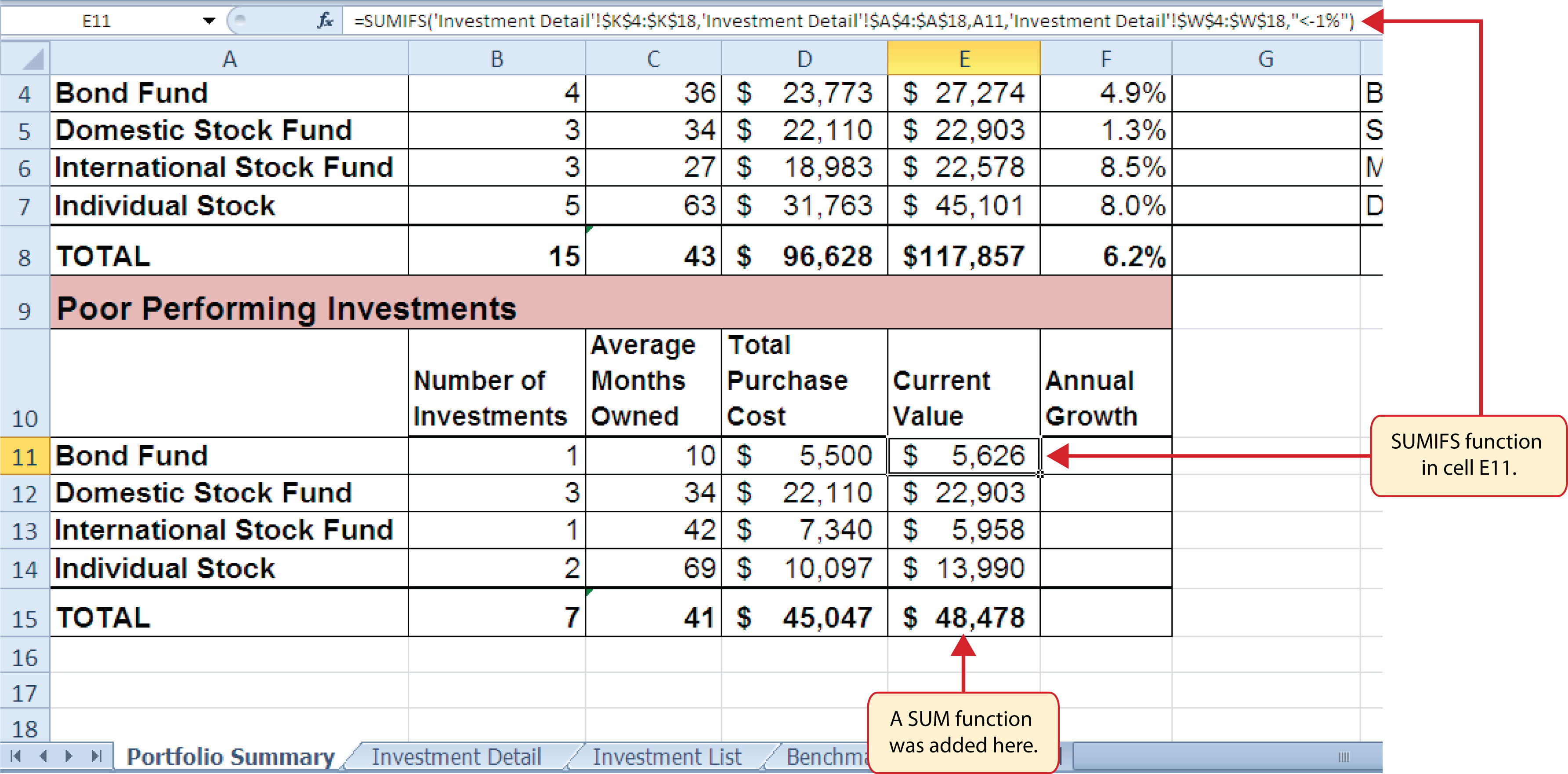Now that the Total Purchase Cost and Current Value columns are completed for the Poor Performing Investments section on the Portfolio Summary worksheet, we can add a formula to show the annual growth rate by investment type. Since this is the same formula that was used in the Total Summary section of the Portfolio Summary worksheet, we can just copy and paste it. The following steps explain how to do this:

1. Copy the formula in cell F4 on the Portfolio Summary worksheet.
2. Highlight the range F11:F15 on the Portfolio Summary worksheet.
3. Paste the formula using the Paste Formulas command.

### Integrity Check

Error Message for Statistical IF Functions

If you receive an error message when attempting to enter a statistical IF function into a cell location, check to make sure any criteria using a comparison operator is enclosed in quotation marks. For example, if you are assessing a range of cells to see if the values are greater than or equal to zero, you must define the Criteria argument as follows: “>=0”. If you do not use the quotation marks, Excel will not accept the function in the cell location and will display an error message.

Figure 3.41 "Completed Poor Performing Investments Section of the Portfolio Summary Worksheet" shows the completed Poor Performing Investments section on the Portfolio Summary worksheet. Notice that even though an investment is considered “poor performing” it does not mean that money is lost on the investment. As shown in the figure, only one investment in the International Stock Fund category is losing money, with an annual growth rate of −5.4%. However, the total annual growth rate for all investments in the Poor Performing section is 2.2% (see cell F15). This is less than half the growth rate for the overall portfolio, which is 6.2% (see cell F8). A final observation is that all the investments in the Domestic Stock Fund category are “poor performing.” The same number of investments in the Total Summary section appears in the Poor Performing Investments section. This completes the Portfolio Summary worksheet with the exception of one column. We will complete this column in the next section with a lookup function.

Figure 3.41 Completed Poor Performing Investments Section of the Portfolio Summary Worksheet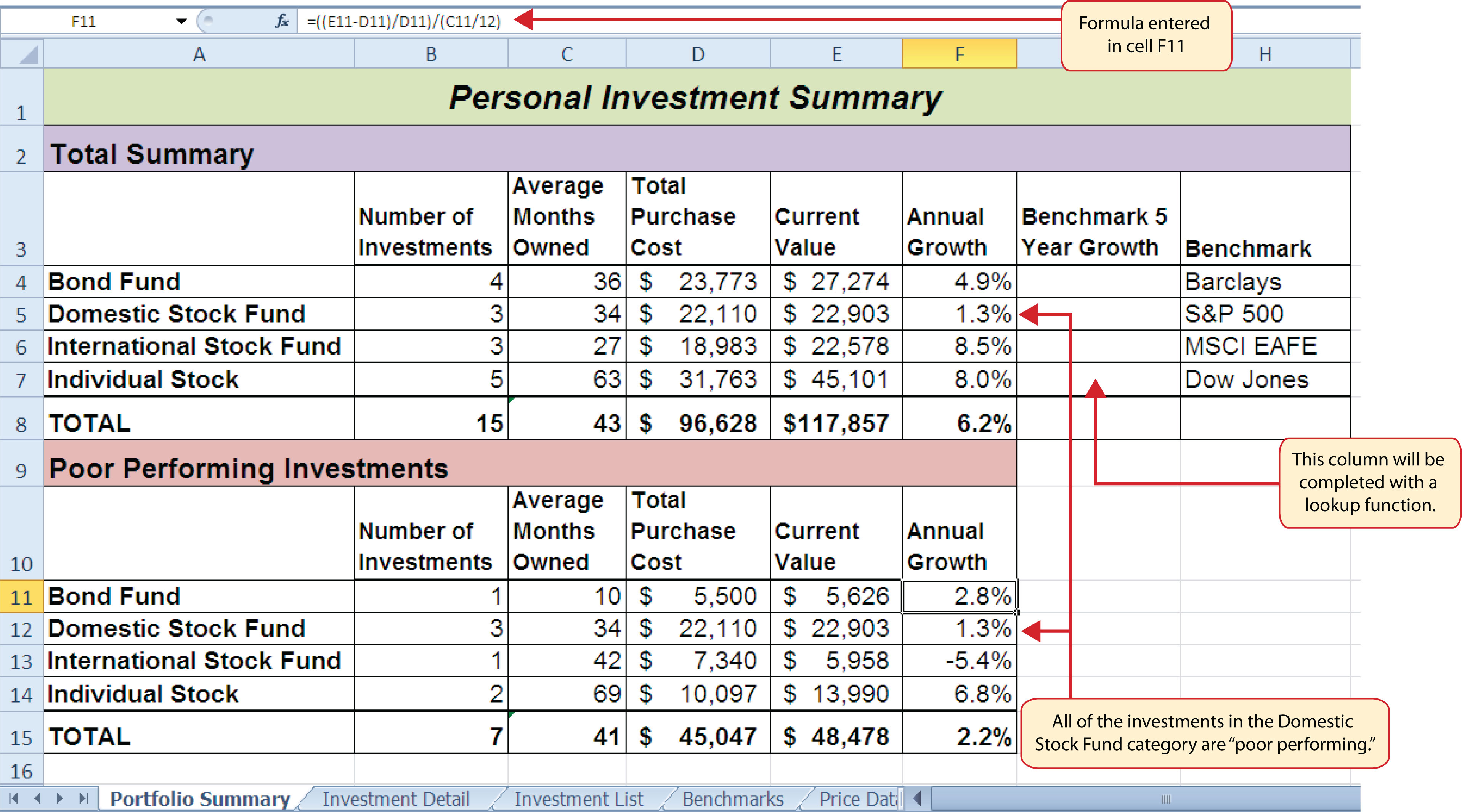### Skill Refresher: SUMIFS Function

1. Type an equal sign (=).
2. Type the function name SUMIFS followed by an open parenthesis (().
3. Define the Sum_range argument with a range of cells that contain values to be summed.
4. Type a comma.
5. Define the Criteria_range1 argument with a range of cells that will be evaluated based on the criteria used to define the Criteria1 argument.
6. Type a comma.
7. Define the Criteria1 argument with a cell location, number, text, or logical test. Text and logical tests must be enclosed in quotation marks.
8. Repeat steps 4, 5, 6, and 7 to define as many pairs of criteria arguments as needed.
9. Type a closing parenthesis ()).
10. Press the ENTER key on your keyboard.

### Key Takeaways

• The COUNTIF, SUMIF, and AVERAGEIF functions can select specific cell locations from a range to compute an output using one criteria argument.
• The COUNTIFS, SUMIFS, and AVERAGEIFS functions can select specific cell locations from a range to compute an output using multiple sets of criteria arguments.
• When using the AVERAGEIF and SUMIF functions, the row numbers used in the ranges to define the Range argument and the Average_range or Sum_range arguments must be identical.
• The benefit of using statistical functions when creating a summary worksheet is that if rows of data are added to the detail worksheet, the new data will automatically be included in the function output.
• When using statistical IF functions, the logical test used to define a criteria argument must be enclosed in quotation marks.

### Exercises

1. Consider the following SUMIF function: =SUMIF(B2:B10,A1,E2:E20). What cell range will be used to compute the output for the function?

1. E2:E10
2. B2:B10
3. E2:E20
4. B2:B20
2. Which of the following is true with respect to the COUNTIF function?

1. It can only be used to sum selected cells from a range using one set of defined criteria.
2. It can be used to count both numeric and nonnumeric values in selected cells from a range using one set of defined criteria.
3. It can be used to count only numeric values in selected cells from a range given one set of defined criteria.
4. It can be used to count only numeric values in selected cells from a range using multiple sets of defined criteria.
3. If you wanted to calculate the average of the values contained in the range B5:B20 based on any values in the range E5:E20 with a value greater than 5, which of the following functions would provide an accurate result?

1. =COUNTIF(B5:B20,>5,E5:E20)
2. =AVERAGEIF(B5:B20,>5,E5:E20)
3. =AVERAGEIF(E5:E20,>5,B5:B20)
4. =AVERAGEIF(E5:E20,”>5”,B5:B20)
4. Suppose you wanted to sum the values in the range C3:C15 where the values in B3:B15 are equal to the word Bond and where the values in the range D3:D15 are greater than 3%. Which of the following functions would provide an accurate result?

1. =SUMIFS(C3:C15,B3:B15,“Bond”,D3:D15,“>3%”)
2. =SUMIFS(B3:B15,“Bond”,D3:D15,“>.03”,C3:C15)
3. =SUMIFS(\$C\$3:\$C\$15,\$B\$3:\$B\$15,“Bond”,\$D\$3:\$D\$15,>3%)
4. =SUMIFS(\$B\$3:\$B\$15,=Bond,\$D\$3:\$D\$15,>.03,\$C\$3:\$C\$1)

## 3.3 Lookup Functions

### Learning Objectives

1. Use the VLOOKUP function to search and display the contents of a cell location for data that is organized in columns.
2. Use the HLOOKUP function to search and display the contents of a cell location for data that is organized in rows.
3. Create a web query that imports stock price data into a worksheet from a website.

The final section of this chapter addresses lookup functions. Lookup functionsFunctions that are used to search for and display data located in an existing worksheet or in other worksheets or workbooks. are typically used to search for and display data located in other worksheets or workbooks. The two lookup functions we will use in our example of the personal investment portfolio are the VLOOKUP and HLOOKUP functions. In addition to demonstrating these functions, we will also show how we can enhance the personal investment portfolio workbook with a web query. Web queries are used to bring live or current data into a worksheet from a website.

## The VLOOKUP Function

Follow-along file: Continue with Excel Objective 3.00. (Use file Excel Objective 3.14 if starting here.)

### Lesson Video: VLOOKUP Function

The VLOOKUP function is typically used to access and display data located in another worksheet or workbook. The function can also be used to access and display data located in the same worksheet. This is a very powerful and versatile function because it eliminates the need to copy or recreate data that exists in other worksheets or workbooks. It is called a VLOOKUP function because the function will search vertically down the first column of a range of cells to find what is called a lookup value. This process is very similar to the statistical IF functions in Section 3.2 "Statistical IF Functions". You will recall that these functions used criteria to select cells from a range that was used in the mathematical output. The VLOOKUP function is essentially performing the same process; however, instead of selecting multiple cells from a range, the function is only looking for one specific cell location. Once the function finds the specific cell location, it will display the contents of that cell location or another cell location in the range. Before using the VLOOKUP function in the personal investment portfolio workbook, it is strongly recommended that you carefully read the definitions for the function arguments listed in Table 3.10 "Arguments for the VLOOKUP Function".

Table 3.10 Arguments for the VLOOKUP Function

Argument Definition
Lookup_value This argument is typically defined with a cell location, number, or text. Text data must be enclosed in quotation marks for this argument. The function will search for the criteria entered into this argument in the first column of the range used to define the Table_array argument. For example, if the word Hat is used to define this argument, the function will search for the word Hat in the first column of the range used to define the Table_array argument.
Table_array Range of cells that contain data you wish the VLOOKUP function to search though (Lookup_value) and display. This cell range must contain the criteria used to define the Lookup_value in the first column. For example, if the range A2:D15 is used to define this argument, the criteria used to define the Lookup_value argument must exist in Column A.
Col_index_num This is the column index number argument. It is defined with the number of columns to the right of the first column in the range used to define the Table_array argument that contains the data you wish to display. For example, suppose the data you wish the function to display is contained in Column C. If the range used to define the Table_array argument is A2:D15, then the column index number will be 3. Counting the columns to the right of the first column in this range, Column A would be 1, Column B would be 2, and Column C would be 3. It is important to remember to count the first column in the table array range as 1.
[Range_lookup] This argument is defined with either the word TRUE or the word FALSE. When this argument is defined with the word FALSE, the function will look for an exact match to the criteria used to define the Lookup_value argument in the first column of the table array range. It is important to note the function will search the entire range to find a match. If this argument is defined with the word TRUE, the function will look for a value that is an exact match or the closest match that is less than the lookup value. For example, if the lookup value is 80 and the highest value in the first column of the table array range is a 78, the function will consider 78 a match for the number 80. However, if the lookup value is 80 and the lowest number in the first column of the table array range is 85, the function will produce an error. This is because the number 80 and any value less than 80 do not exist in the first column of the table array range. It is important to note that if you define this argument with the word TRUE, the data in the table array range must be sorted in ascending order. This is because the function will stop searching for a match once the value in the first column exceeds the lookup value. If the data in the table array range is not sorted, the function can either produce an error code or display an erroneous result. This argument is in brackets because if it is not defined it will automatically be defined with the word TRUE.

### Integrity Check

Using a TRUE Range Lookup for VLOOKUP and HLOOKUP

If you are defining the Range_lookup argument with the word TRUE for either the VLOOKUP or HLOOKUP function, the range used to define the Table_array argument must be sorted in ascending order. For the VLOOKUP function, the table array range must be sorted from smallest to largest or from A to Z based on the values in the first column. For the HLOOKUP function, the table array range must be sorted from left to right based on the values in the first row, from smallest to largest or A to Z.

You may have noticed that on the Investment Detail worksheet, the Description column is blank (see Figure 3.2 "Investment Detail Worksheet"). Descriptions for several investments are included in the workbook in the Investment List worksheet as shown in Figure 3.42 "Investment List Worksheet". The VLOOKUP function will be used to search for a specific symbol in Column A of the Investment List worksheet and display the description for that symbol located in Column B. The following steps explain how to accomplish this:

Figure 3.42 Investment List Worksheet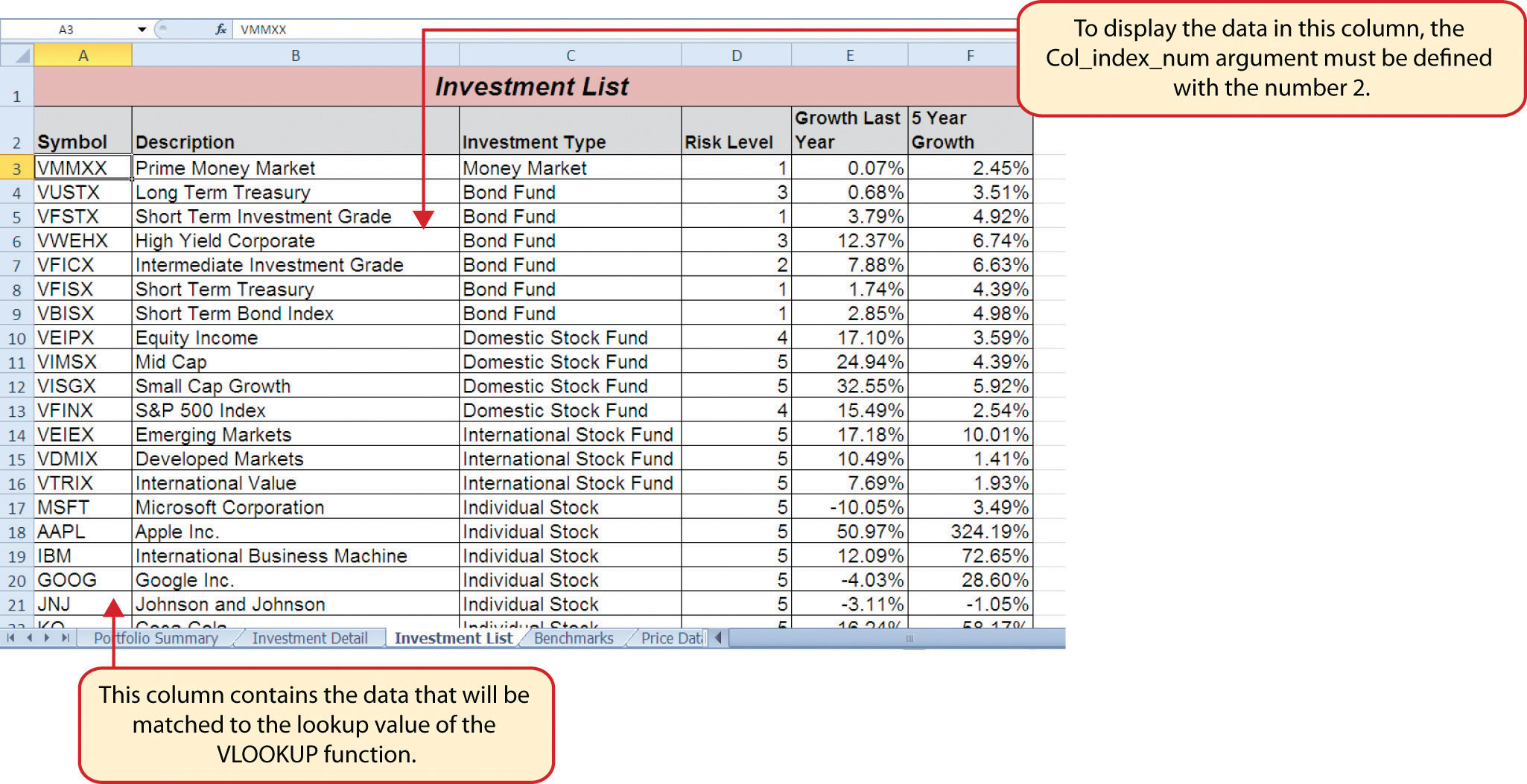1. Click cell C4 on the Investment Detail worksheet.
2. Click the Formulas tab on the Ribbon.
3. Click the Lookup & Reference button in the Function Library group of commands.
4. Select the VLOOKUP function from the list of functions. Use the scroll bar to scroll down to the bottom of the list. This will open the Function Arguments dialog box for the VLOOKUP function.
5. Click the Collapse Dialog button next to the Lookup_value argument on the Function Arguments dialog box.
6. Click cell B4 and press the ENTER key on your keyboard. The symbol in cell B4 is the lookup value that will be searched in the first column of the range defined for the Table_array argument.
7. Click the Collapse Dialog button next to the Table_array argument on the Function Arguments dialog box.
8. Click the Investment List worksheet tab.
9. Highlight the range A3:F23 on the Investment List worksheet and press the ENTER key on your keyboard. The function will look in Column A of this range for the lookup value.
10. Click in the input box for the Table_array argument and place an absolute reference on the range A3:F23. This is done by typing a dollar sign (\$) in front of the column letter and row number of each cell location in the range.
11. Press the TAB key on your keyboard to advance to the Col_index_num argument and type the number 2. Once the function finds the lookup value in Column A of the range A3:F23, it will display the description that is in Column B of the same row.
12. Press the TAB key on your keyboard to advance to the Range_lookup argument and type the word FALSE. This will direct the function to search for only exact matches to lookup value.
13. Click the OK button at the bottom of the Function Arguments dialog box.
14. Copy the VLOOKUP function in cell C4 and paste it into the range C5:C18 using the Paste Formulas command.

Figure 3.43 "Completed Function Arguments Dialog Box for the VLOOKUP Function" shows the completed Function Arguments dialog box for the VLOOKUP function. Notice that the Range_lookup argument is defined with the word FALSE. This will direct the function to search for an exact match to the lookup value and will also direct the function to search the entire first column of the table array range. Finally, it is important to note the absolute reference on the table array range. This will prevent the table array range from changing when the function is pasted into other cell locations.

Figure 3.43 Completed Function Arguments Dialog Box for the VLOOKUP Function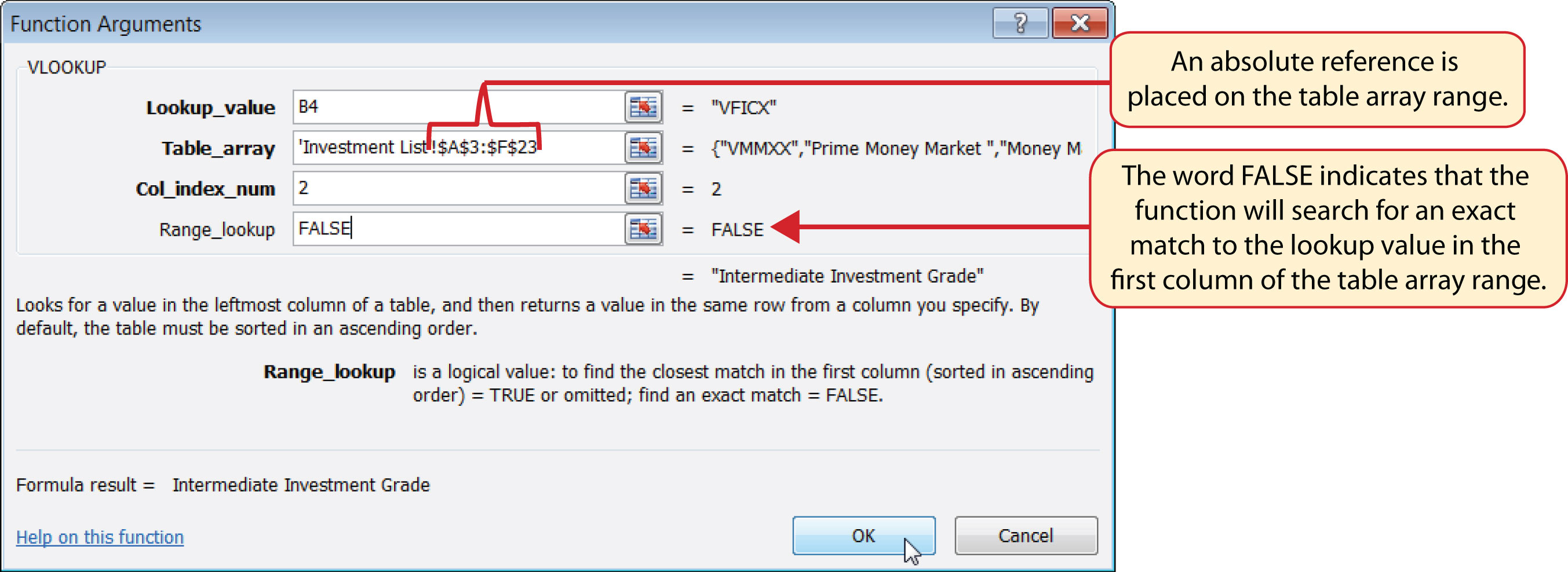Figure 3.44 "Results of the VLOOKUP Function in the Investment Detail Worksheet" shows the results of the VLOOKUP function in the Investment Detail worksheet. The function is searching for each symbol in Column B of the Investment Detail worksheet in Column A of the Investment List worksheet. When the function finds a match, it will display whatever is in the cell location two columns to the right, or Column B, in the Investment List worksheet. For example, the symbol VDMIX, which is in cell B8 on the Investment Detail worksheet (see Figure 3.44 "Results of the VLOOKUP Function in the Investment Detail Worksheet"), is also in cell A15 on the Investment List worksheet (see Figure 3.42 "Investment List Worksheet"). As a result, the function is displaying whatever is in cell B15 on the Investment List worksheet, which is the description “Developed Markets.”

Figure 3.44 Results of the VLOOKUP Function in the Investment Detail Worksheet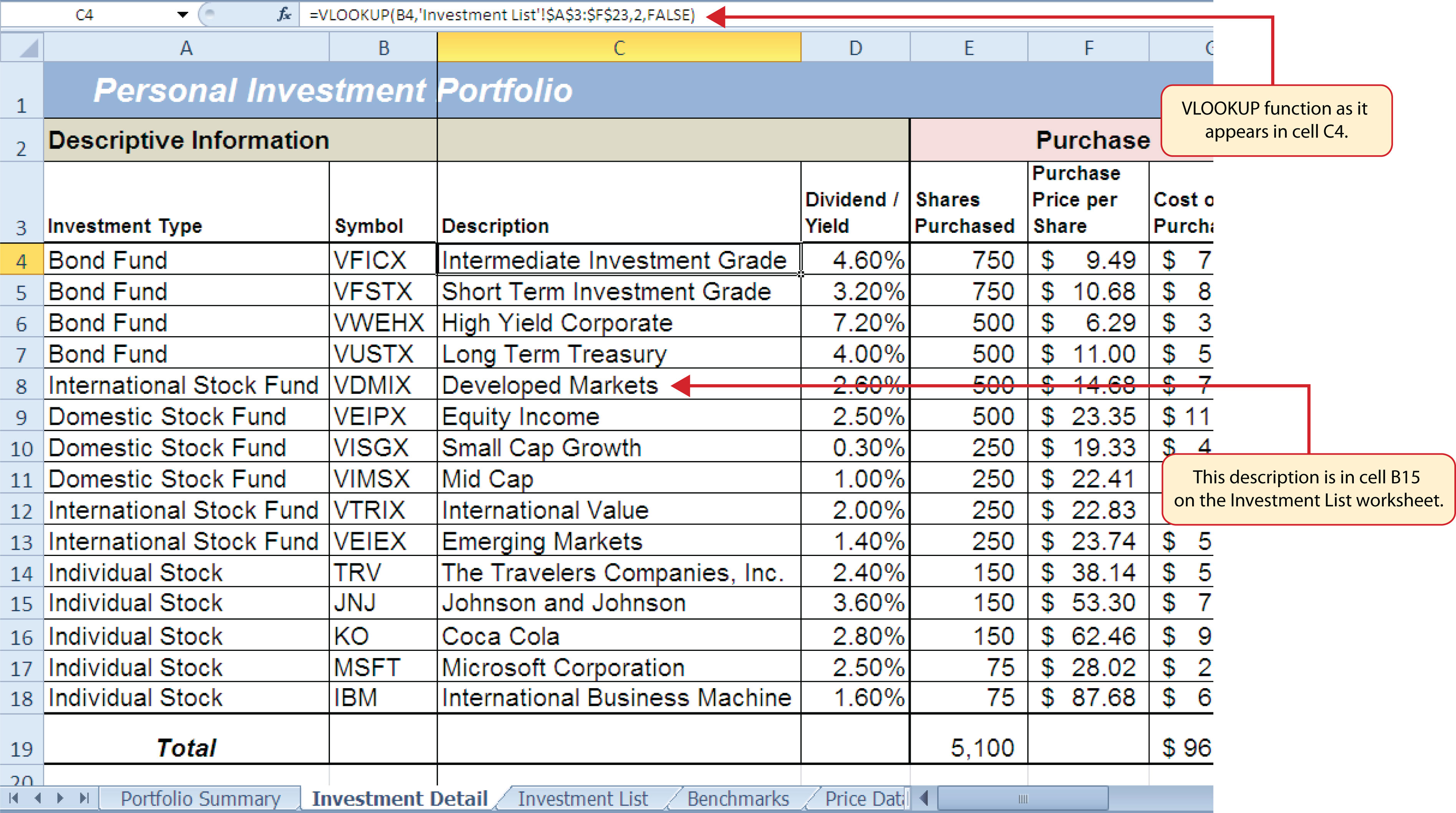### Integrity Check

Absolute References on the Table Array Range for the VLOOKUP and HLOOKUP Functions

If you are copying and pasting a VLOOKUP or HLOOKUP function, you will most likely need to place an absolute reference on the range used to define the Table_array argument. The table array range will change because of relative referencing once the function is pasted to new cell locations. This may result in an error output for either the VLOOKUP or HLOOKUP function. This is because the function will not be able to find the lookup value since the range has been adjusted. If you are defining the Range_lookup argument with the word TRUE, an adjustment in the table array range may result in an erroneous output.

### Skill Refresher: VLOOKUP Function

1. Type an equal sign (=).
2. Type the function name VLOOKUP followed by an open parenthesis (().
3. Define the Lookup_value argument with a cell location, number, or text that will be searched in another workbook or worksheet. Text must be placed in quotation marks.
4. Type a comma.
5. Define the Table_array argument with a range of cells that contain the lookup value in the first column along with data that is to be displayed or used by the function.
6. Type a comma.
7. Define the Col_index_num argument with a number that designates the columns to the right in the table array range that will be displayed by the function. Count the first column of the table array range as 1.
8. Type a comma.
9. Define the Range_lookup argument with either the word FALSE or the word TRUE. The word FALSE will search for exact matches to the lookup value. The word TRUE will search for an exact or closest match less than the lookup value. Excel will define this argument as TRUE if it is omitted.
10. Type a closing parenthesis ()).
11. Press the ENTER key on your keyboard.

## The HLOOKUP Function

Follow-along file: Continue with Excel Objective 3.00. (Use file Excel Objective 3.15 if starting here.)

### Lesson Video: HLOOKUP Function

The HLOOKUP function serves the same purpose as the VLOOKUP function. The HLOOKUP function can be used to display data from another worksheet or workbook. However, instead of searching for the lookup value vertically down the first column of the table array range, the HLOOKUP function searches horizontally across the first row of the table array range. When the function finds a match for the lookup value, it will display the contents in a cell location based on a row index number. This number designates how many rows below the first row of the table array range the function should display. Table 3.11 "Arguments for the HLOOKUP Function" provides a definition for each argument of the HLOOKUP function. It is best to review the definitions of these arguments carefully before using the function.

Table 3.11 Arguments for the HLOOKUP Function

Argument Definition
Lookup_value This argument is typically defined with a cell location, number, or text. Text data must be enclosed in quotation marks for this argument. The function will search for the criteria entered into this argument in the first row of the range used to define the Table_array argument. For example, if the word Hat is used to define this argument, the function will search for the word Hat in the first row of the range used to define the Table_array argument.
Table_array Range of cells that contain data you wish the HLOOKUP function to search though (Lookup_value) and display. This cell range must contain the criteria used to define the Lookup_value in the first row. For example, if the range A2:D15 is used to define this argument, the criteria used to define the Lookup_value argument must exist in Row 2.
Row_index_num This is the row index number argument. It is defined with the number of rows below the first row in the range used to define the Table_array argument that contains the data you wish to display. For example, suppose the data you wish the function to display is contained in Row 5. If the range used to define the Table_array argument is A2:D15, then the column index number will be 4. Counting the rows below the first row in this range, Row 2 would be 1, Row 3 would be 2, Row 4 would be 3, and Row 5 would be 4. It is important to remember to count the first row in the table array range as 1.
[Range_lookup] This argument is defined with either the word TRUE or the word FALSE. When this argument is defined with the word FALSE, the function will look for an exact match to the criteria used to define the Lookup_value argument in the first row of the table array range. It is important to note the function will search the entire range to find a match. If this argument is defined with the word TRUE, the function will look for a value that is an exact match or the closest match that is less than the lookup value. For example, if the lookup value is 80 and the highest value in the first row of the table array range is a 78, the function will consider 78 a match for the number 80. However, if the lookup value is 80 and the lowest number in the first row of the table array range is 85, the function will produce an error. This is because the number 80 and any value less than 80 do not exist in the first row of the table array range. It is important to note that if you define this argument with the word TRUE, the data in the table array range must be sorted based on the values in the first row in ascending order from left to right. This is because the function will stop searching for a match once the value in the first row exceeds the lookup value. If the data in the table array range is not sorted, the function can either produce an error code or display an erroneous result. This argument is in brackets because if it is not defined it will automatically be defined with the word TRUE.

The HLOOKUP function will be used on the Portfolio Summary worksheet to display the benchmark growth rates in the range G4:G7. A benchmarkA value or qualitative example that can be used as a standard point of comparison. Results that are higher or better than a benchmark are typically considered positive, whereas results that are lower or worse than the benchmark are typically considered negative. is a value that can be used as a standard point of comparison. The Benchmarks worksheet contains growth rates at different year intervals for the benchmarks that will be used to compare the performance for each investment type (see Figure 3.45 "Benchmarks Worksheet"). For the purposes of this workbook, we will be comparing the growth rates for each investment type to the 5-year average growth rate for the benchmarks categories listed in the range H4:H7. The following steps explain how to construct the HLOOKUP function to display the 5-year benchmark values in the Portfolio Summary worksheet:

Figure 3.45 Benchmarks Worksheet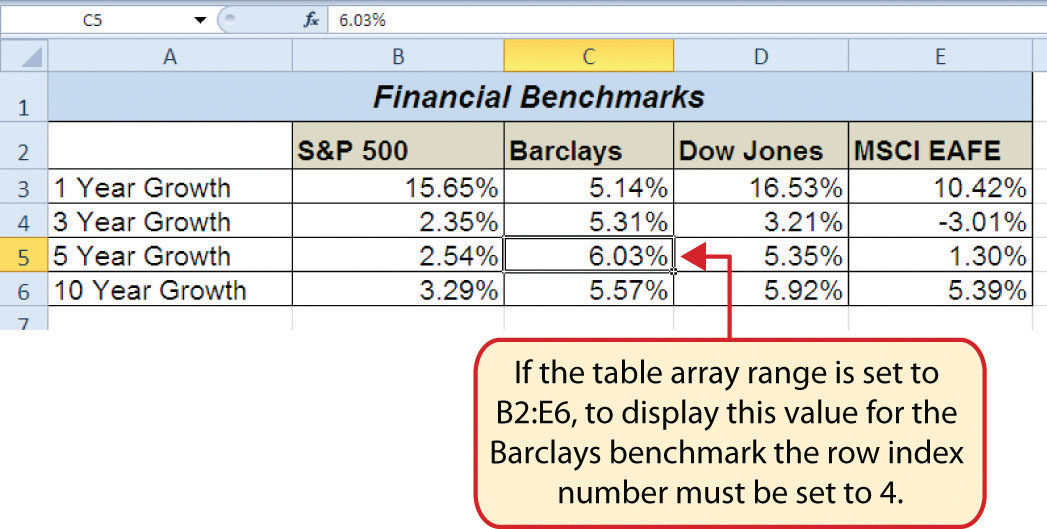1. Click cell G4 in the Portfolio Summary worksheet.
2. Click the Formulas tab on the Ribbon.
3. Click the Lookup & Reference button in the Function Library group of commands.
4. Select the HLOOKUP function from the list of functions. This will open the Function Arguments dialog box for the HLOOKUP function.
5. Click the Collapse Dialog button next to the Lookup_value argument on the Function Arguments dialog box.
6. Click cell H4 and press the ENTER key on your keyboard. The description in cell H4 will be the lookup value that will be searched in the first row of the range defined for the Table_array argument.
7. Click the Collapse Dialog button next to the Table_array argument on the Function Arguments dialog box.
8. Click the Benchmarks worksheet tab.
9. Highlight the range B2:E6 on the Benchmarks worksheet and press the ENTER key on your keyboard. The function will look in Row 2 of this range for the lookup value.
10. Click in the input box for the Table_array argument and place an absolute reference on the range B2:E6. This is done by typing a dollar sign (\$) in front of the column letter and row number of each cell location in the range.
11. Press the TAB key on your keyboard to advance to the Row_index_num argument and type the number 4. Once the function finds the lookup value in Row 2 of the range B2:E6, it will display the value that is in Row 5 of the same column. Remember that Row 2 is counted as Row 1 for the row index number in this example.
12. Press the TAB key on your keyboard to advance to the Range_lookup argument on the Function Arguments dialog box and type the word FALSE. This will direct the function to search for only exact matches of the lookup value.
13. Click the OK button at the bottom of the Function Arguments dialog box.
14. Copy the HLOOKUP function in cell G4 and paste it into the range G5:G7 using the Paste Formulas command.

Figure 3.46 "Completed Function Arguments Dialog Box for the HLOOKUP Function" shows the completed Function Arguments dialog box for the HLOOKUP function. The row index number 4 indicates that the function will display the contents of the cell location in the fourth row of the table array range.

Figure 3.46 Completed Function Arguments Dialog Box for the HLOOKUP Function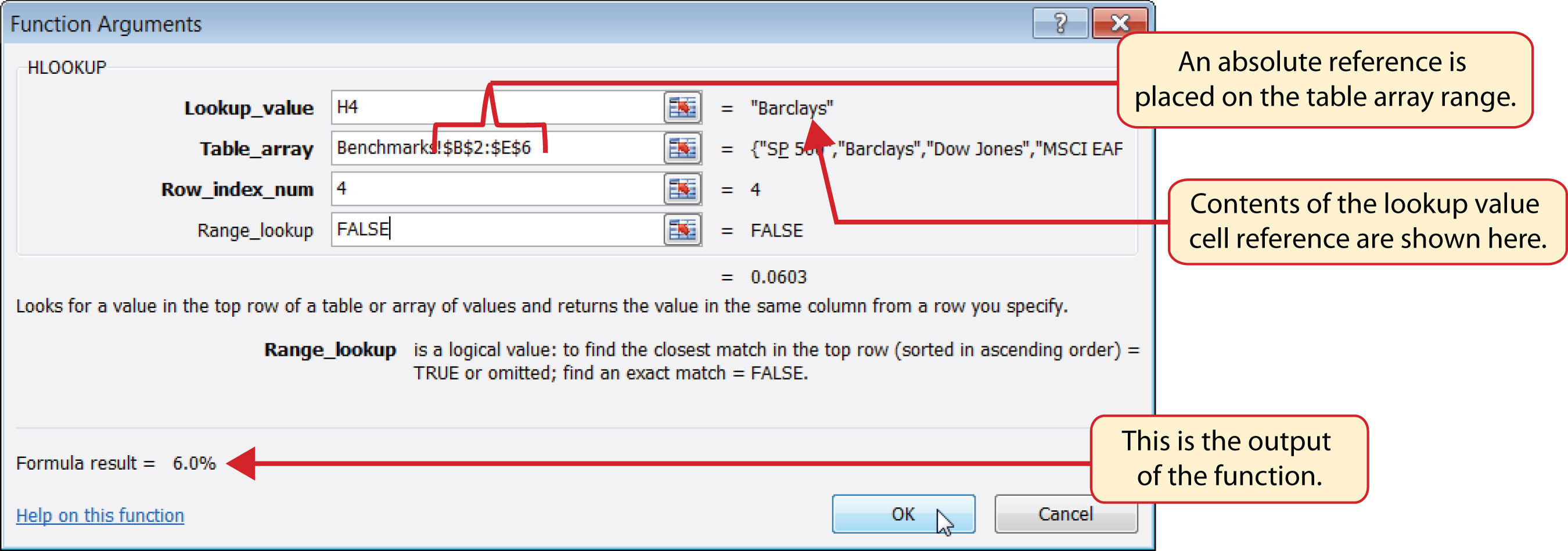Figure 3.47 "Completed Portfolio Summary Worksheet" shows the output of the HLOOKUP function. Notice that the output of the function in cell G4 is 6.0%. This is because the lookup value was defined with the entry in cell H4, which is the Barclays index. Looking at Figure 3.45 "Benchmarks Worksheet", if you count the first row of the table array range as Row 1, the value 6.03% is the fourth row in the Barclays column. Since the values in Column G on the Portfolio Summary worksheet are set to 1 decimal place, the value is displayed as 6.0%.

Figure 3.47 Completed Portfolio Summary Worksheet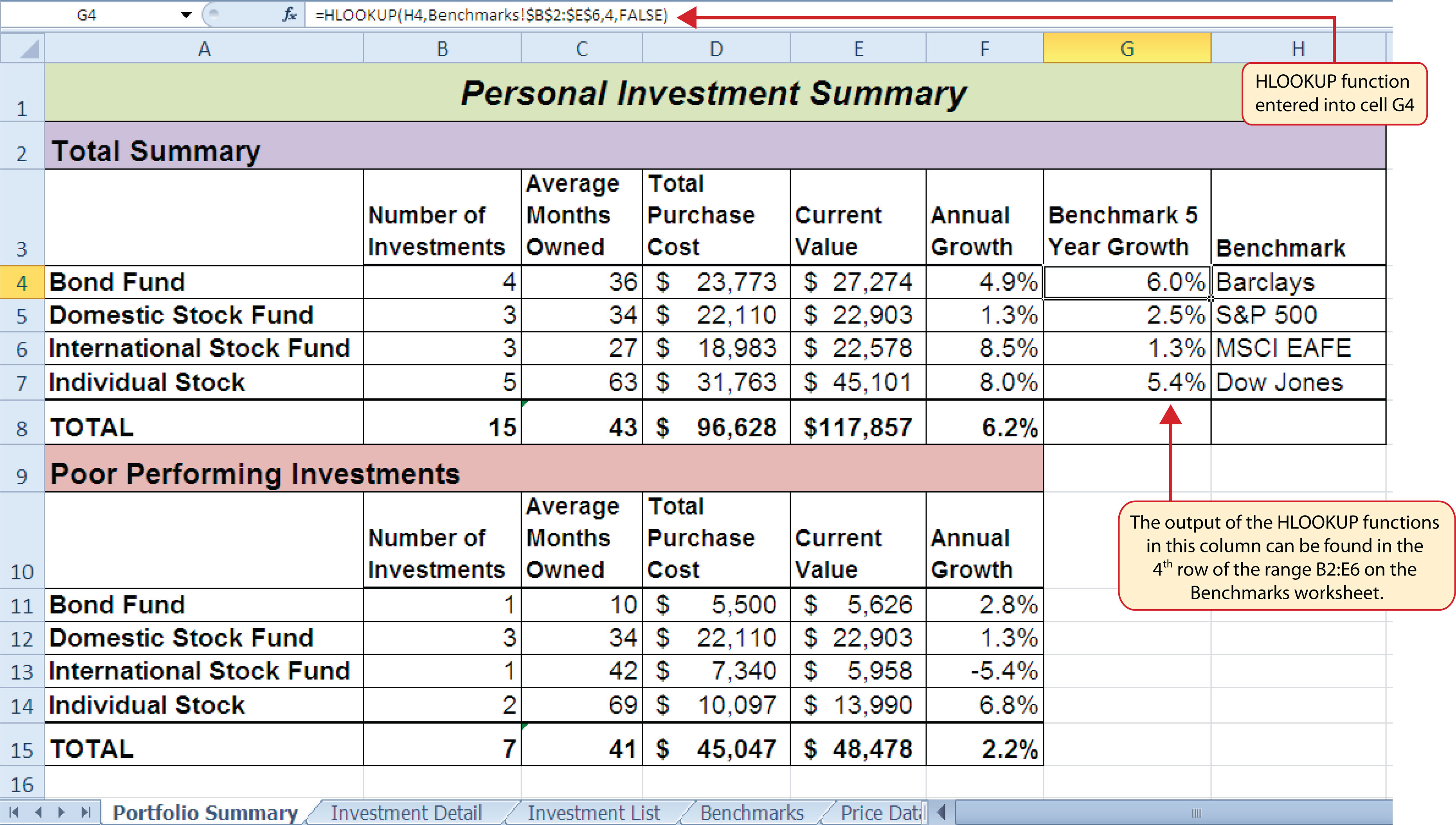### Integrity Check

#N/A and #REF! Errors with Lookup Functions

If you receive the #N/A error code when using the VLOOKUP or HLOOKUP function, it indicates that Excel cannot find the lookup value in the table array range. Check that the lookup value exists in the first column for the VLOOKUP, or the first row for the HLOOKUP, in the range used to define the Table_array argument. You may also see this error code if you copy and paste the function and forget to put an absolute reference on the range used to define the Table_array argument. The #REF! error code indicates that the column index number or row index number exceeds the number of columns or rows in the range used to define the Table_array argument.

### Skill Refresher: HLOOKUP Function

1. Type an equal sign (=).
2. Type the function name HLOOKUP followed by an open parenthesis (().
3. Define the Lookup_value argument with a cell location, number, or text that will be searched in another workbook or worksheet. Text must be placed in quotation marks.
4. Type a comma.
5. Define the Table_array argument with a range of cells that contain the lookup value in the first row along with data that is to be displayed or used by the function.
6. Type a comma.
7. Define the Row_index_num argument with a number that designates the rows from the top of the table array range that will be displayed by the function. Count the first row of the table array range as 1.
8. Type a comma.
9. Define the Range_lookup argument with either the word FALSE or the word TRUE. The word FALSE will search for exact matches to the lookup value. The word TRUE will search for an exact or closest match less than the lookup value. Excel will define this argument as TRUE if it is omitted.
10. Type a closing parenthesis ()).
11. Press the ENTER key on your keyboard.

## Web Queries

Follow-along file: Continue with Excel Objective 3.00. (Use file Excel Objective 3.16 if starting here.)

### Lesson Video: Web Queries

The final skill we will demonstrate in this chapter is the creation of a web query. Web queries allow you to import external data from a website into an Excel worksheet. We can enhance the personal investment portfolio demonstrated in this chapter through the use of web queries to import current stock prices from a website. Once a stock price is imported into the workbook, a cell reference can be added to the Investment Detail worksheet to reflect the most current price for an investment. It is important to note that you must establish an Internet connection before proceeding with this exercise. The following steps explain how to retrieve the current stock price for Microsoft from the Yahoo! Finance website and import it into the Price Data worksheet:

1. Click cell A2 on the Price Data worksheet.
2. Click the Data tab of the Ribbon.
3. Click the From Web button located in the Get External Data group of commands. This will open the New Web Query dialog box.
4. Type the following Internet address (URL) in the Address input box (see Figure 3.48 "New Web Query Dialog Box"): http://www.yahoo.com. Press the ENTER key on your keyboard after entering the URL.
5. Use the scroll bar on the right side of the New Web Query dialog box to locate the Finance link on the left navigation pane of the website. Click the Finance link (see Figure 3.48 "New Web Query Dialog Box").

Figure 3.48 New Web Query Dialog Box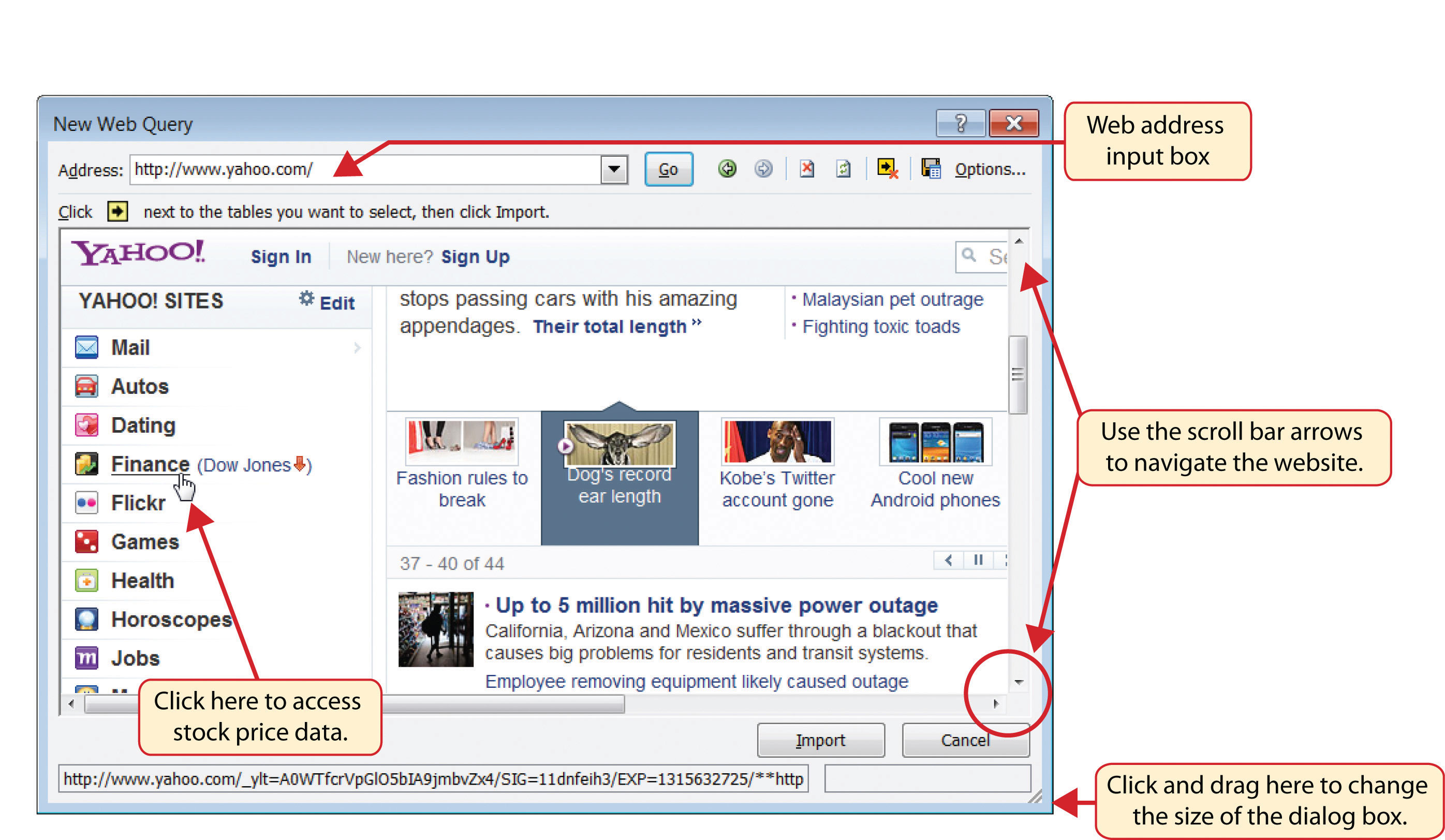6. Locate the Get Quotes input box by using the scroll bar to scroll to the top of the website (see Figure 3.49 "Get Quotes Input Box on Yahoo! Finance").
7. Click in the Get Quotes input box, type the stock symbol MSFT, and press the ENTER key on your keyboard (see Figure 3.49 "Get Quotes Input Box on Yahoo! Finance").

Figure 3.49 Get Quotes Input Box on Yahoo! Finance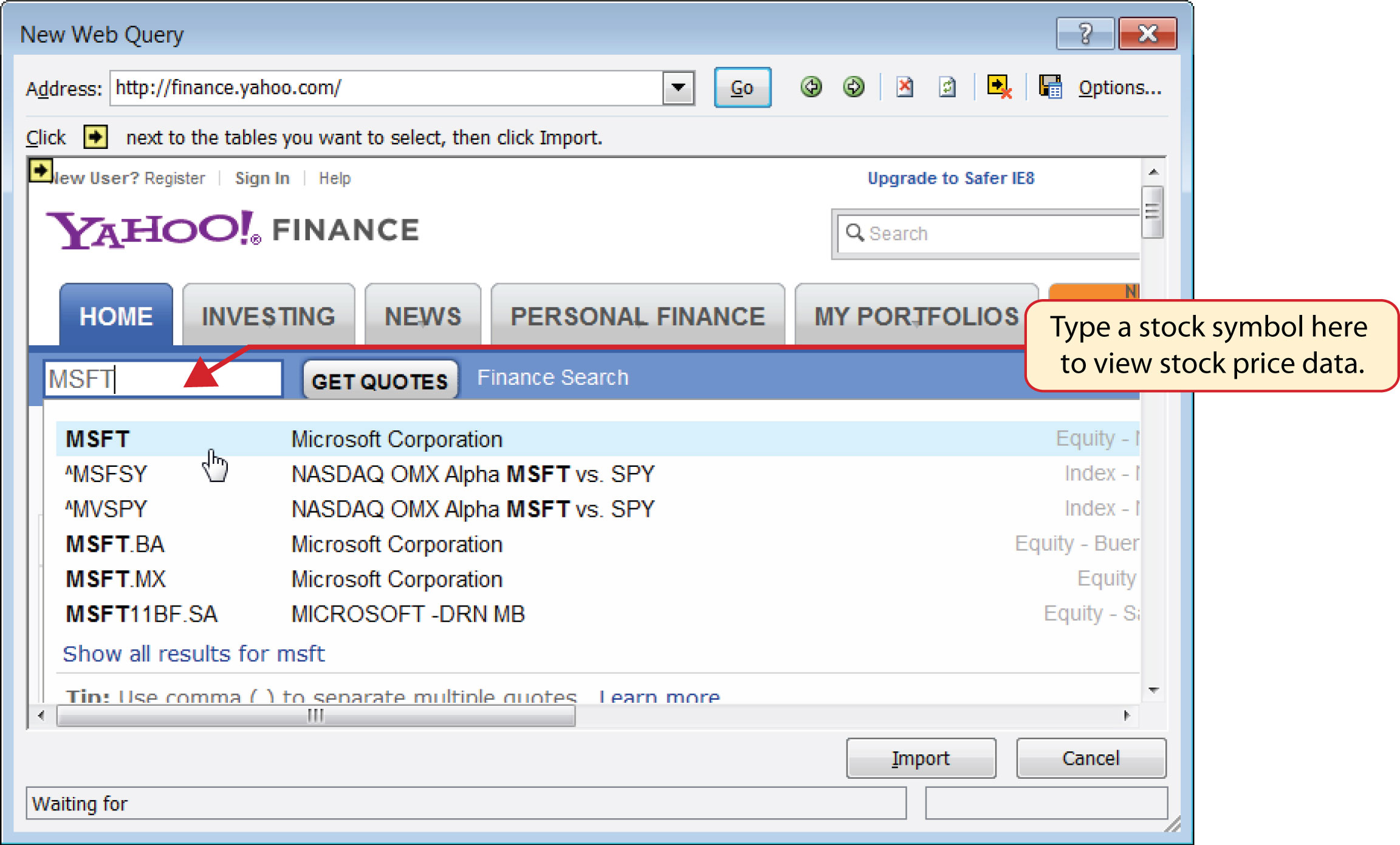8. When viewing the stock price data for MSFT, you will see several yellow boxes or tags with arrows (see Figure 3.50 "Selecting a Tag to Import Data from a Website"). These tags indicate blocks or tables of data that can be imported into a worksheet from a website. Click the yellow tag next to the Last Trade price. The yellow tag will turn green with a check mark inside of it.

Figure 3.50 Selecting a Tag to Import Data from a Website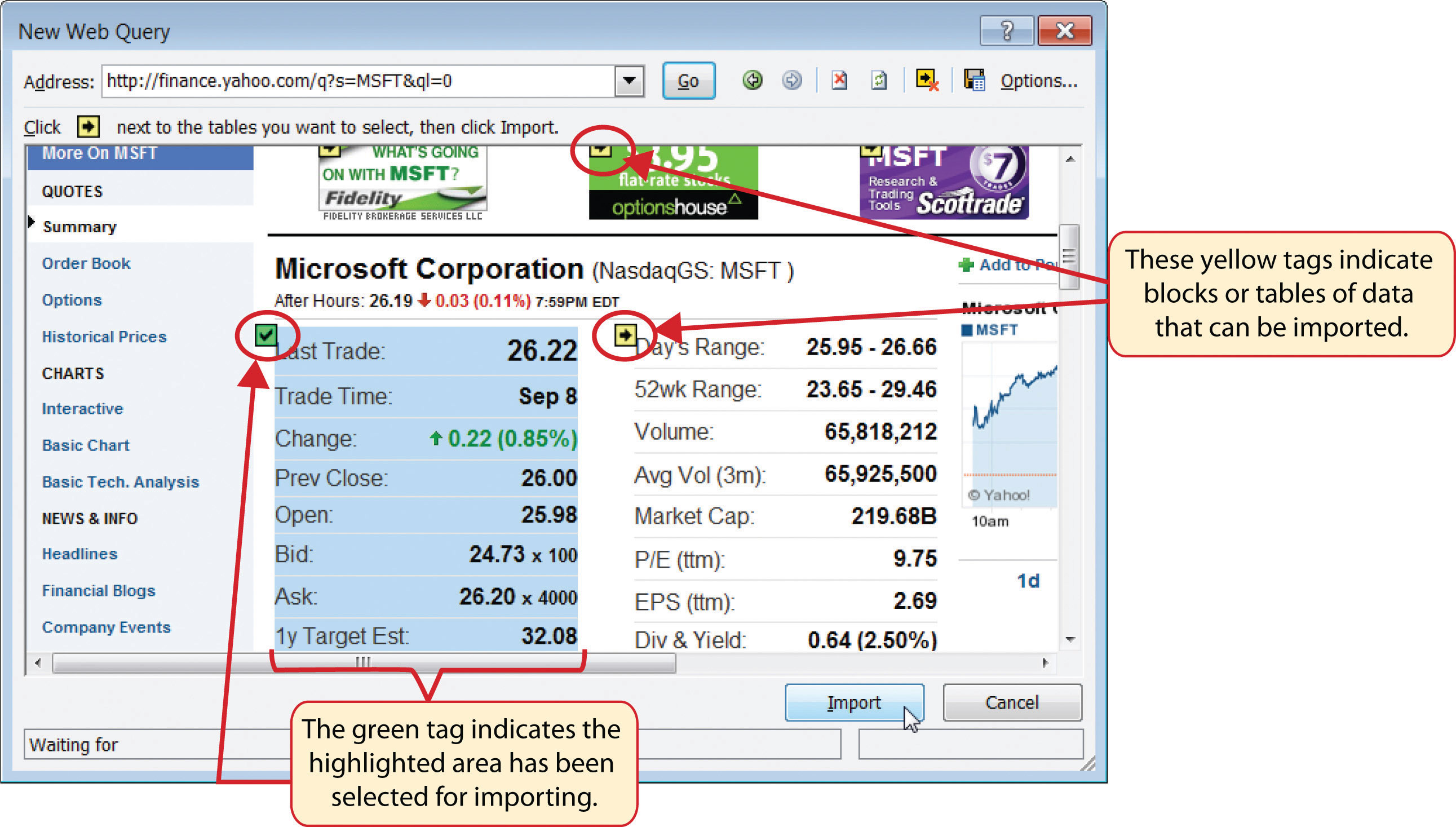9. Click the Import button at the bottom of the New Web Query dialog box. This will open the Import Data dialog box, as shown in Figure 3.51 "Import Data Dialog Box".

Figure 3.51 Import Data Dialog Box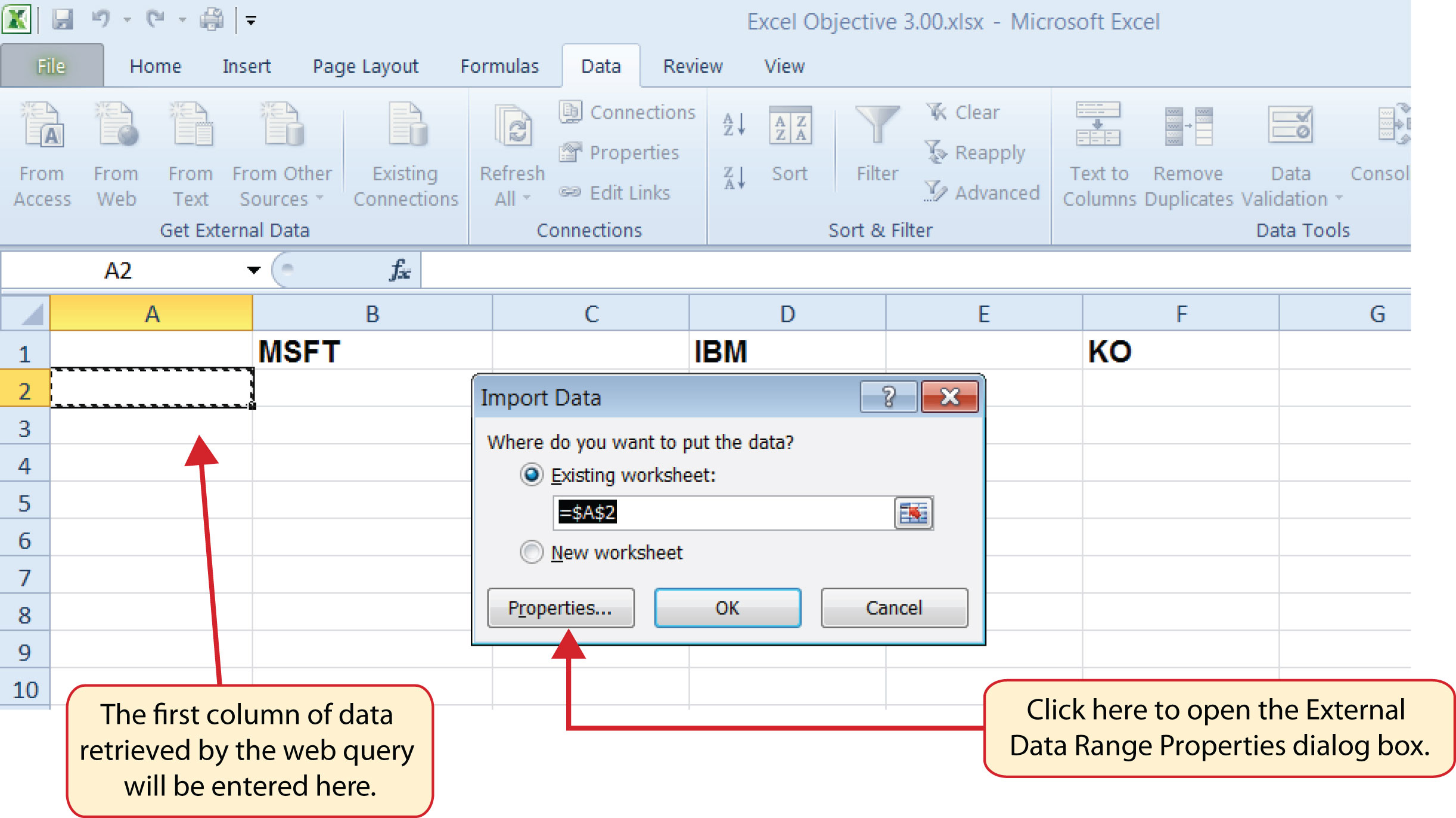10. Click the Properties button at the bottom of the Import Data dialog box (see Figure 3.51 "Import Data Dialog Box"). This will open the External Data Range Properties dialog box.
11. Click in the Name input box on the External Data Range Properties dialog box and delete the query name that is provided. Type the new query name Microsoft Stock Price.
12. Click the box next to the “Refresh every” property. Change the refresh minutes to 5. This means the query will automatically search the website every 5 minutes to retrieve an updated stock price for Microsoft (see Figure 3.52 "Final Settings for the External Data Range Properties Dialog Box").

Figure 3.52 Final Settings for the External Data Range Properties Dialog Box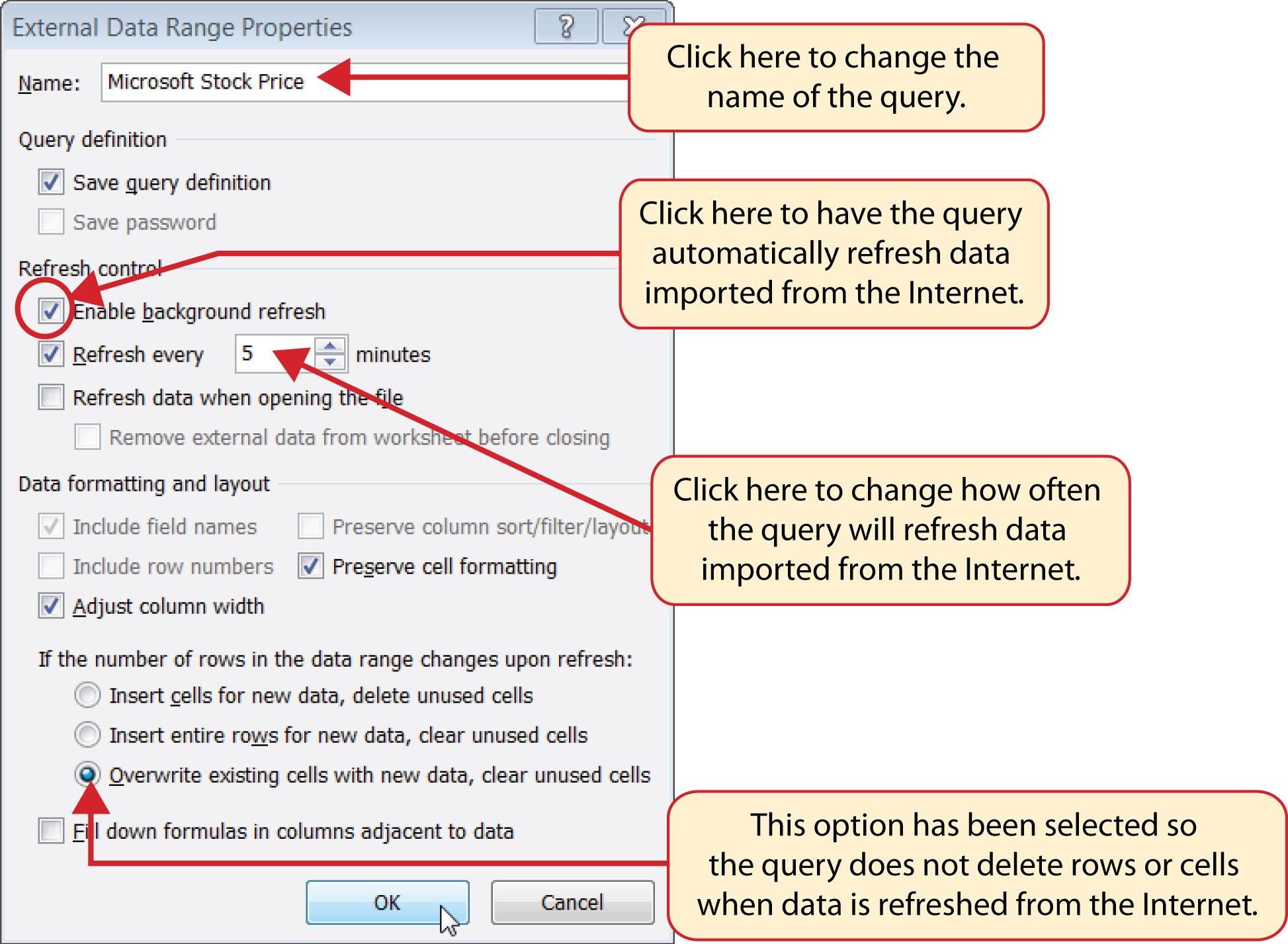13. Click the option at the bottom of the External Data Range Properties dialog box that reads “Overwrite existing cells with new data, clear unused cells” (see Figure 3.52 "Final Settings for the External Data Range Properties Dialog Box"). It is important to select this option if you intend to add more than one web query to a worksheet or if you are using formulas in adjacent columns to a web query. This option will prevent the web query from deleting entire rows of data from the worksheet.
14. Click the OK button at the bottom of the External Data Range Properties dialog box.
15. Click the OK button at the bottom of the Import Data dialog box.
16. After the data is imported, click cell H17 on the Investment Detail worksheet and type an equal sign (=).
17. Click the Price Data worksheet tab.
18. Click cell B2 on the Price Data worksheet and press the ENTER key on your keyboard. This will display the price that is imported from the web query on the Investment Detail worksheet.
19. Figure 3.53 "Stock Price for Microsoft Imported into the Price Data Worksheet" shows the results of importing the Microsoft stock price from the Yahoo! Finance website. It is important to note that the data you retrieve from the website will be different from what is shown in this figure.

Figure 3.53 Stock Price for Microsoft Imported into the Price Data Worksheet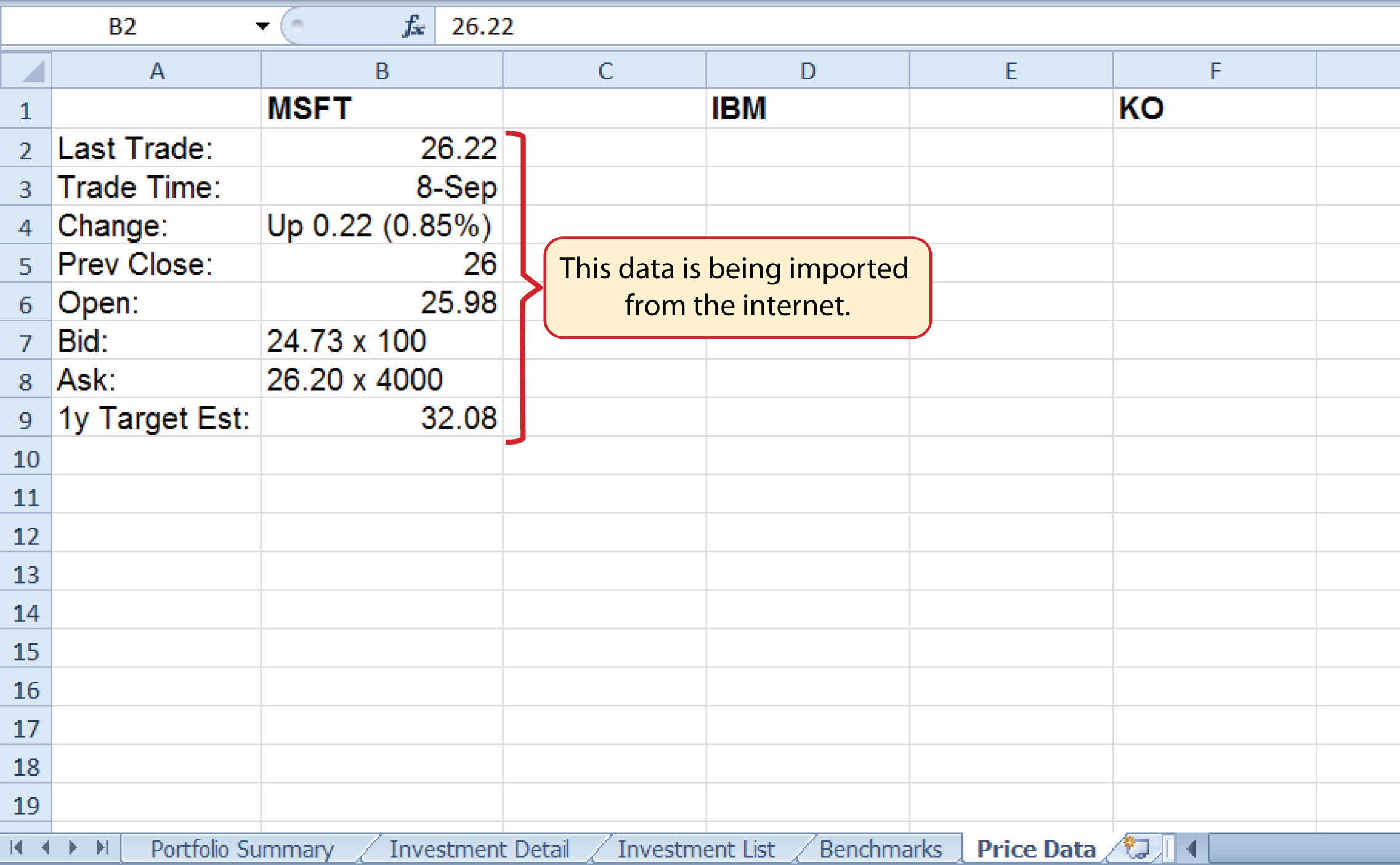### Skill Refresher: Web Queries

1. Click a cell location on a worksheet where the first column of the data being imported should appear.
2. Click the Data tab of the Ribbon.
3. Click the From Web button in the Get External Data group of commands.
4. Enter a website address in the Address input box on the New Web Query dialog box and press the ENTER key on your keyboard.
5. Use the scroll bars and website links to navigate the website.
6. Select one or more yellow tags that contain the block or blocks of data you wish to import.
7. Click the Import button at the bottom of the New Web Query dialog box.
8. Click the Properties button at the bottom of the Import Data dialog box.
9. Make any necessary changes on the External Data Range Properties dialog box.
10. Click the OK button at the bottom of the External Data Range Properties dialog box.
11. Click the OK button at the bottom of the Import Data dialog box.

### Key Takeaways

• Lookup functions are powerful and versatile tools because they eliminate the need to copy or recreate data that exists in other worksheets or workbooks.
• The VLOOKUP function will look vertically down the first column of the table array range to find the lookup value. The lookup value must exist in the first column of the table array range when using the VLOOKUP function.
• The HLOOKUP function will look horizontally across the first row of the table array range to find the lookup value. The lookup value must exist in the first row of the table array range when using the HLOOKUP function.
• If the Range_lookup argument for the VLOOKUP function is defined with the word TRUE, the data in the table array range must be sorted in ascending order (smallest to largest) based on the values in the first column.
• If the Range_lookup argument for the HLOOKUP function is defined with the word TRUE, the data in the table array range must be sorted in ascending order (smallest to largest), left to right, based on the values in the first row.
• If you are copying and pasting a VLOOKUP or HLOOKUP function to other cell locations on a worksheet, make sure there is an absolute reference placed on the table array range.
• Web queries are used to import external data from a website into an Excel worksheet.

### Exercises

1. Which of the following is true with respect to the range of cells used to define the Table_array argument when using the VLOOKUP function?

1. The lookup value must exist in the first row of this range.
2. The lookup value must exist in the first column of this range.
3. This range must be sorted in ascending order if the Range_lookup argument is defined with the word FALSE.
4. The range used to define the Table_array argument is the lookup value.
2. Assume that the range B3:E23 is used to define the Table_array argument for an HLOOKUP function. How should the Row_index_num argument be defined if the output of the function should be the contents of the cell location in Row 15 in the same column as the lookup value?

1. with the number 15
2. with the range B3:B15
3. with cell location B15
4. with the number 13
3. Column A contains values that will be used to define the Lookup_value argument of a VLOOKUP function. The function will search for an exact match to the lookup value in the range A2:D15 in the Description List worksheet. The output of the function should be the contents in the cell location in Column C of the same row as the lookup value. Once the function is created, it will be copied and pasted to other cell locations in the same column. Which of the following VLOOKUP functions will provide an accurate result based on these criteria?

1. =VLOOKUP(A4,‘Description List’!A2:D15,2,FALSE)
2. =VLOOKUP(\$A\$4,‘Description List’!\$A\$2:\$D\$15,2,FALSE)
3. =VLOOKUP(A4,‘Description List’!\$A\$2:\$D\$15,3,TRUE)
4. =VLOOKUP(A4,‘Description List’!\$A\$2:\$D\$15,3,FALSE)
4. When using a web query, what is the appropriate setting to make if you want Excel to search the website for new information every minute?

1. Click the “Refresh every” option and change the minutes to 1 in the Import Data dialog box.
2. Click the “Refresh every” option and change the minutes to 1 in the External Data Range Properties dialog box.
3. Click the “Refresh every” option and change the minutes to 1 in the New Web Query dialog box.
4. Click the “Refresh every” option and change the minutes to 1; also select the “Overwrite existing cells with new data, clear unused cells” option in the External Data Range Properties dialog box.

## 3.4 Chapter Assignments and Tests

To assess your understanding of the material covered in the chapter, please complete the following assignments.

### Careers in Practice (Skills Review)

Retail Inventory Analyst (Comprehensive Review Part A)

Starter File: Chapter 3 CiP Exercise 1

Difficulty: Level 1 Easy

The challenge of pursuing any position in a retail career is analyzing large volumes of data to measure the financial performance of the business. Large retail corporations may service thousands of customers in hundreds of stores every day. This creates an enormous amount of sales data that is typically stored in large database systems. A retail analyst is typically asked to make sense of all this data and develop reports for other managers in the company. In fact, a retail analyst is often asked to prepare sales reports for the most senior executives in a retail corporation. The skills covered in this chapter are extremely valuable in helping a retail analyst summarize large volumes of data that allow other managers to understand the financial performance of the company and make critical decisions every day. In this exercise, you will create a sales report similar to one that is commonly used in a retail career. This part of the exercise utilizes the IF function to analyze the sales and inventory data by store. The information created from this analysis can be shared with a manager in the shipping department. Begin this exercise by opening the file named Chapter 3 CiP Exercise 1.

1. Click cell D3 on the Sales by Store worksheet. Set the Freeze Panes command by clicking the View tab of the Ribbon and then clicking the Freeze Panes button. Select the Freeze Panes option from the drop-down list. This will keep the column headings and store numbers in view as you scroll through the worksheet.
2. Click cell L3 on the Sales by Store worksheet. Enter an IF function by typing an equal sign (=) followed by the function name IF and an open parenthesis ((). Define the arguments of the function as follows:

• Logical_test: The logical test will assess if the value in cell J3 is greater than 5%. Click cell J3 and then type >5%. Complete the argument by typing a comma.
• Value_if_true: If the logical test is true, the function should show the word Growth. Type the word “Growth” with the quotation marks as shown. Complete the argument by typing a comma.
• Value_if_false: If the logical test is false, the function should leave the cell blank. To do this, type two quotation marks (“”). Complete the function by typing a closing parenthesis and press the ENTER key on your keyboard.
3. Copy and paste the IF function in cell L3 into the range L4:L26 by double clicking the Auto Fill Handle (see Figure 3.54). You will see the word Growth only for stores where sales are growing at a rate greater than 5% compared to last year. The other cell locations in this column will remain blank if the growth rate is at or less than 5%.

Figure 3.54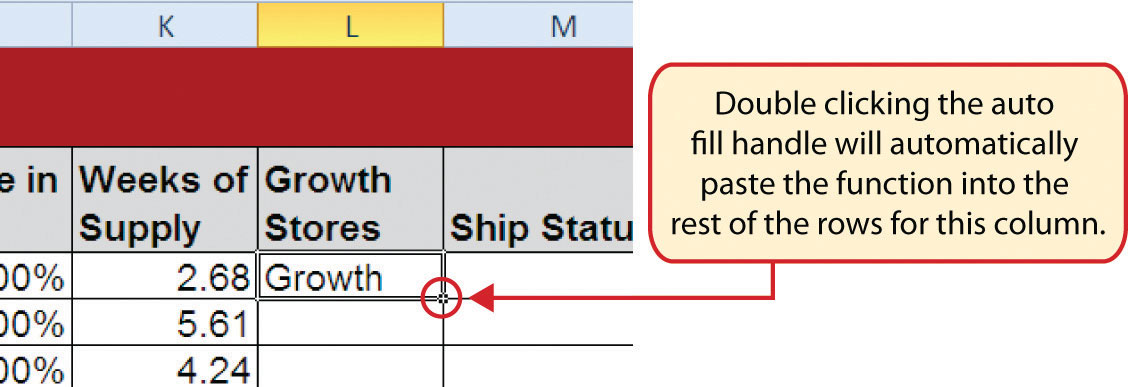Double click the Auto Fill Handle to copy and paste formulas and functions.

4. Click cell M3 on the Sales by Store worksheet. This column will use the AND function within the IF function to identify small stores that cannot receive any shipments until more inventory is sold. Begin the function by typing an equal sign (=) followed by the function name IF and an open parenthesis ((). Define the arguments of the function as follows:

• Logical_test: The logical test will assess if the store size in cell F3 is equal to 10000 and if the weeks of supply in cell K3 is greater than 8. This will require the use of the AND function. Type the function name AND followed by an open parenthesis. Define the first argument by clicking cell F3 and then typing the following: =10000. Type a comma and define the second argument by clicking cell K3 and then typing the following: >8. Type a closing parenthesis followed by a comma (),).
• Value_if_true: If the logical test is true, the function should show the word HOLD. Type “HOLD” with the quotation marks as shown. Complete the argument by typing a comma.
• Value_if_false: If the logical test is false, the function should show the word OK. Type “OK” with the quotation marks as shown. Complete the function by typing a closing parenthesis and press the ENTER key on your keyboard.
5. Copy and paste the IF function in cell M3 into the range M4:M26 by double clicking the Auto Fill Handle. The function now shows for any store that is 10,000 square feet if shipments should be held.
6. Click cell N3 on the Sales by Store worksheet. This column will show for all stores if the inventory is too high, low, or normal. This will require a nested IF function. Begin the function by typing an equal sign (=) followed by the function name IF and an open parenthesis ((). Define the arguments of the function as follows:

• Logical_test: The first logical test will assess if the weeks of supply in cell K3 is less than 4. Click cell K3 and then type <4. Complete the argument by typing a comma.
• Value_if_true: If the logical test is true, the function should show the word LOW. Type “LOW” with the quotation marks as shown. Complete the argument by typing a comma.
• Value_if_false: This argument will be used to begin a second IF function. Type the function name IF and an open parenthesis ((). Define the arguments of this second IF function as follows:
• Logical_test: This second logical test will assess if the weeks of supply in cell K3 is greater than 8. Click cell K3 and then type >8. Complete the argument by typing a comma.
• Value_if_true: If the logical test is true, the function should show the word HIGH. Type “HIGH” with the quotation marks as shown. Complete the argument by typing a comma.
• Value_if_false: IF the second logical test is false, the function should show the word NORMAL. Type “NORMAL” with the quotation marks as shown. Complete the function by typing two closing parentheses ())).Press the ENTER key.
7. Copy and paste the IF function in cell N3 into the range N4:N26 by double clicking the Auto Fill Handle. The function now highlights for each store if the inventory is low, high, or normal.
8. Click cell O3 on the Sales by Store worksheet. This column will use the OR function within the IF function to identify stores that should be prioritized for merchandise shipments. Begin the function by typing an equal sign (=) followed by the function name IF and an open parenthesis ((). Define the arguments of the function as follows:

• Logical_test: The logical test will assess if the change in sales in cell J3 is greater than 8% or if the weeks of supply in cell K3 is less than 5. This will require the use of the OR function. Type the function name OR followed by an open parenthesis ((). Define the first argument by clicking cell J3 and then typing the following: >8%. Type a comma and define the second argument by clicking cell K3 and then typing the following: <5. Type a closing parenthesis followed by a comma (),).
• Value_if_true: If the logical test is true, the function should show the word Priority. Type “Priority” with the quotation marks as shown. Complete the argument by typing a comma.
• Value_if_false: If the logical test is false, the function should leave the cell blank. To do this, type two quotation marks (“”). Complete the function by typing a closing parenthesis and press the ENTER key on your keyboard.
9. Copy and paste the IF function in cell O3 into the range O4:O26 by double clicking the Auto Fill Handle. The function now shows the word Priority for any store that is experiencing high sales growth or low inventory. This information can also be used by a shipping manager to prioritize the flow of deliveries to the stores.
10. Save the workbook by adding your name in front of the current workbook name (i.e., “your name Chapter 3 CiP Exercise 1”).
11. Close the workbook and Excel.

Figure 3.55 Completed CiP Exercise 1 Sales by Store Worksheet (Columns L–O)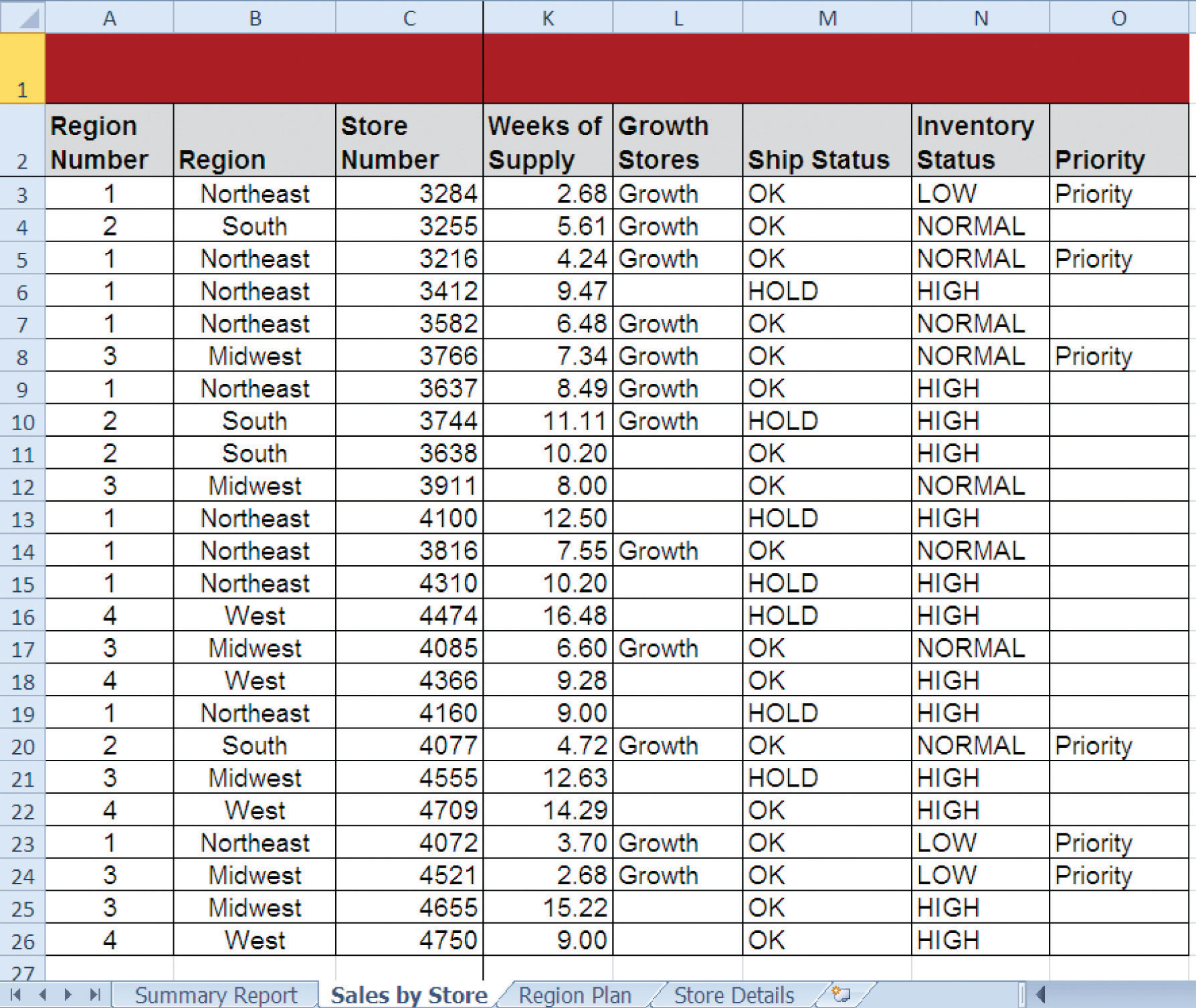### Careers in Practice (Skills Review)

Retail Sales Analyst (Comprehensive Review Part B)

Starter File: Chapter 3 CiP Exercise 1 (Continued from Comprehensive Review Part A)

Difficulty: Level 2 Moderate

This exercise continues the career theme for a retail analyst. In this exercise, you will summarize the detailed sales data using the statistical IF functions. The report will summarize the store level detail by region, which could be used by senior executives of the company. Begin this exercise by opening the file named Chapter 3 CiP Exercise 1 or continue with this file if you completed the comprehensive review part A.

1. Click cell C3 on the Summary Report worksheet. This column will be used to count the stores for each region of the company. Begin the function by clicking the Formulas tab on the Ribbon. Click the More Functions button, click the Statistical option, and then click the COUNTIF function from the list. Define the arguments in the Function Arguments dialog box as follows:

• Range: Click the Collapse Dialog button next to the Range argument, click the Sales by Store worksheet tab, and highlight the range A3:A26. Press the ENTER key on your keyboard. Click in the input box for the Range argument and place an absolute reference on the range. Press the TAB key on your keyboard to advance to the next argument.
• Criteria: Type cell A3. Complete the function by clicking the OK button at the bottom of the Function Arguments dialog box.
2. Copy and paste the COUNTIF function in cell C3 by double clicking the Auto Fill Handle. The function will show the number of stores for each region.
3. Click cell D3 on the Summary Report worksheet. This column will be used to sum the current sales by region. Begin the function by clicking the Formulas tab on the Ribbon. Click the Math & Trig button and select the SUMIF function from the list. Define the arguments in the Function Arguments dialog box as follows:

• Range: Click the Collapse Dialog button next to the Range argument, click the Sales by Store worksheet tab, and highlight the range A3:A26. Press the ENTER key on your keyboard. Click in the input box for the Range argument and place an absolute reference on the range. Press the TAB key on your keyboard to advance to the next argument.
• Criteria: Type cell A3. Press the TAB key on your keyboard to advance to the next argument.
• Sum_range: Click the Collapse Dialog button next to the Sum_range argument, click the Sales by Store worksheet tab, and highlight the range I3:I26. Press the ENTER key on your keyboard. Click in the input box for the Sum_range argument and place an absolute reference on the range. Complete the function by clicking the OK button at the bottom of the Function Arguments dialog box.
4. Copy and paste the SUMIF function in cell D3 by double clicking the Auto Fill Handle. The function will show the total sales this year for each region.
5. Click cell E3 on the Summary Report worksheet. This column will be used to sum the sales last year by region. Enter a SUMIF function and define the arguments exactly as stated in step 3. However, define the Sum_range argument with the range H3:H26 on the Sales by Store worksheet. Remember to put an absolute reference on this range before completing the function.
6. Copy and paste the SUMIF function in cell E3 by double clicking the Auto Fill Handle. The function will show the total sales last year for each region.
7. Enter a formula in cell F3 on the Summary Report worksheet to calculate the percent change in sales for each region. Your formula should first subtract the Sales Last Year in cell E3 from the Sales This Year in cell D3. Then divide this result by the Sales Last Year in cell E3.
8. Copy and paste the formula in cell F3 by double clicking the Auto Fill Handle.
9. Click cell C11 on the Summary Report worksheet. This column will be used to count the number of stores by size for the region number typed into cell B9. Begin the function by clicking the Formulas tab on the Ribbon. Click the More Functions button, click the Statistical option, and select the COUNTIFS function from the list. Define the arguments in the Function Arguments dialog box as follows:

• Criteria_range1: Click the Collapse Dialog button next to the Criteria_range1 argument, click the Sales by Store worksheet tab, and highlight the range A3:A26. Press the ENTER key on your keyboard. Click in the input box for the Criteria_range1 argument and place an absolute reference on the range. Press the TAB key on your keyboard to advance to the next argument.
• Criteria1: Type cell B9. Place an absolute reference on this cell location. Press the TAB key on your keyboard to advance to the next argument.
• Criteria_range2: Click the Collapse Dialog button next to the Criteria_range2 argument, click the Sales by Store worksheet tab, and highlight the range F3:F26. Press the ENTER key on your keyboard. Click in the input box for the Criteria_range2 argument and place an absolute reference on the range. Press the TAB key on your keyboard to advance to the next argument.
• Criteria2: Type cell B11. Complete the function by clicking the OK button at the bottom of the Function Arguments dialog box.
10. Copy the COUNTIFS function in cell C11 and paste it into the range C12:C14 using the Paste Formulas command.
11. Click cell D11 on the Summary Report worksheet. This column will be used to sum the current sales by store size for the region number typed into cell B9. Begin the function by clicking the Formulas tab on the Ribbon. Click the Math & Trig button, and select the SUMIFS function from the list. Define the arguments in the Function Arguments dialog box as follows:

• Sum_range: Click the Collapse Dialog button next to the Sum_range argument, click the Sales by Store worksheet tab, and highlight the range I3:I26. Press the ENTER key on your keyboard. Click in the input box for the Sum_range argument and place an absolute reference on the range. Press the TAB key on your keyboard to advance to the next argument.
• Criteria_range1: Click the Collapse Dialog button next to the Criteria_range1 argument, click the Sales by Store worksheet tab, and highlight the range A3:A26. Press the ENTER key on your keyboard. Click in the input box for the Criteria_range1 argument and place an absolute reference on the range. Press the TAB key on your keyboard to advance to the next argument.
• Criteria1: Type cell B9. Place an absolute reference on this cell location. Press the TAB key on your keyboard to advance to the next argument.
• Criteria_range2: Click the Collapse Dialog button next to the Criteria_range2 argument, click the Sales by Store worksheet tab, and highlight the range F3:F26. Press the ENTER key on your keyboard. Click in the input box for the Criteria_range2 argument and place an absolute reference on the range. Press the TAB key on your keyboard to advance to the next argument.
• Criteria2: Type cell B11. Complete the function by clicking the OK button at the bottom of the Function Arguments dialog box.
12. Copy the SUMIFS function in cell D11 and paste it into the range D12:D14 using the Paste Formulas command.
13. Click cell E11 on the Summary Report worksheet. This column will be used to sum the sales last year by store size for the region number typed into cell B9. Enter a SUMIFS function into this cell location and define the arguments exactly as stated for step 11. However, define the Sum_range argument with the range H3:H26. Remember to place an absolute reference on this range.
14. Copy the SUMIFS function in cell E11 and paste it into the range E12:E14 using the Paste Formulas command.
15. Enter a formula in cell F11 on the Summary Report worksheet to calculate the percent change in sales for each store size. Your formula should first subtract the Sales Last Year in cell E11 from the Sales This Year in cell D11. Then divide this result by the Sales Last Year in cell E11.
16. Copy the formula in cell F11 and paste it into the range F12:F14 using the Paste Formulas command.
17. Place a conditional format on the range F11:F14 to show any negative numbers in red. Begin by highlighting the range F11:F14 on the Summary Report worksheet. Click the Conditional Formatting button in the Home tab of the Ribbon and select the New Rule option from the drop-down list. Click the “Format only cells that contain” option from the New Formatting Rule dialog box. Change the comparison operator box from “between” to “less than”. Click in the input box next to the comparison operator box and type a 0. Click the Format button and change the text color to red. Complete the command by clicking the OK button in the Format Cells dialog box and in the new New Formatting Rule dialog box.
18. Click cell G3 on the Summary Report worksheet. The purpose of this column is to show the sales growth target for each region. The sales growth targets can be found in the Region Plan worksheet. An HLOOKUP function will be used to display the sales growth plan for each region. Begin the function by clicking the Formulas tab of the Ribbon. Then click the Lookup & Reference button and select the HLOOKUP function from the list. Define the arguments of the function as follows:

• Lookup_value: Type cell A3 in the input box for this argument. Then press the TAB key on your keyboard to advance to the next argument.
• Table_array: Click the Collapse Dialog button next to the Table_array argument, click the Region Plan worksheet tab, and highlight the range B2:E5. Press the ENTER key on your keyboard. Click in the input box for the Table_array argument and place an absolute reference on the range. Press the TAB key on your keyboard to advance to the next argument.
• Row_index_num: Type the number 4 and press the TAB key on your keyboard.
• Range_lookup: Type the word FALSE. Complete the function by clicking the OK button at the bottom of the Function Arguments dialog box.
19. Copy and paste the HLOOKUP function in cell G3 by double clicking the Auto Fill Handle.
20. Enter a formula in cell H3 that subtracts the Sales Growth Target in cell G3 from the Change in Sales in cell F3 (F3−G3). Then copy and paste the formula into the range H4:H6.
21. Click cell C9 on the Summary Report worksheet. The purpose of this cell is to display the name of the region for the number that is typed into cell B9. This will be accomplished by using a VLOOKUP function. Begin the function by clicking the Formulas tab of the Ribbon, then click the Lookup & Reference button and select the VLOOKUP function from the list. Define the arguments of the function as follows:

• Lookup_value: Type cell B9 in the input box for this argument and press the TAB key on your keyboard to advance to the next argument.
• Table_array: Click the Collapse Dialog button next to the Table_array argument and highlight the range A3:B6 on the Summary Report worksheet. Press the ENTER key on your keyboard. Press the TAB key on your keyboard to advance to the next argument.
• Col_index_num: Type the number 2 and press the TAB key on your keyboard.
• Range_lookup: Type the word FALSE. Complete the function by clicking the OK button at the bottom of the Function Arguments dialog box.
22. Looking at the Summary Report worksheet, you will notice that the change in sales for the West region is −4.77%. Type the number 4 in cell B9 to see the change in sales summarized for each store size in the region. Examine the sales results by store size for each of the other three regions. Notice that even if a region is showing an increase in sales over last year, it does not necessarily mean that all stores in that region are performing well.
23. Save the workbook by adding your name in front of the current workbook name (i.e., “your name Chapter 3 CiP Exercise 1”).
24. Close the workbook and Excel.

Figure 3.56 Completed CiP Exercise 1 Summary Report Worksheet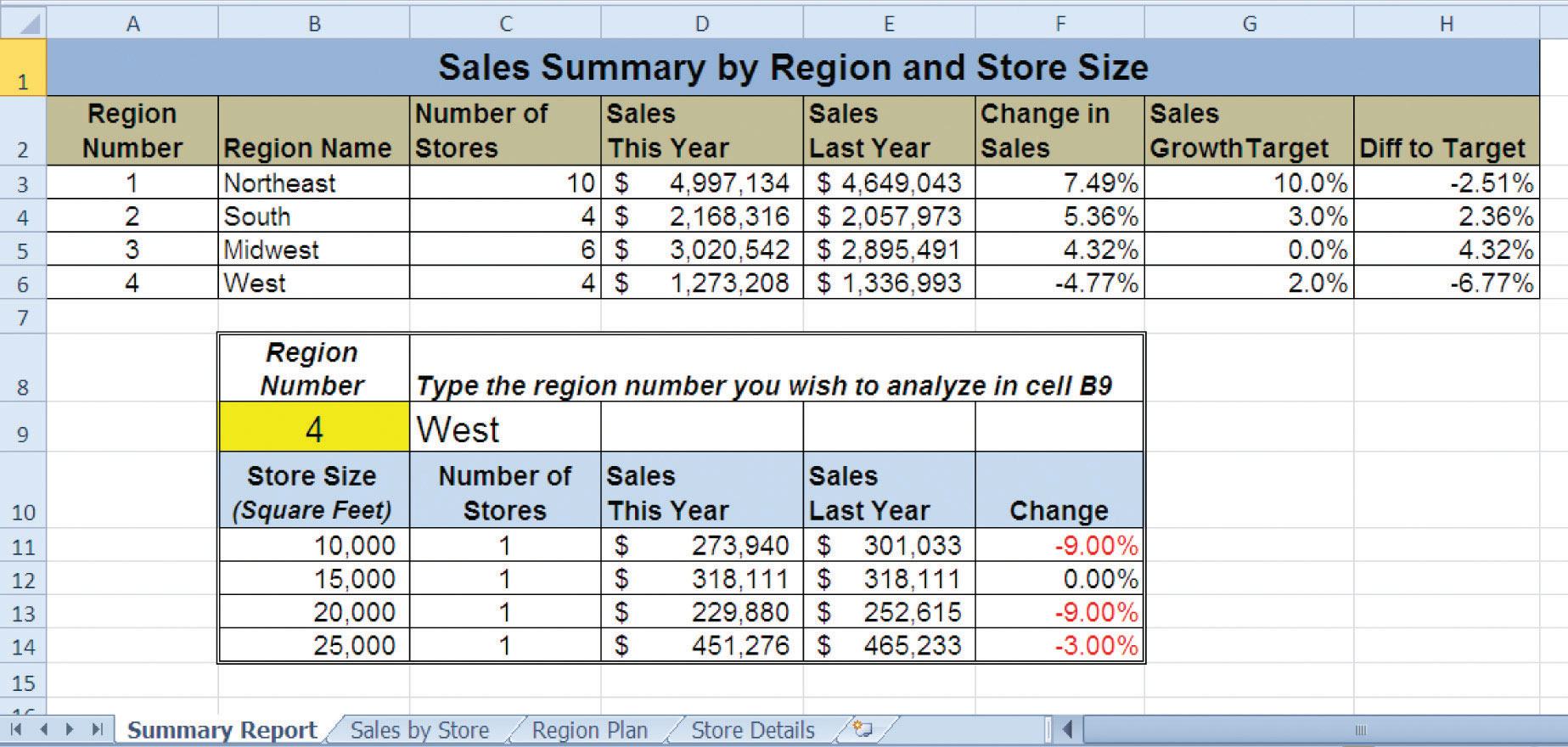Payroll for a Medical Group

Starter File: Chapter 3 CiP Exercise 2

Difficulty: Level 2 Moderate

Medical groups are common in the health care industry and range in size. The defining trait of a medical group is that there is more than one doctor on staff for a particular practice, which creates more flexible hours for patients and physicians. In addition, a medical group can provide a variety of medical services in one location. This exercise illustrates how to use the skills presented in this chapter to summarize payroll details for employees working in a medical group. Begin this exercise by opening the file named Chapter 3 CiP Exercise 2.

1. Set the Freeze Panes command on the Payroll Details worksheet so that Rows 1 and 2 and Columns A and B are locked in place while scrolling through the worksheet.
2. Enter an IF function in cell G3 on the Payroll Details worksheet to calculate the Social Security tax. Social Security funds are used by the government to provide income for people who are retired, a beneficiary of a retiree, or disabled. An employer must withhold 4.2% of an employee’s weekly pay for Social Security. However, an employee is only taxed up to \$100,000. The logical test of the IF function should assess if the value in the Pay Year to Date column is greater than or equal to 100000. If the logical test is true, the output of the function should be zero. Otherwise, the function should multiply the value in the Gross Pay This Week by 4.2%. Copy and paste the function into the range G4:G22.
3. Enter a formula in cell H3 on the Payroll Details worksheet that calculates the Medicare Tax. Medicare funds are used by the government to provide medical financial support to senior citizens. Your formula should multiply the Gross Pay This Week by 1.45%. Copy and paste this formula into the range H4:H22.
4. Enter a formula in cell I3 on the Payroll Details worksheet that calculates the total FICA tax (FICA stands for Federal Insurance Contributions Act). Your formula should add the Social Security Tax to the Medicare Tax. Copy and paste this formula into the range I4:I22.
5. Enter an IF function in cell J3 on the Payroll Details worksheet to calculate the Federal Tax. If the Gross Pay This Week is less than or equal to 1150, then the tax is calculated by multiplying the Gross Pay This Week by 20%. Otherwise, this tax is calculated by multiplying the Gross Pay This Week by 25%. Copy and paste the IF function into the range J4:J22.
6. If an employee has been working with the medical group for 1 or more years, the company will match 50% of the employee’s 401(k) retirement contributions. Calculate the company’s 401(k) retirement contributions by entering an IF function in cell L3 on the Payroll Details worksheet. If the Years of Service is greater than or equal to 1, multiply the 401K Retirement value by 50%. Otherwise, the output of the function should be zero. Copy and paste the IF function into the range L4:L22.
7. The medical group offers its employees an additional retirement benefit based on the employee’s position and years of service. Employees who are with the practice 3 or more years will receive an additional contribution to their retirement account. Calculate this benefit by entering an IF function in cell M3 on the Payroll Details worksheet. If an employee is a physician and has been working in the medical group for 3 or more years, the function should multiply the Gross Pay This Week by 8%. For all other employees who have been working at the medical group for 3 or more years, the function should multiply the Gross Pay This Week by 5%. Copy and paste the function into the range M4:M22.
8. Enter a formula into cell N3 on the Payroll Details worksheet that calculates the Total Retirement Benefits. Your formula should add the 401K Company Match value to the Retirement Benefit value. Copy and paste the formula into the range N4:N22.
9. Enter a formula into cell O3 on the Payroll Details worksheet that calculates the Net Payment for each employee. Your formula should subtract the values in the range I3:K3 from the Gross Pay This Week in cell F3. The range I3:K3 includes the following: Total FICA (cell I3), Federal Tax (cell J3), and 401K Retirement (cell K3). Copy and paste this formula into the range O4:O22.
10. Enter a COUNTIF function into cell B3 on the Payroll Summary worksheet. The function should count the number of employees in the range A3:A22 on the Payroll Details worksheet for each Practice listed in Column A on the Payroll Summary worksheet. Copy and paste the function into the range B4:B7.
11. Enter an AVERAGEIF function into cell C3 on the Payroll Summary worksheet. The function should calculate the average Years of Service in the range D3:D22 on the Payroll Details worksheet for each Practice listed in Column A on the Payroll Summary worksheet. Copy and paste the function into the range C4:C7.
12. Enter a SUMIF function into cell D3 on the Payroll Summary worksheet. The function should sum the Total FICA tax in the range I3:I22 on the Payroll Details worksheet for each Practice listed in Column A on the Payroll Summary worksheet. However, the government requires that employers match the FICA tax that is withheld from employees’ paychecks. Therefore, multiply the result of this SUMIF function by 2. Copy and paste the function into the range D4:D7.
13. Enter a SUMIF function into cell E3 on the Payroll Summary worksheet. The function should sum the Federal Tax withholdings in the range J3:J22 on the Payroll Details worksheet for each Practice listed in Column A on the Payroll Summary worksheet. Copy and paste the function into the range E4:E7.
14. Enter a SUMIF function into cell F3 on the Payroll Summary worksheet. The function should sum the Net Payments in the range O3:O22 on the Payroll Details worksheet for each Practice listed in Column A on the Payroll Summary worksheet. Copy and paste the function into the range F4:F7.
15. Enter a SUMIF function into cell G3 on the Payroll Summary worksheet. The function should sum the Total Retirement Benefits in the range N3:N22 on the Payroll Details worksheet for each Practice listed in Column A on the Payroll Summary worksheet. Copy and paste the function into the range G4:G7.
16. Enter a SUM function in cell B8 on the Payroll Summary worksheet that sums the values in the range B3:B7. Copy and paste this SUM function into the range D8:G8. Then format the range D8:G8 to the Accounting number format.
17. Save the workbook by adding your name in front of the current workbook name (i.e., “your name Chapter 3 CiP Exercise 2”).
18. Close the workbook and Excel.

Figure 3.57 Completed CiP Exercise 2 Payroll Details Worksheet (Columns G–O)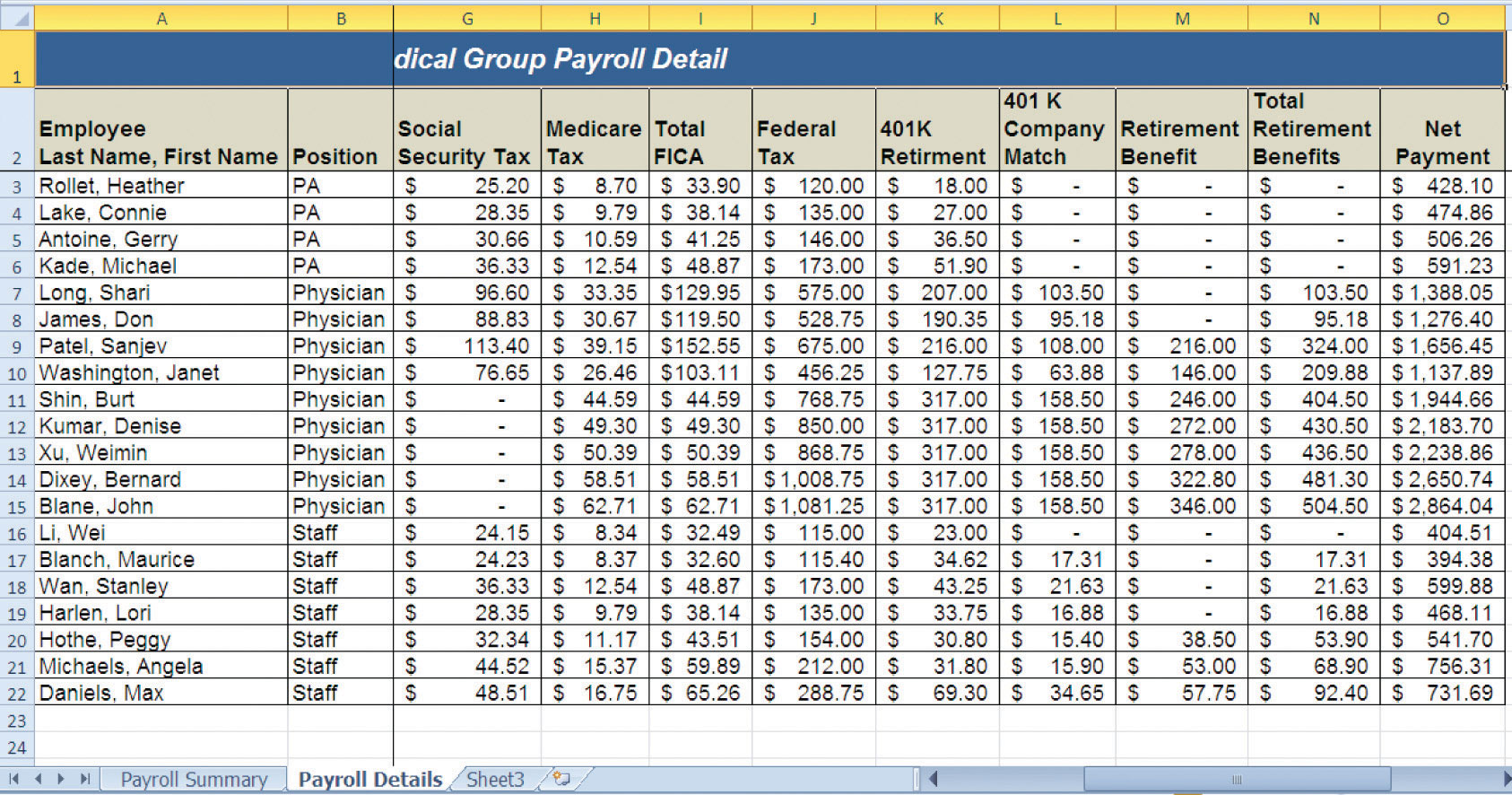Figure 3.58 Completed CiP Exercise 2 Payroll Summary Worksheet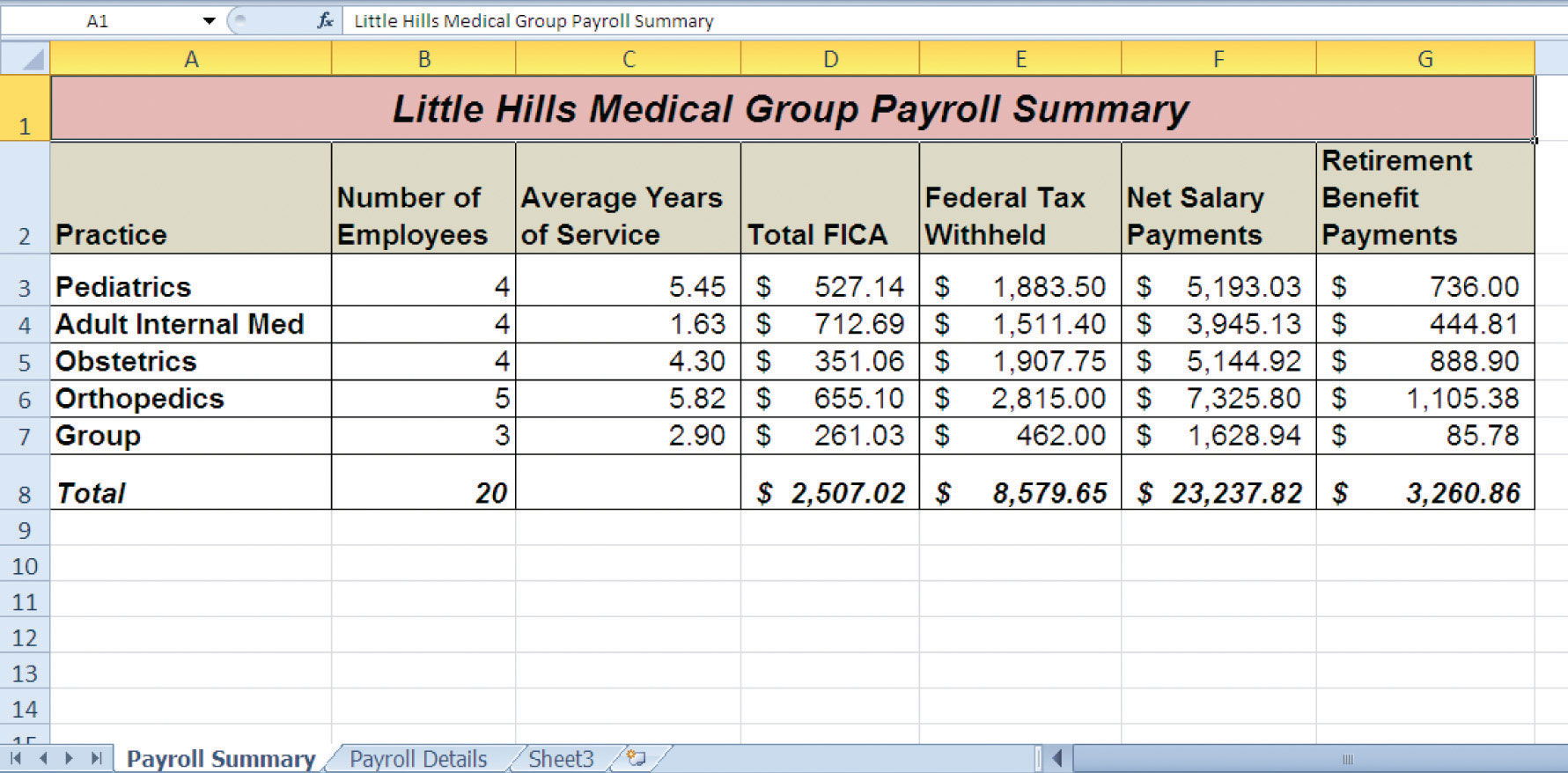### Integrity Check

Starter File: Chapter 3 IC Exercise 3

Difficulty: Level 3 Difficult

The purpose of this exercise is to analyze a worksheet to determine whether there are any integrity flaws. Read the scenario that follows and then open the Excel workbook related to this exercise. You will find a worksheet in the workbook named AnswerSheet. This worksheet is to be used for any written responses required for this exercise.

Scenario

You recently became the inventory director for a medium-size apparel retail company. The company relies on you to keep a very close watch on the inventory levels for all the items the company sells. Any apparel inventory that is left unsold after the current season will have to be sold at a significant discount. Large excess amounts of inventory can result in drastic profit losses for the company. The company analyzes inventory based on a sell-through rate, which is the weekly unit sales divided by the current inventory units. A sell-through percentage between 12% and 15% is considered normal. At this rate the company will sell all inventory for an item in 6 to 8 weeks. However, a sell-through rate that is outside of this range will require immediate action. An analyst has e-mailed you an inventory status report based on the company’s inventory management guidelines. He explains the following in his e-mail:

• The Status column of the inventory contains a function I put together that tells us where we have issues. The company suggests that we put a “rush priority” on any inventory orders for more than 100 units in a week and for which the sell-through rate is greater than or equal to 20%.
• If the sell-through rate for any item is less than 10%, we have to work with the buyers to see if any incoming orders can be cancelled. The function I created indicates any slow-selling items with a “cancel warning” description.
• Overall, it looks like our biggest problem is that we don’t have enough inventory. In fact, most of the items on the report are showing a “rush priority.” I guess this is a good problem to have.

Assignment

1. Look at the Status column (Column E) for the first few items on the inventory report. Does the output of the function created by the analyst make sense given the company’s guidelines for managing inventory? Document your observation in the AnswerSheet worksheet.
2. Evaluate the construction of the IF function in the Status column for the first item. Does the function accurately evaluate the Sell Through percentages in Column D? Document your observation in the AnswerSheet worksheet.
3. The company suggests that a rush priority be placed on any orders if the unit sales for an item are greater than 100 and the sell-through rate is greater than or equal to 20%. Does the IF function in the Status column accurately account for this condition? Document your observation in the AnswerSheet worksheet.
4. Enter a new IF function in the Status column to accurately assess the inventory for each item based on the company’s inventory policies. Make any other adjustments to the worksheet so that it is easy to see all items that require “Rush Priorities” as well “Cancel Warnings.”

### Applying Excel Skills

Keeping a Stock Portfolio Current with Web Queries

Starter File: Chapter 3 AES Assignment 1

Difficulty: Level 3 Difficult

The purpose of this exercise is to create a worksheet that tracks a small stock portfolio by utilizing web queries to bring in current price data from the web. The Excel workbook for this assignment contains a small subset of data from the Personal Investment Portfolio that was used to demonstrate the skills in this chapter. Your assignment is to complete the workbook based on the following requirements:

1. The Price Data worksheet contains symbols for five stocks. Create a web query for each stock symbol that imports the current stock price from Yahoo! Finance. Set the properties for each web query to retrieve data from the web every 2 minutes. Make sure that the first column of the web query for MSFT begins in cell location A2 on the Price Data worksheet.
2. Complete the Current Price column on the Investment Detail worksheet using an HLOOKUP function. Your function should display the “Last Trade” price from the Price Data worksheet for the appropriate stock symbol in Column B in the Investment Detail worksheet.
3. Enter formulas in Columns G, H, and I to calculate the Cost of Purchase, Current Value, and Unrealized Gain/Loss. Refer to Table 3.2 "Definitions for Columns A through G of the Investment Detail Worksheet", Table 3.3 "Definitions for Columns H through K of the Investment Detail Worksheet", and Table 3.5 "Definitions for Columns S through X of the Investment Detail Worksheet" earlier in this chapter if needed to create these formulas.
4. Show the total Unrealized Gain/Loss for the portfolio in cell I8.
5. For any stock, or for the entire portfolio, that is showing an Unrealized Loss in Column I, have Excel automatically change the font color to red and use a bold format.

A Second Look at Federal Income Taxes

Starter File: Chapter 3 AES Assignment 2

Difficulty: Level 3 Difficult

The purpose of this assignment is to revisit the federal payroll tax calculations that were demonstrated in the Careers in Practice: Payroll for a Medical Group exercise. In that exercise, we assumed that if the employee’s weekly income was less than or equal to \$1,150, the federal tax was estimated at 20%. For any income over \$1,150, the federal tax was estimated at 25%. However, the IRS publishes tables that provide more details as to how federal taxes should be calculated and withheld for weekly payrolls.

The Excel workbook for this assignment contains two worksheets. The Payroll Details worksheet is a subset of the data that was used for the Careers in Practice exercise. The Withholding Table worksheet contains part of an Excel worksheet that was published by the IRS. There are six levels of weekly income in Columns A and B of the worksheet. Columns C and D provide information for calculating the amount of federal tax that should be withheld. For example, if an employee’s weekly salary is \$200, you would use Row 3 to calculate the amount of federal tax that should be withheld. This means you would first subtract the value in cell C3 from the weekly salary, which is: 200 − 111.15 = 88.85. Then the result of 88.85 is multiplied by the percentage in cell D3, which is: 88.85 × .10 = 8.885. Therefore, the federal tax that should be withheld for an employee who is paid \$200 a week is \$8.885. Expressed as a formula, the calculation would be as follows: (200 − 111.15) × .10.

Your assignment is to complete the Federal Tax calculations in Column F of the Payroll Details worksheet. You are required to reference the data in the Withholding Table worksheet such that if the tax rates change in the future (a very common occurrence), your federal tax calculations will automatically be updated. Hint: You will need to use the VLOOKUP function twice in your formula. Follow the example calculation carefully and manually check the output of your formula to determine whether you are producing an accurate result.

Starter File: Chapter 3 AES Assignment 3

Difficulty: Level 3 Difficult

The Excel workbook for this assignment contains a partially completed grade book for a typical college-level course. The Grade Details worksheet contains a list of hypothetical students who were enrolled in the course as well as the grades they received for each course requirement. Column H contains a formula that calculates the final grade for each student. Table 3.12 "Final Grade Calculation" shows information that was included in the course syllabus that shows students how their final grades will be calculated.

Paper 1 10
Paper 2 10
Class Participation 15
Quizzes 10
Midterm Exam 20
Final Exam 35
Special Note A final grade below a 70 will require that this course be repeated for credit.

The Excel skills demonstrated in this chapter can be used to create a dynamic and “smart” grade book for any academic course. Your assignment is to complete the grade book using several of the Excel skills that you have learned so far. Open the Excel workbook for this assignment and complete the grade book based on the following requirements:

1. It is very important to make sure a student’s grade is accurately calculated. One area that must be checked often when calculating final grades is the percentages assigned to each requirement in the course. One way to do this is to see if the percentages add up to 100%. Use an IF function in the merged cells H2 and I2 on the Grade Details worksheet to determine if the percentages in the range B2:G2 add up to 100%. If the percentages do not add up to 100%, show the phrase “Percentages do not equal 100%.” Otherwise, leave the cell blank (use two quotation marks: “”). Use the results of your IF function to make any necessary adjustments to the grade book (refer to Table 3.12 "Final Grade Calculation" as needed).
2. Calculate class averages in Row 29 for each course requirement as well as the final grades in Columns B through H.
3. Use a VLOOKUP function to display the appropriate letter grade for each student in Column I. The Grade Table worksheet contains data that show how the letter grades should be assigned for the numeric grades.
4. Complete the Class Grade Distribution in the range I32:I43 on the Grade Details worksheet. The purpose of this area is to show how many students received the grades listed in the range H32:H43. For example, how many students receive an A, A−, and so on. Include a percent of total for each grade. Hint: This can be accomplished using the COUNTIF function if you successfully complete the VLOOKUP function in step 3. If you are unable to complete step 3, you can still complete this step using the COUNTIFS function.
5. Add any formatting enhancements to the Grade Details worksheet that would make the worksheet easier to read and use. Consider the “Special Note” in Table 3.12 "Final Grade Calculation".

### Chapter Skills Test

Starter File: Chapter 3 Skills Test

Difficulty: Level 2 Moderate

Answer the following questions by executing the skills on the starter file required for this test. Answer each question in the order in which it appears. If you do not know the answer, skip to the next question. Open the starter file before you begin this test.

1. Set the Freeze Panes command so that Rows 1 and 2 and Columns A and B are locked in place while scrolling through the Investment Detail worksheet.
2. In the Investment Detail worksheet, enter an IF function into cell I3. The output of the function should be the word Gain if the Current Investment Value in cell H3 is greater than the Cost of Purchase in cell G3. Otherwise, the output of the function should be the words No Gain.
3. Copy the IF function in cell I3 and paste it into the range I4:I17 using the Paste Formulas command.
4. In the Investment Detail worksheet, enter a nested IF function into cell F3. If the Dividend/Yield value in cell E3 is less than 2%, show the word Low. If the Dividend/Yield value in cell E3 is greater than or equal to 5%, show the word High. Otherwise, show the word Moderate.
5. Copy the IF function in cell F3 and paste it into the range F4:F17 using the Paste Formulas command.
6. On the Investment Detail worksheet in cell O3, use the OR function within an IF function to evaluate the Current vs. Target value. If the Current vs. Target value in cell N3 is greater than 2% or less than −2%, show the word REBAL. Otherwise, show the word OK.
7. Copy the IF function in cell O3 and paste it into the range O4:O17 using the Paste Formulas command.
8. On the Investment Detail worksheet in cell P3, use the AND function within an IF function to evaluate the Current vs. Target value and the Unrealized Gain/Loss value. If the Current vs. Target value in cell N3 is greater than 2% and if the Unrealized Gain/Loss value in cell J3 is greater than 0, show the word BUY. Otherwise, show the word HOLD.
9. Copy the IF function in cell P3 and paste it into the range P4:P17 using the Paste Formulas command.
10. On the Investment Detail worksheet, apply a conditional format to the range Q3:Q17. If the Months Owned is less than 12, change the font color to red. Otherwise, the font color should remain black.
11. On the Investment Detail worksheet, enter a VLOOKUP function in cell D3 that displays the Growth Last Year for the symbol in cell B3. The growth last year for all investments can be found in Column E on the Investment List worksheet. Your function should look for an exact match to the lookup value. Consider that this function will be copied and pasted into the range D4:D17 when defining the arguments.
12. On the Investment Detail worksheet, format the range D3:D17 to a percentage with two decimal places. Then copy the VLOOKUP function in cell D3 and paste it into the range D4:D17 using the Paste Formulas command.
13. On the Portfolio Summary worksheet, use the COUNTIF function in cell B3 to count the number of investments that match the investment type in cell A3. The function should look for and count the number of investment types in the range A3:A17 on the Investment Detail worksheet. Consider that this function will be copied and pasted into the range B4:B6 when defining the arguments.
14. Copy the COUNTIF function in cell B3 and paste it into the range B4:B6 using the Paste Formulas command.
15. On the Portfolio Summary worksheet, use the AVERAGEIF function in cell C3 to calculate the average Dividend/Yield for the investment type in cell A3. The function should calculate the average using the data in the range E3:E17 on the Investment Detail worksheet. The function should look for a match to the investment type in the range A3:A17 on the Investment Detail worksheet. Consider that this function will be copied and pasted into the range C4:C6 when defining the arguments.
16. Copy the AVERAGEIF function in cell C3 and paste it into the range C4:C6 using the Paste Formulas command.
17. On the Portfolio Summary worksheet, use the SUMIF function in cell D3 to calculate the Percent of Portfolio for the investment type in cell A3. The function should calculate the sum using the data in the range L3:L17 on the Investment Detail worksheet. The function should look for a match to the investment type in the range A3:A17 on the Investment Detail worksheet. Consider that this function will be copied and pasted into the range D4:D6 when defining the arguments.
18. Copy the SUMIF function in cell D3 and paste it into the range D4:D6 using the Paste Formulas command.
19. On the Portfolio Summary worksheet, use the HLOOKUP function to display the Portfolio Target in cell E3. The function should look for the Investment Type in cell A3 in Row 2 of the Portfolio Targets worksheet. The function should display the percentage in the Moderate row (Row 5) for each investment type. The function should look for an exact match to the lookup value. Consider that this function will be copied and pasted into the range E4:E6 when defining the arguments.
20. Copy the HLOOKUP function in cell E3 and paste it into the range E4:E6 using the Paste Formulas command.
21. On the Portfolio Summary worksheet, use the COUNTIFS function in cell B10 to count the number of investments that match the investment type in cell A10 and have an unrealized gain that is greater than or equal to 20%. The function should look for and count the investment types in the range A3:A17 on the Investment Detail worksheet where the Percent Gain/Loss in the range K3:K17 is greater than or equal to 20%. Consider that this function will be copied and pasted into the range B11:B13 when defining the arguments.
22. Copy the COUNTIFS function in cell B10 and paste it into the range B11:B13 using the Paste Formulas command.
23. On the Portfolio Summary worksheet, use the SUMIFS function in cell C10 to sum the Total Unrealized Gain for the investment type in cell A10 where the unrealized gain is greater than or equal to 20%. The function should calculate the sum using the data in the range J3:J17 on the Investment Detail worksheet. The function should look for a match to the investment type in the range A3:A17 on the Investment Detail worksheet where the Percent Gain/Loss in the range K3:K17 is greater than or equal to 20%. Consider that this function will be copied and pasted into the range C11:C13 when defining the arguments.
24. Copy the SUMIFS function in cell C10 and paste it into the range C11:C13 using the Paste Formulas command.
25. On the Portfolio Summary worksheet, use the AVERAGEIFS function in cell D10 to calculate the average Dividend/Yield for the investment type in cell A10 where the unrealized gain is greater than or equal to 20%. The function should calculate the average using the data in the range E3:E17 on the Investment Detail worksheet. The function should look for a match to the investment type in the range A3:A17 on the Investment Detail worksheet where the Percent Gain/Loss in the range K3:K17 is greater than or equal to 20%. Consider that this function will be copied and pasted into the range D11:D13 when defining the arguments.
26. Copy the AVERAGEIFS function in cell D10 and paste it into the range D11:D13 using the Paste Formulas command.
27. Save the workbook by adding your name in front of the current workbook name (i.e., “your name Chapter 3 Skills Test”).
28. Close the workbook and Excel.